# RD Sharma Solutions for Class 6 Maths Chapter 6: Fractions

For the students of Class 6, RD Sharma Solutions is a valuable resource from the exam point of view. Students while solving RD Sharma textbook can make use of the PDF as a reference material to get their doubts cleared immediately. The faculty creates answers with pictorial representation in order to make the concepts easier for students.

Subject experts create accurate solutions based on the exam pattern followed by current CBSE board. The students can use solutions PDF to solve the exercise wise problems and analyse areas of improvement. RD Sharma Solutions for Class 6 Maths Chapter 6 Fractions are provided here.

## RD Sharma Solutions for Class 6 Maths Chapter 6: Fractions Download PDF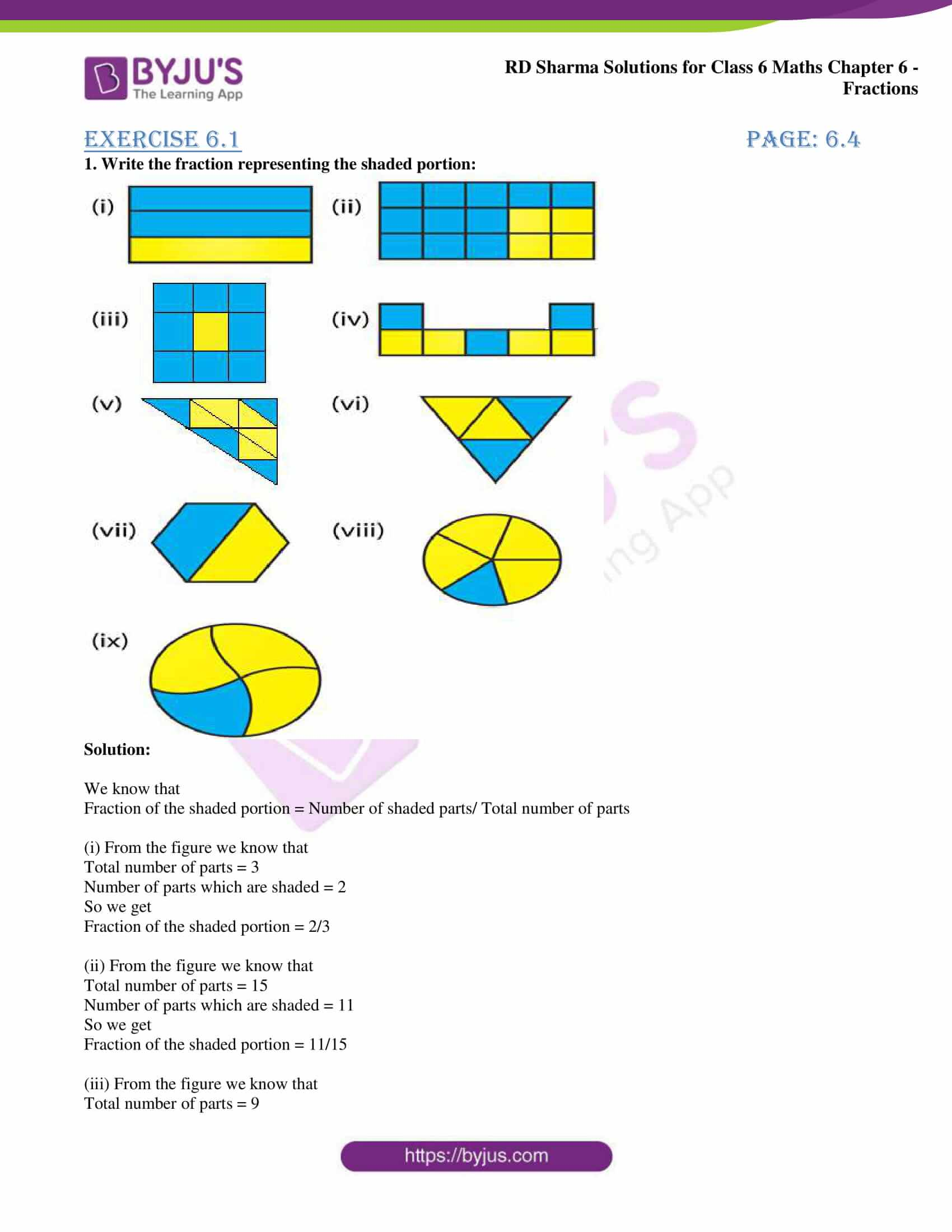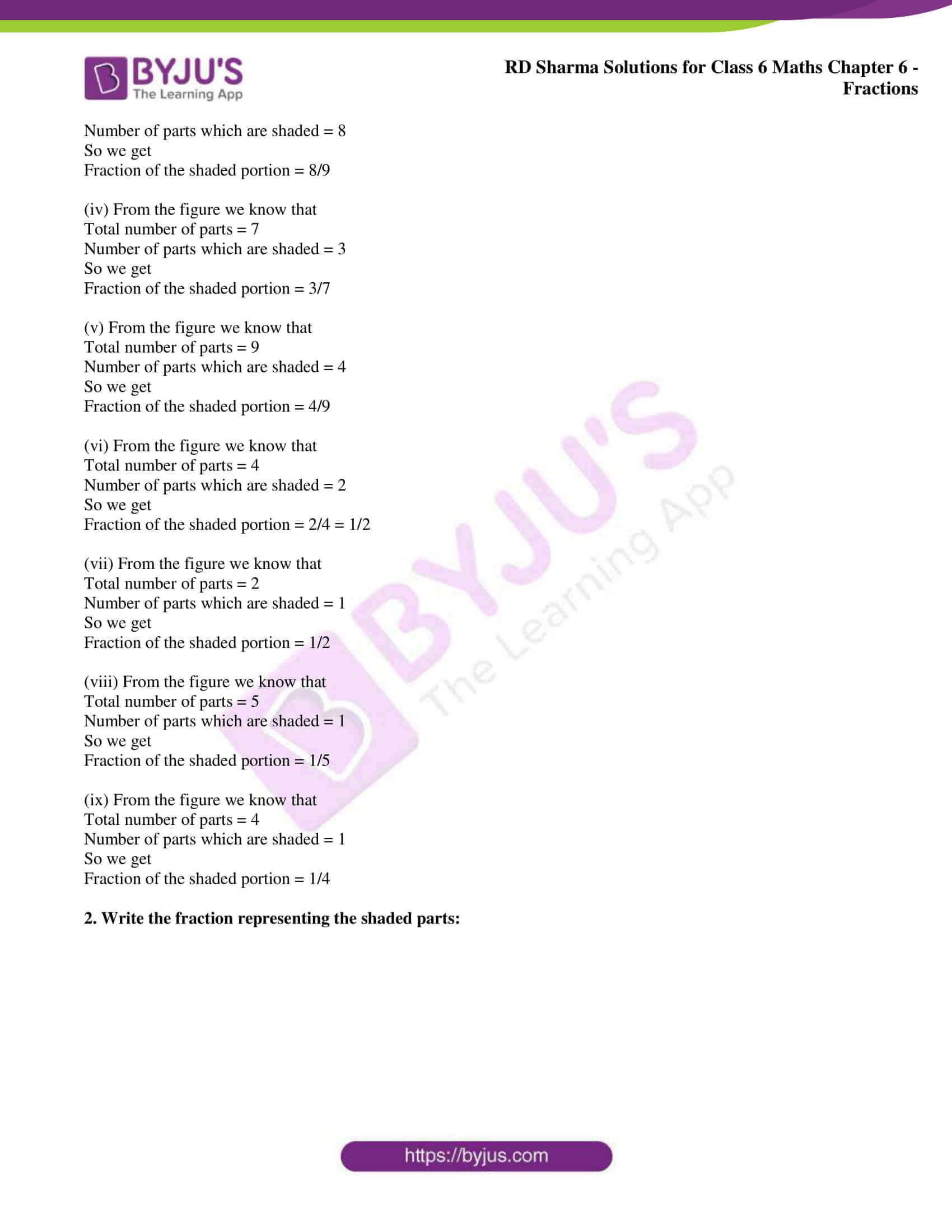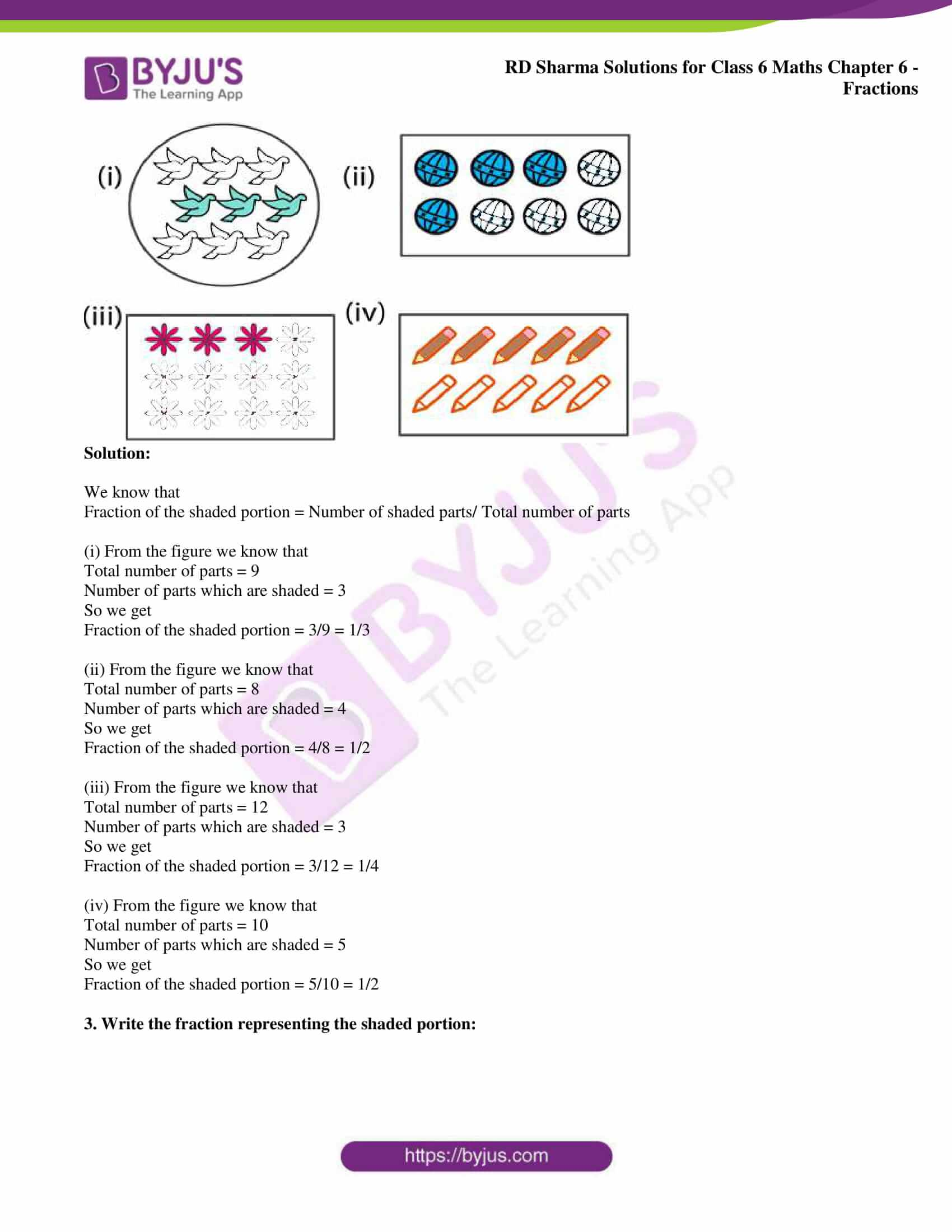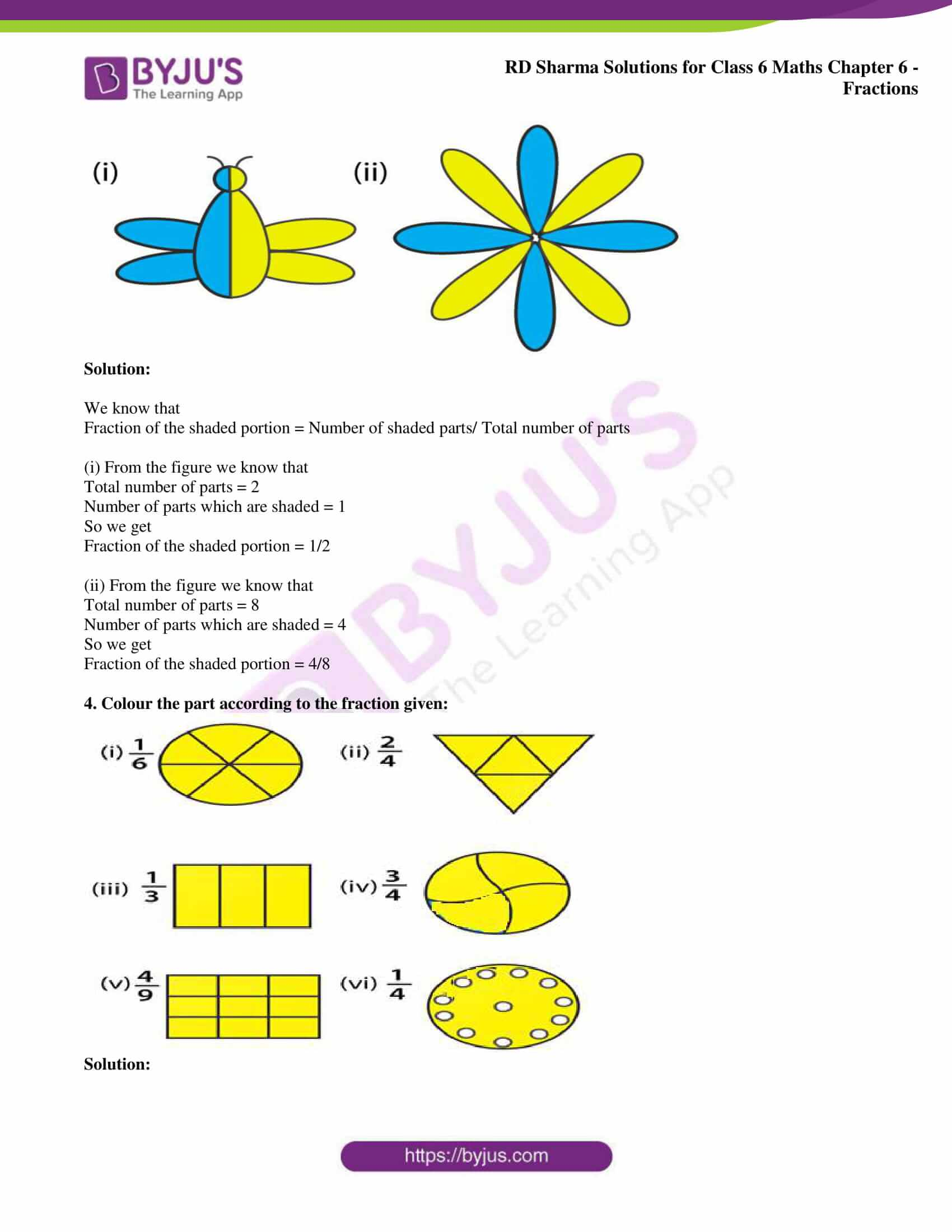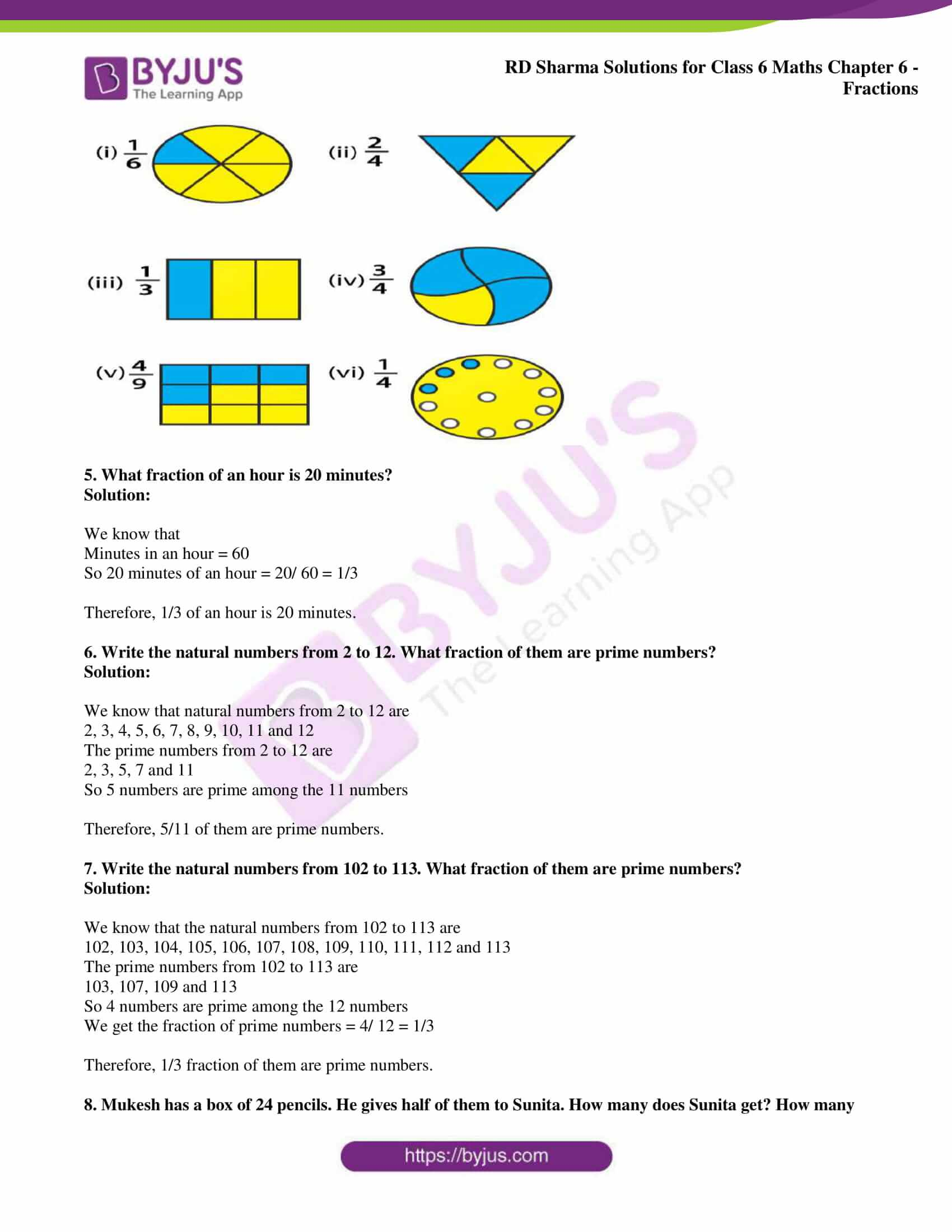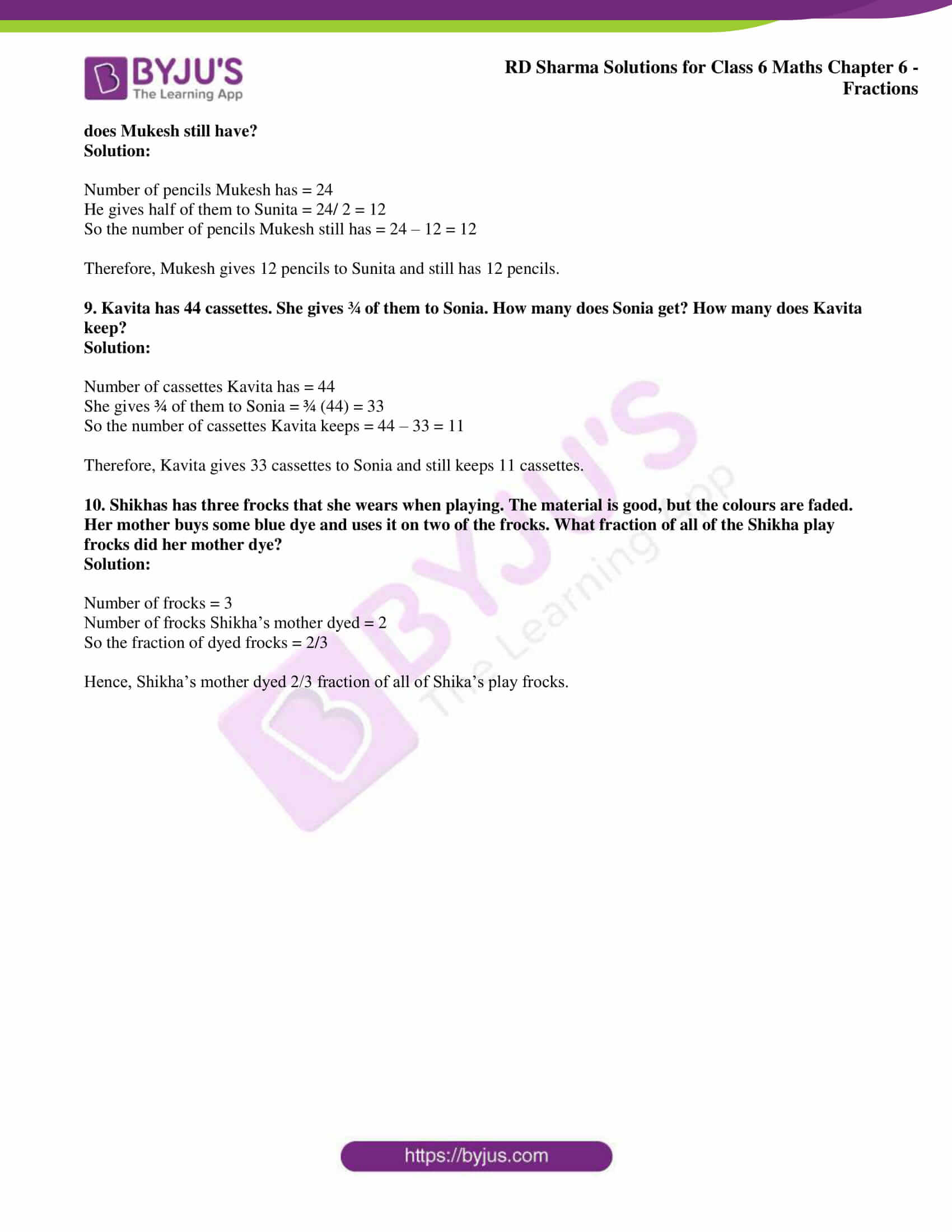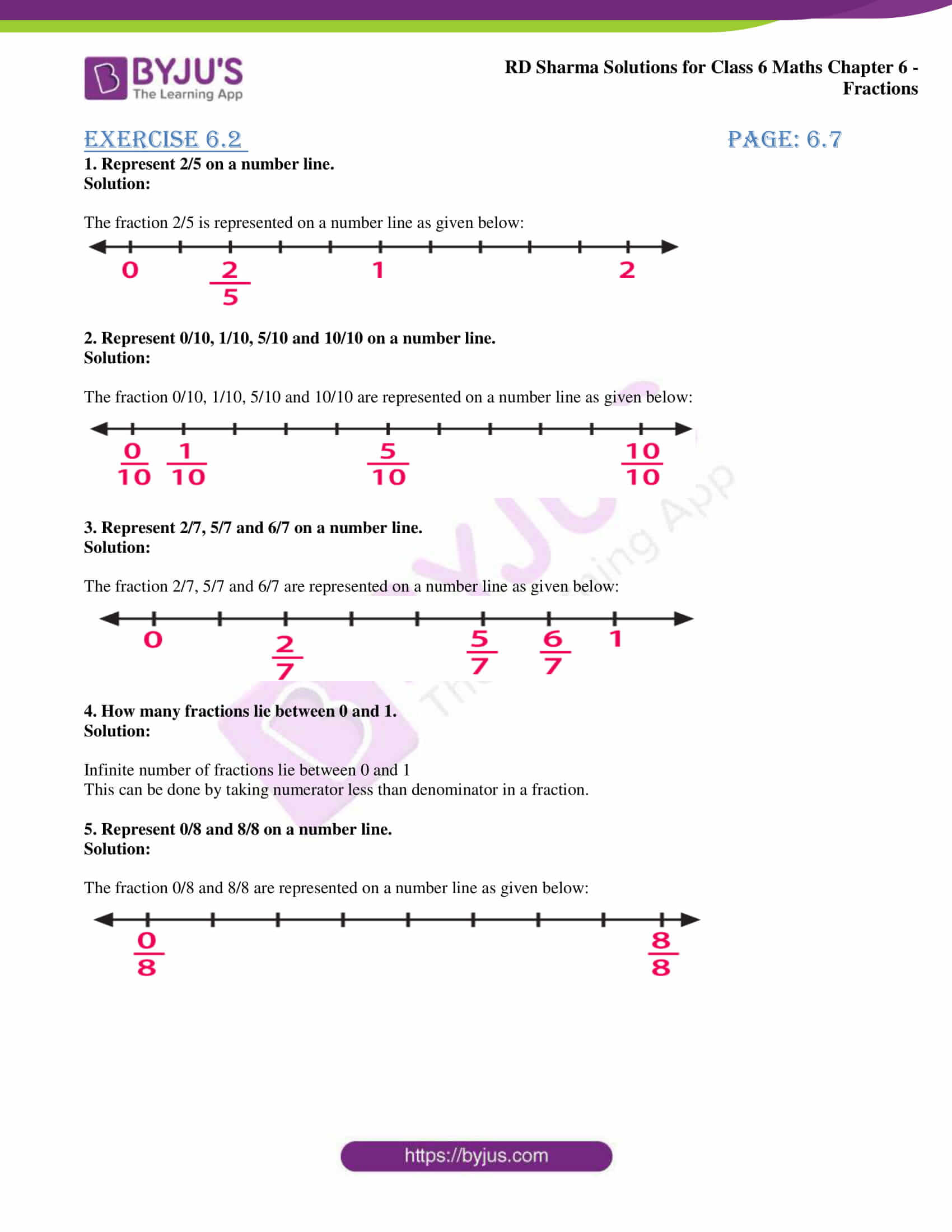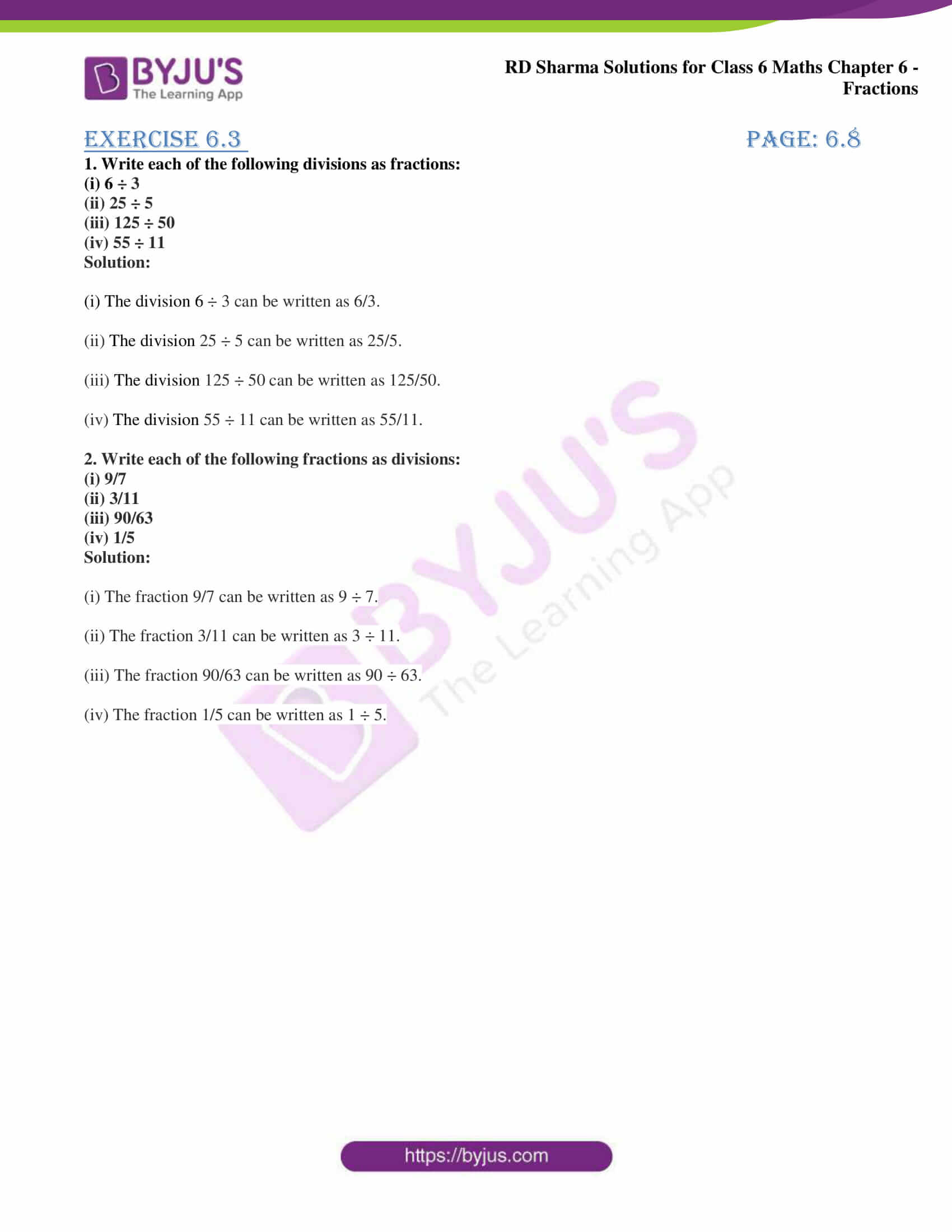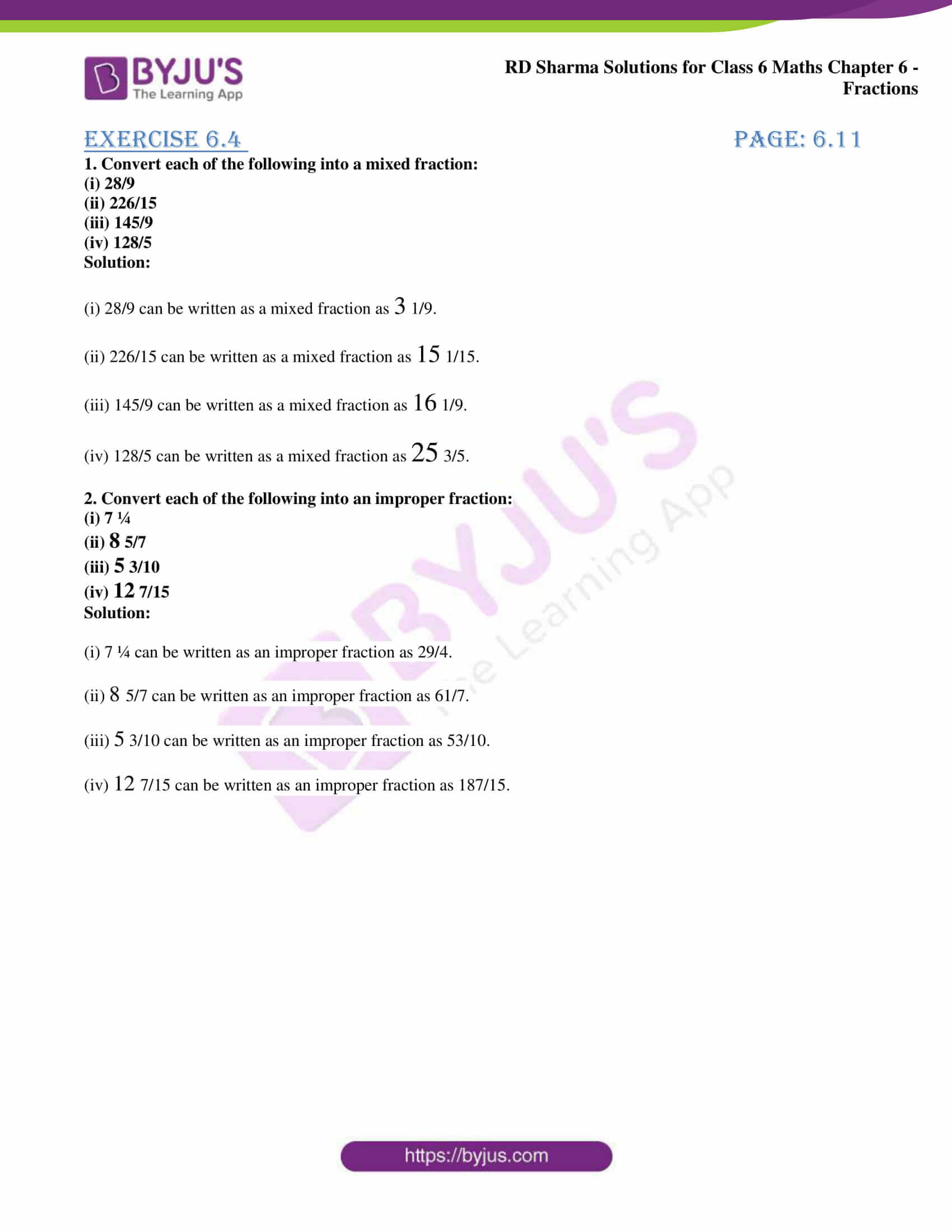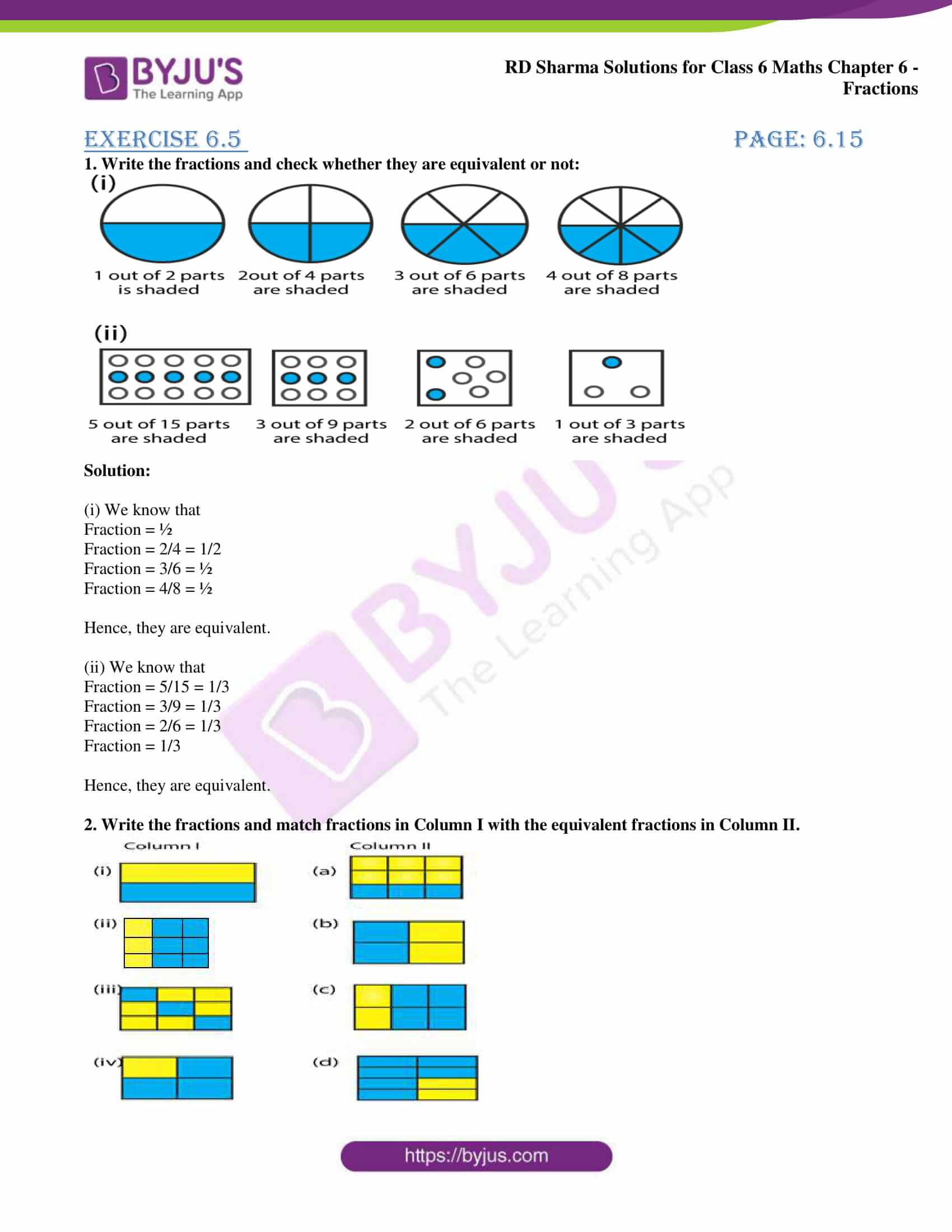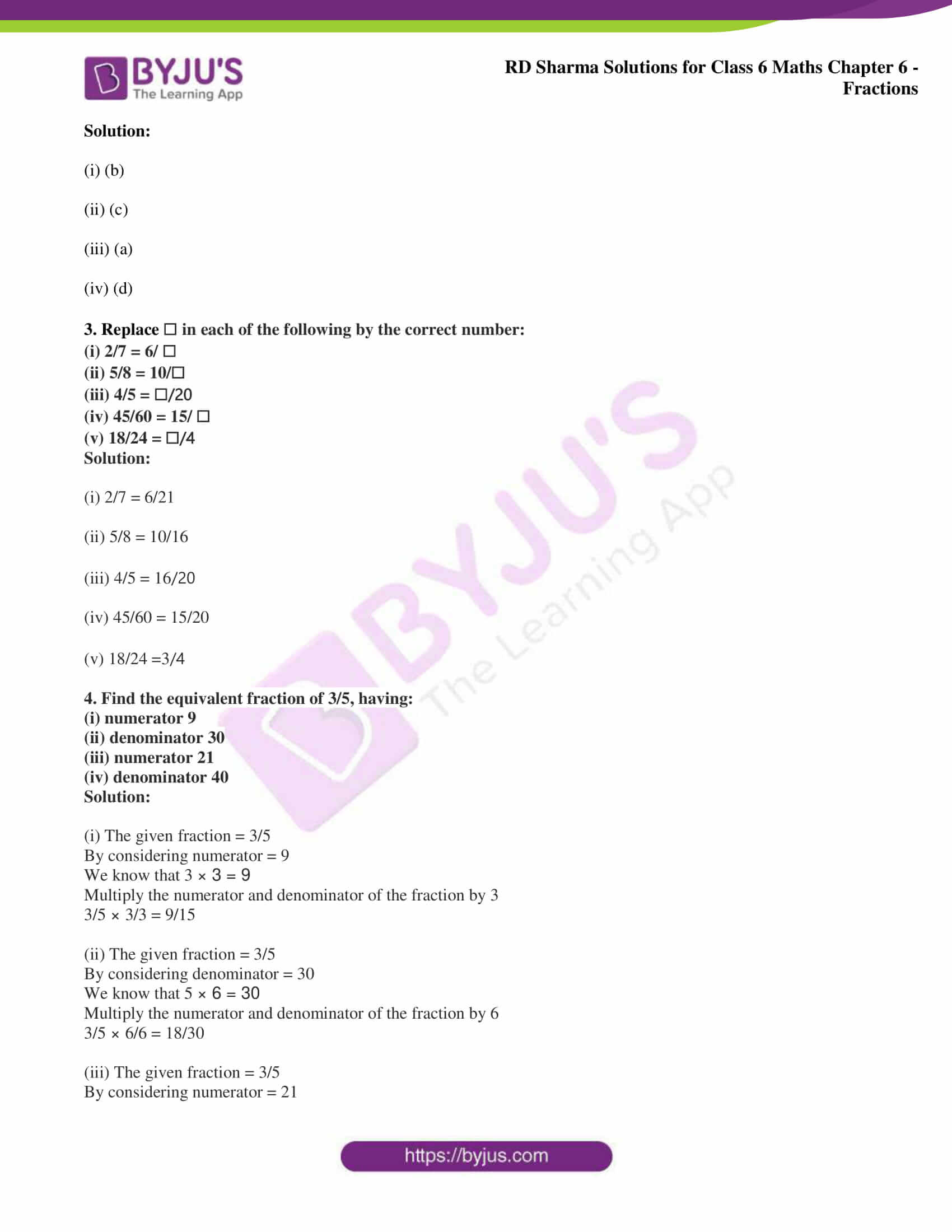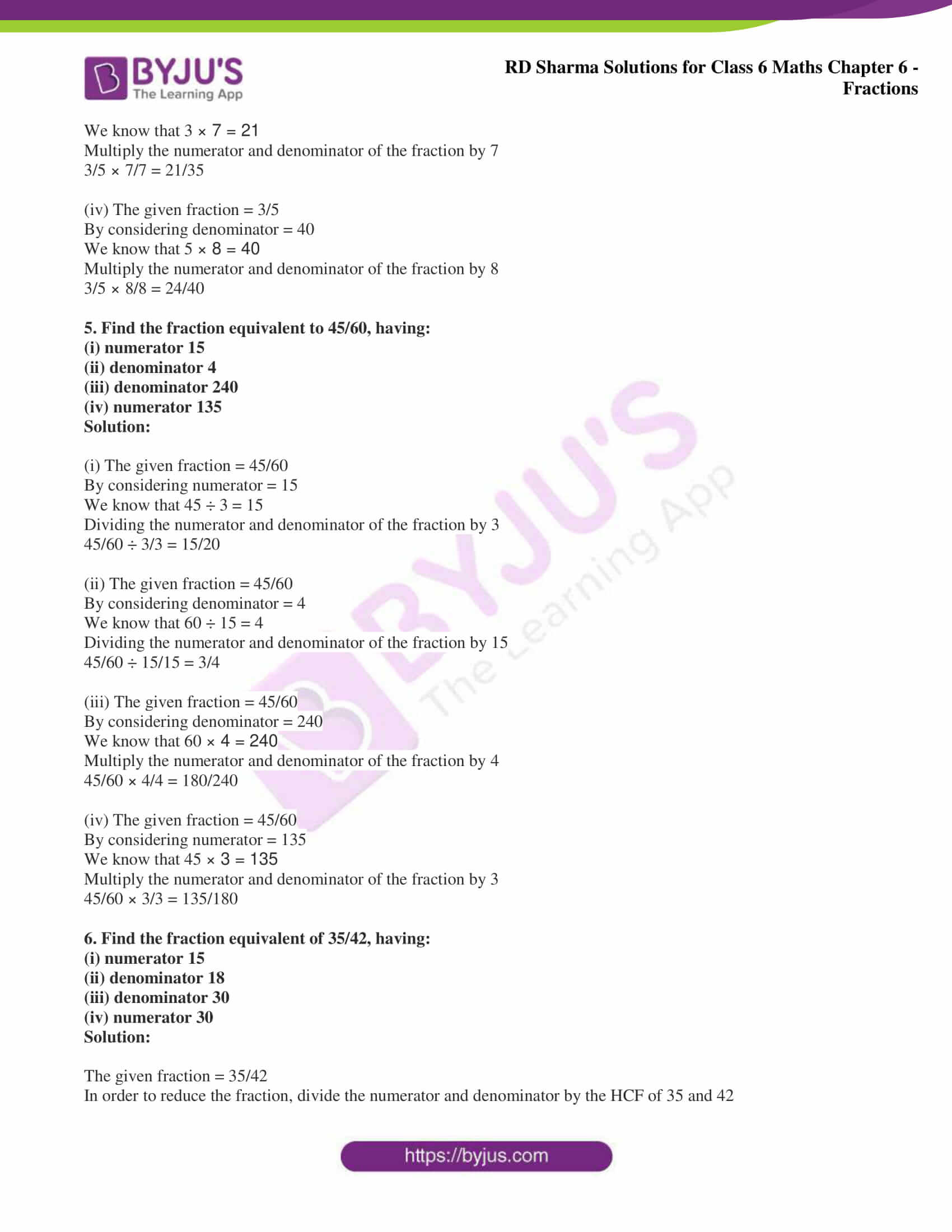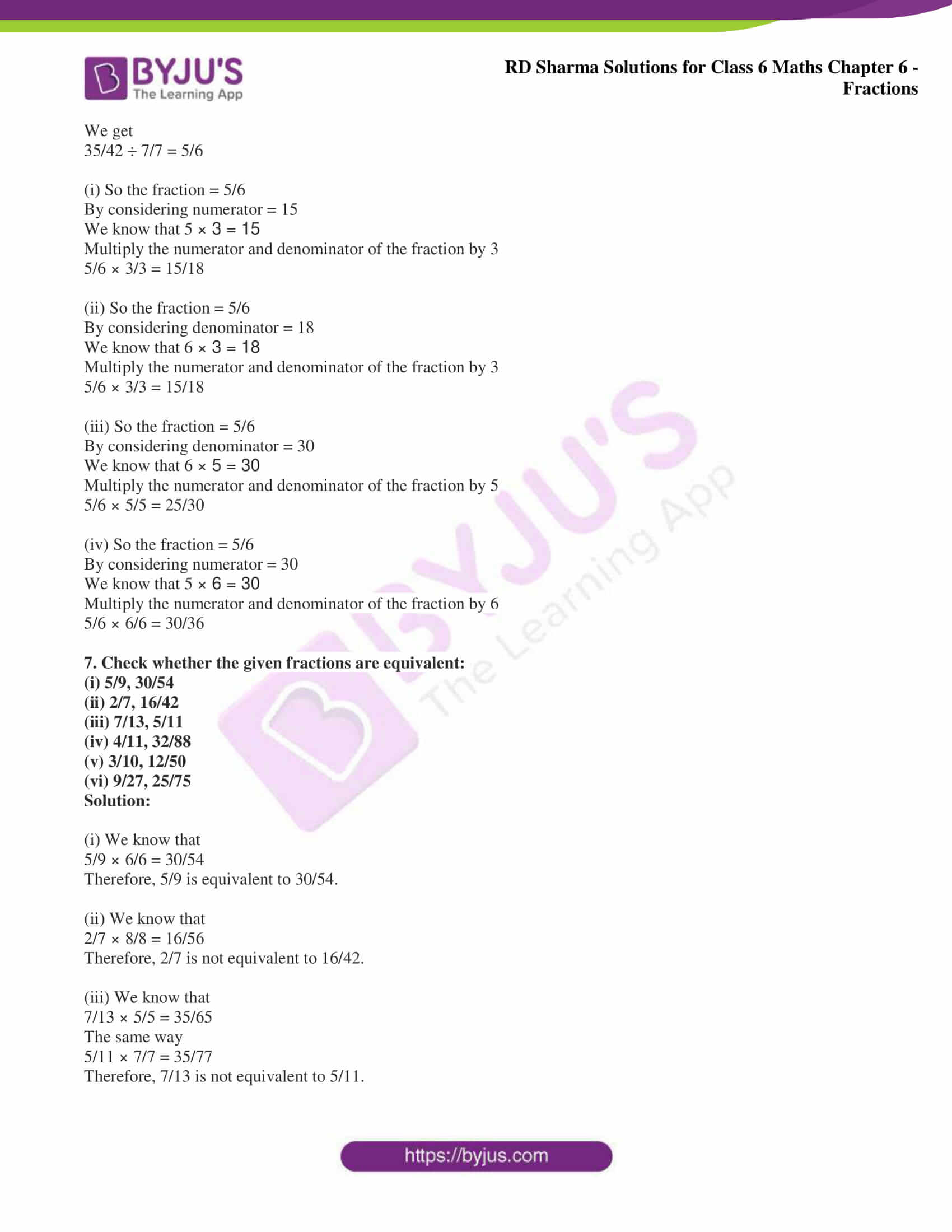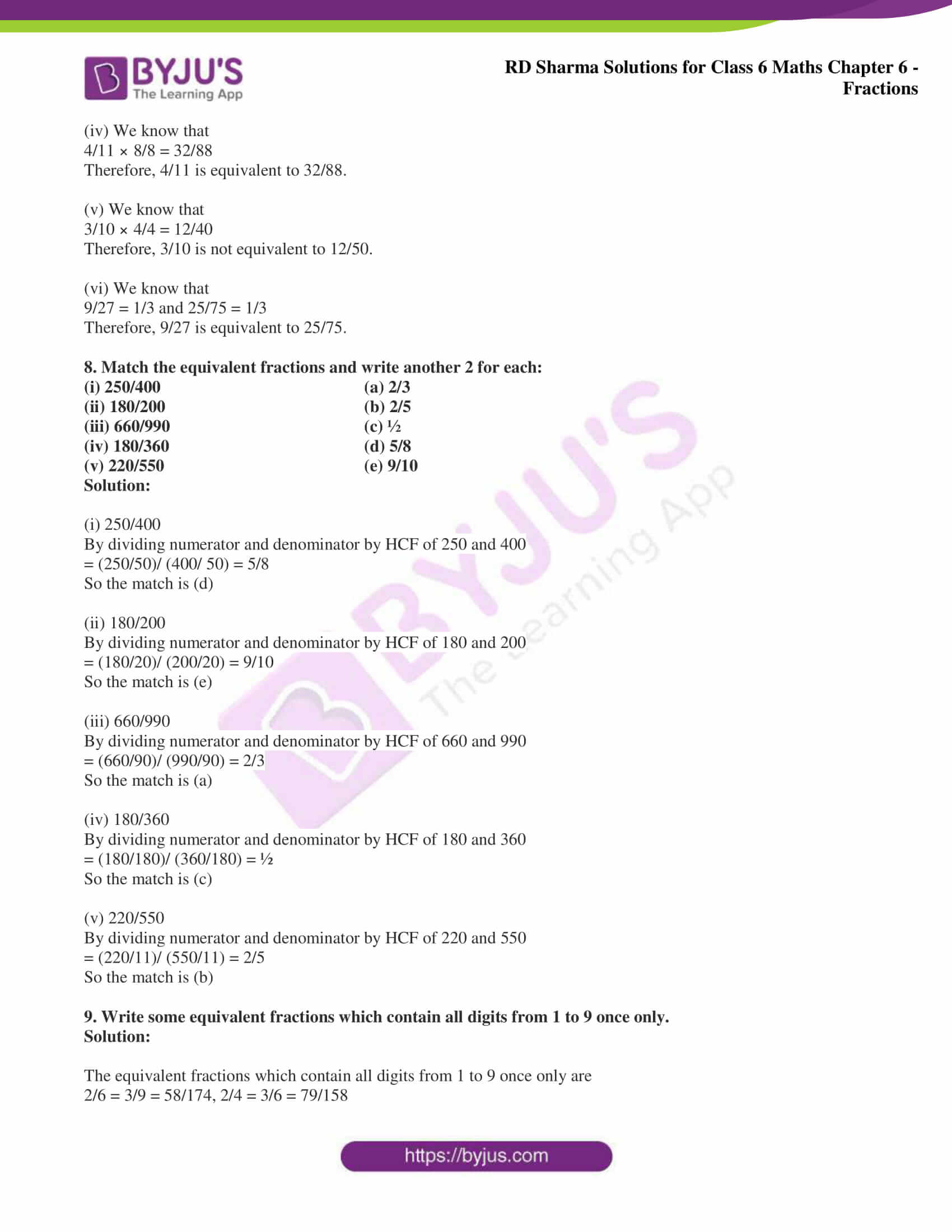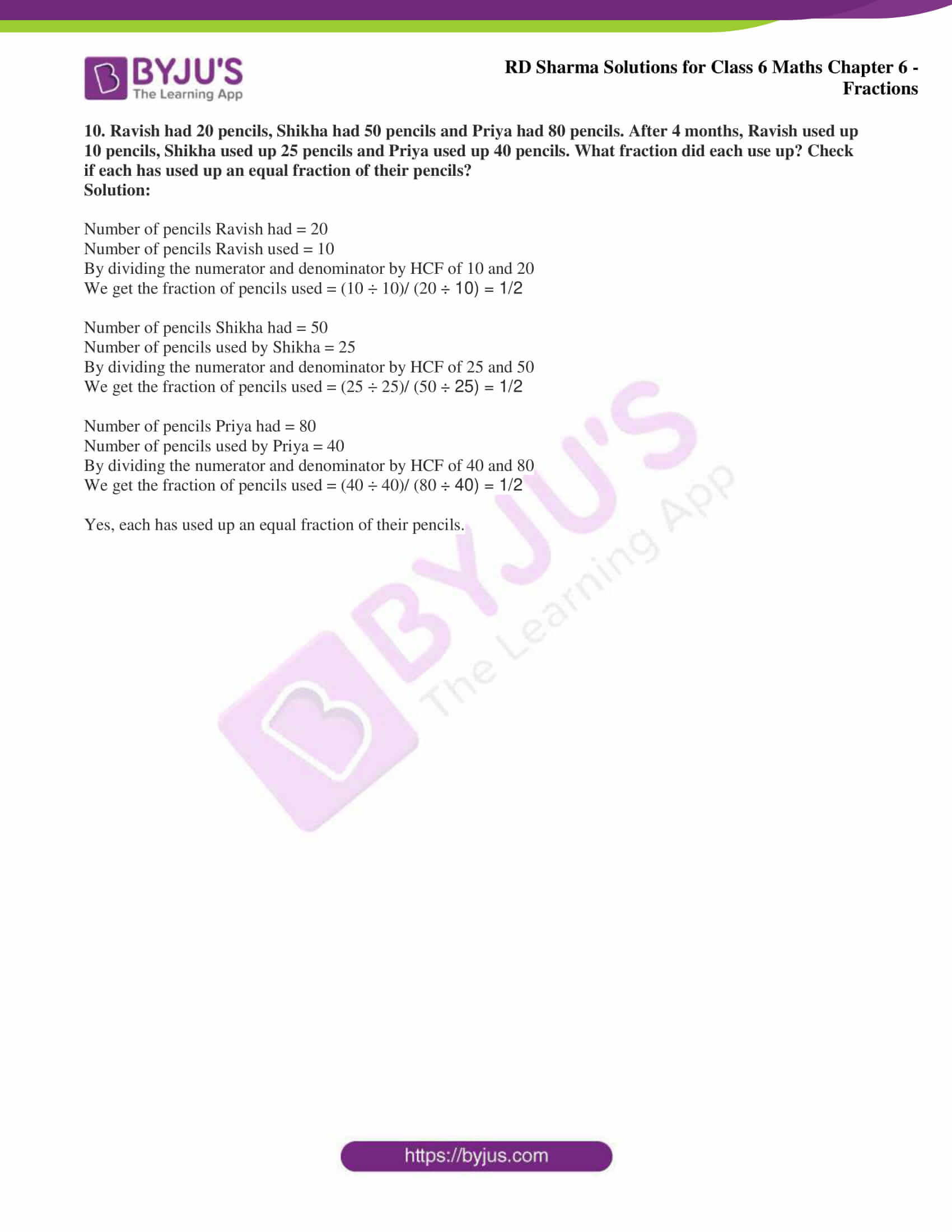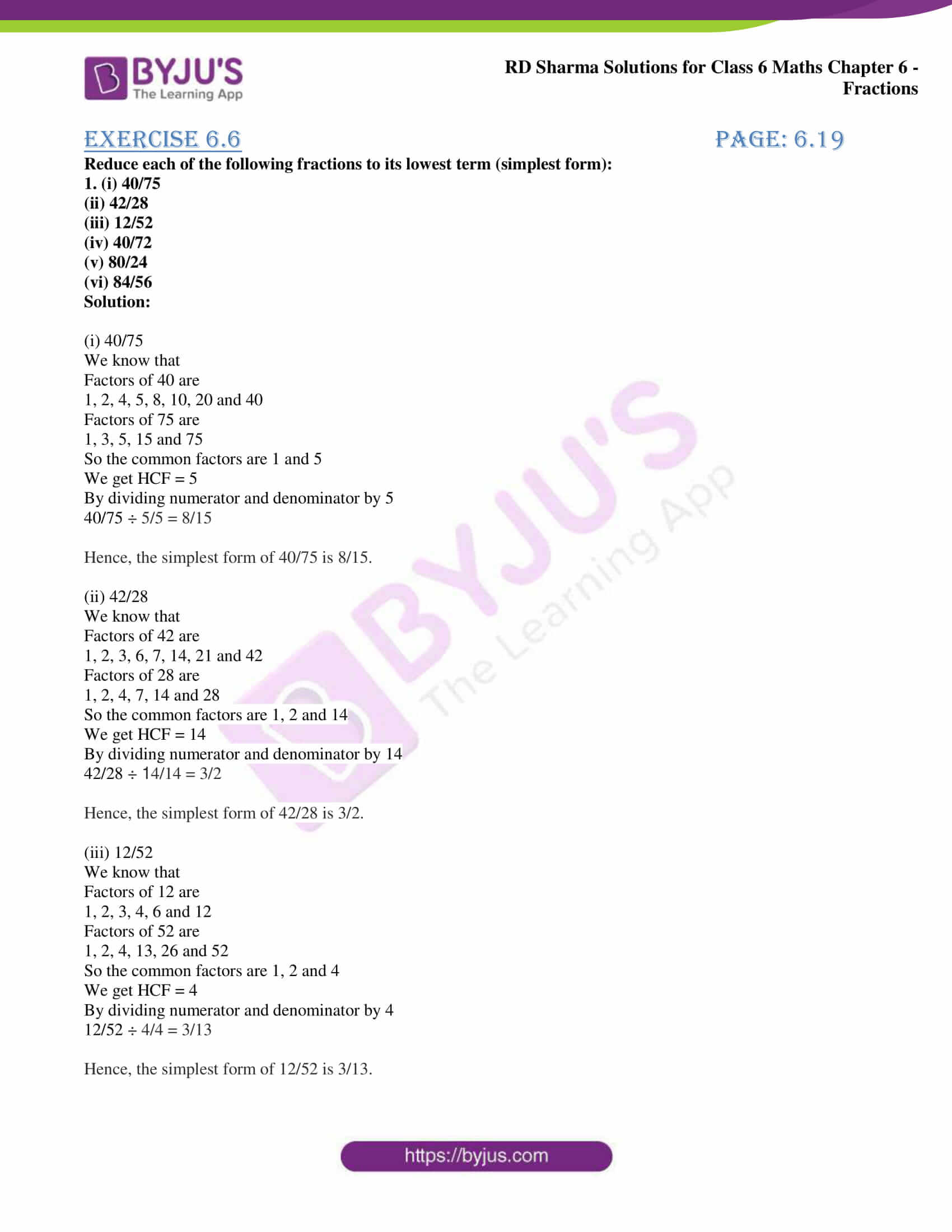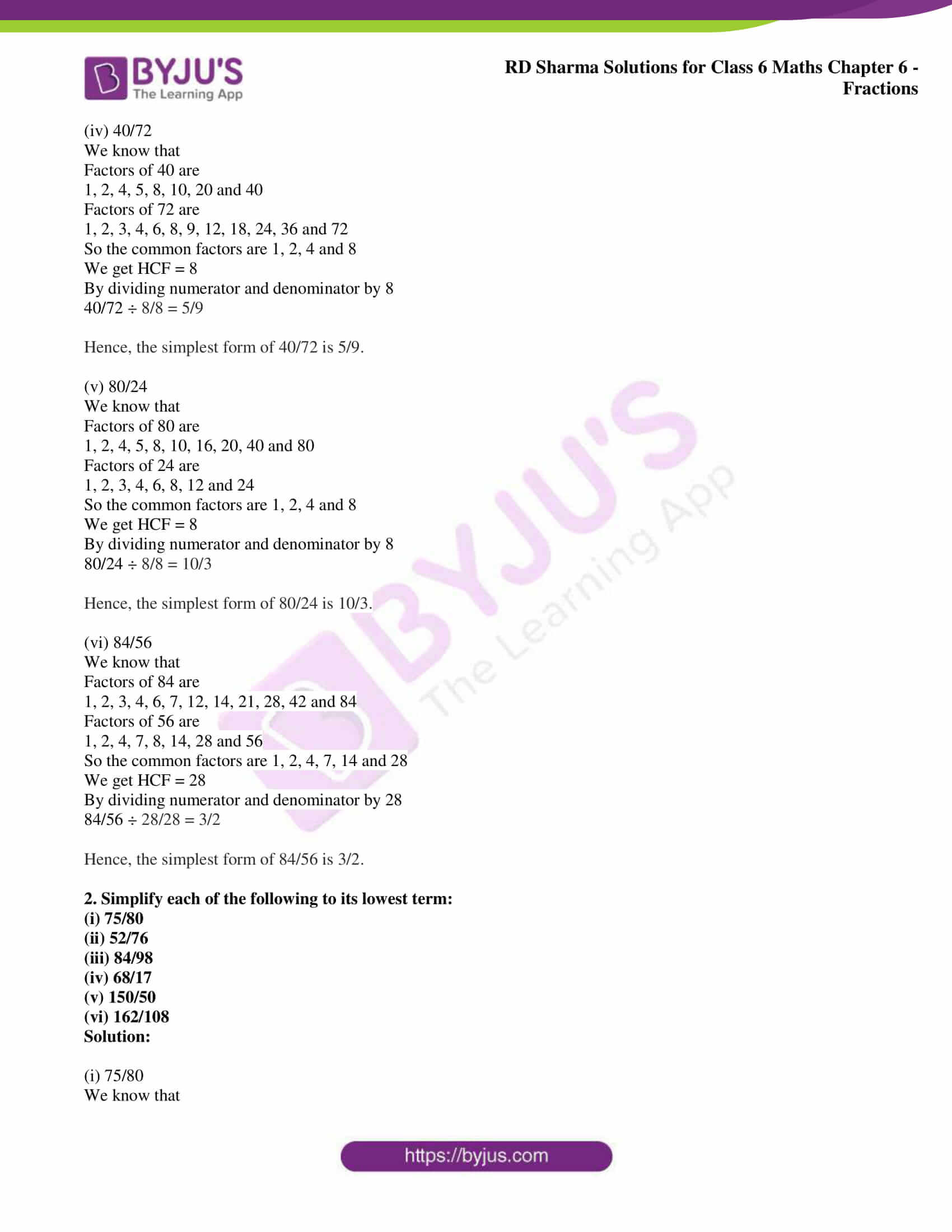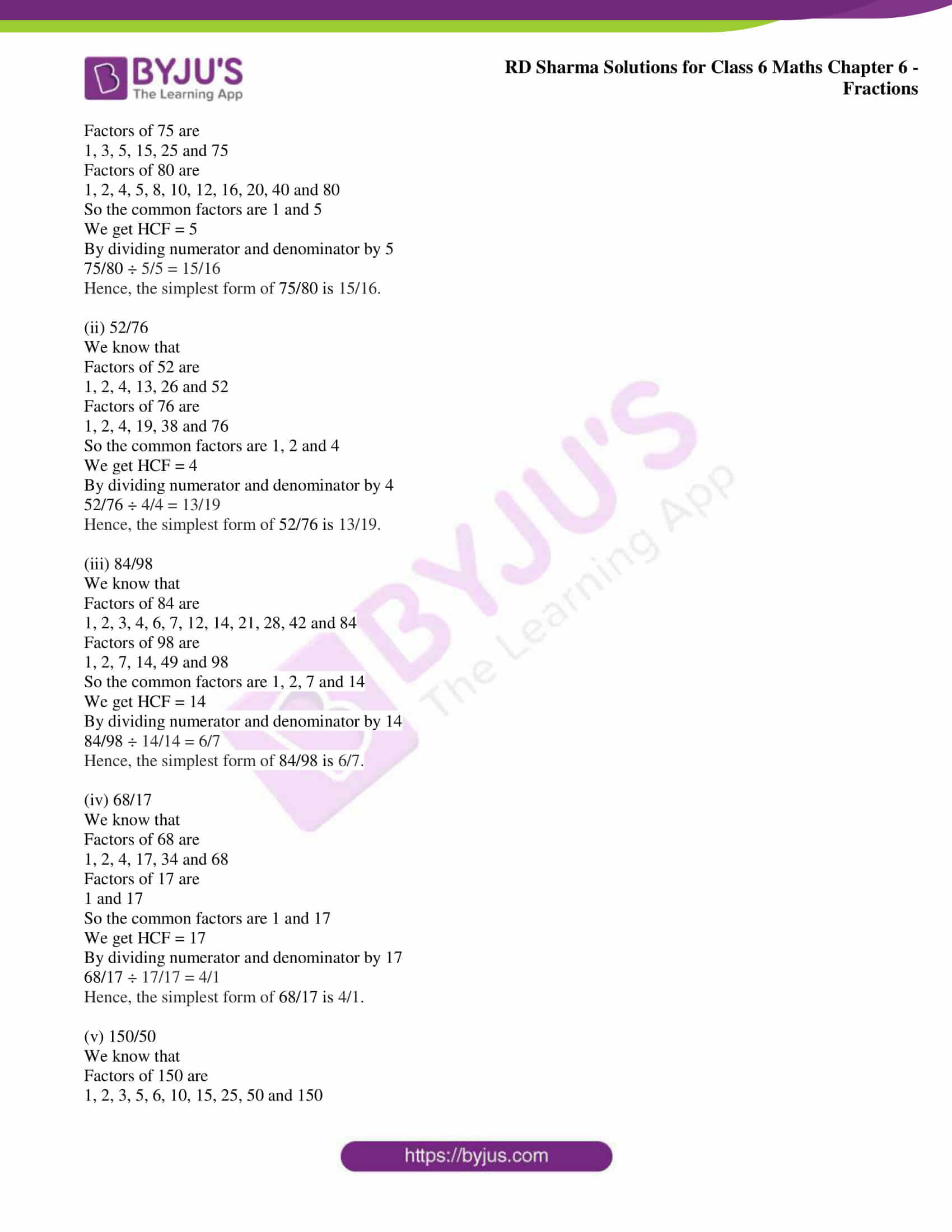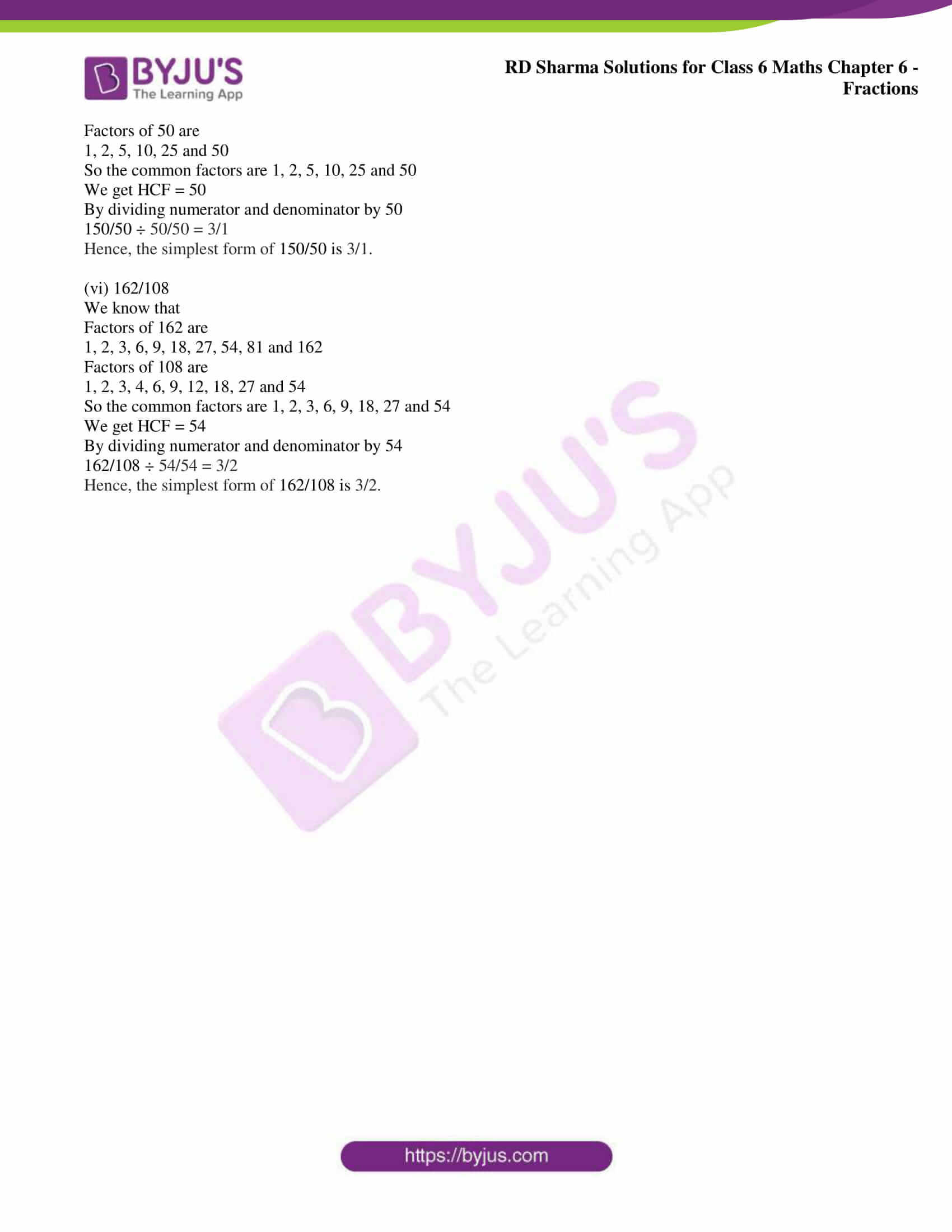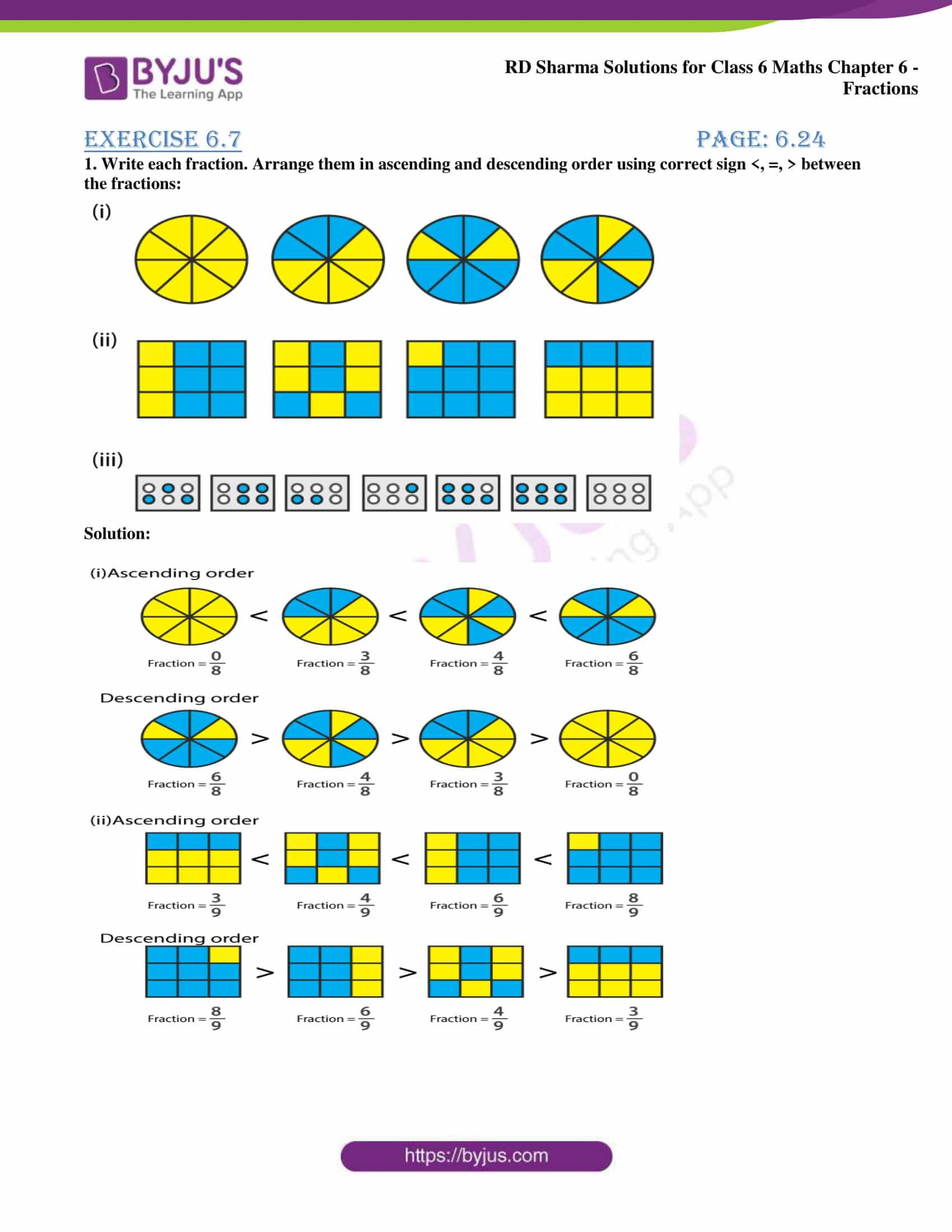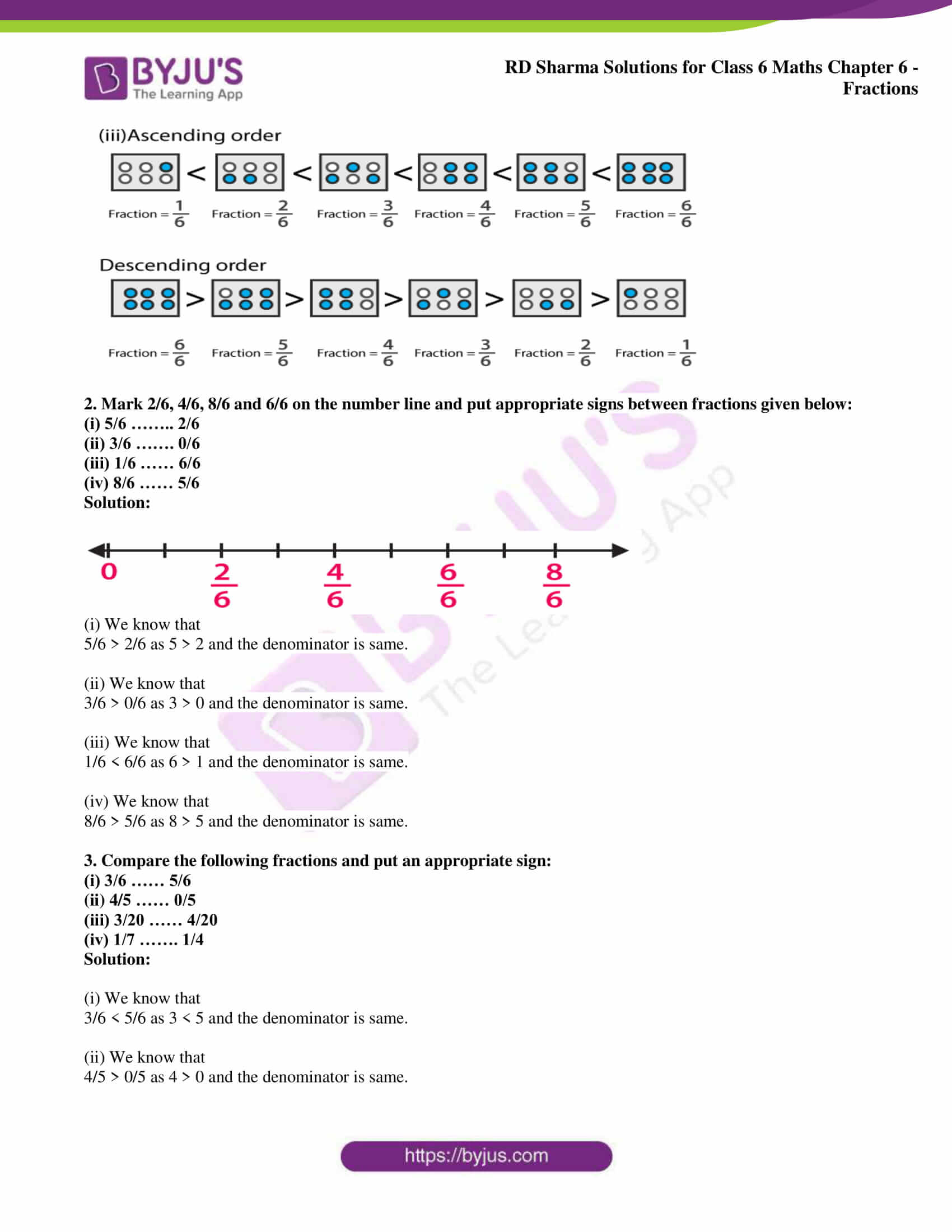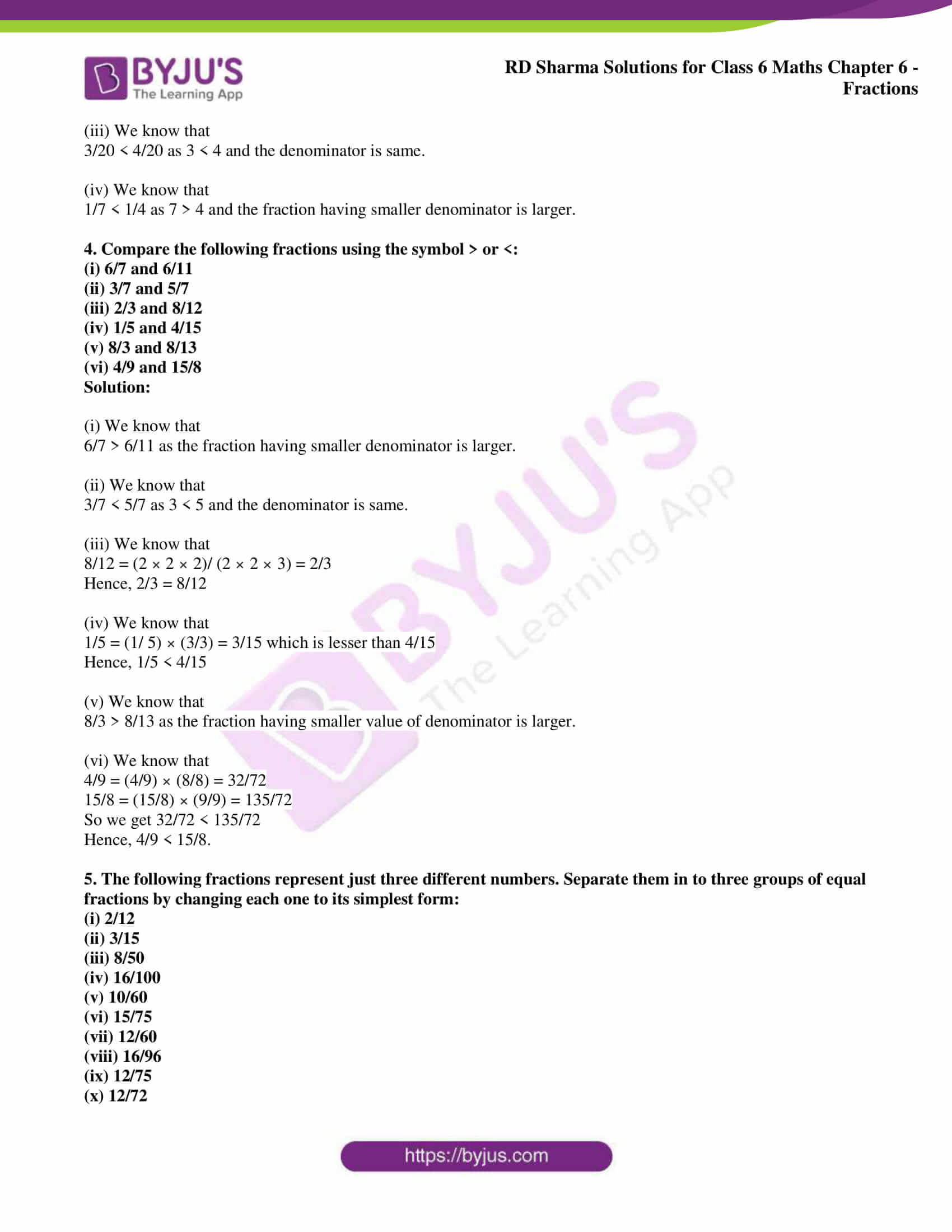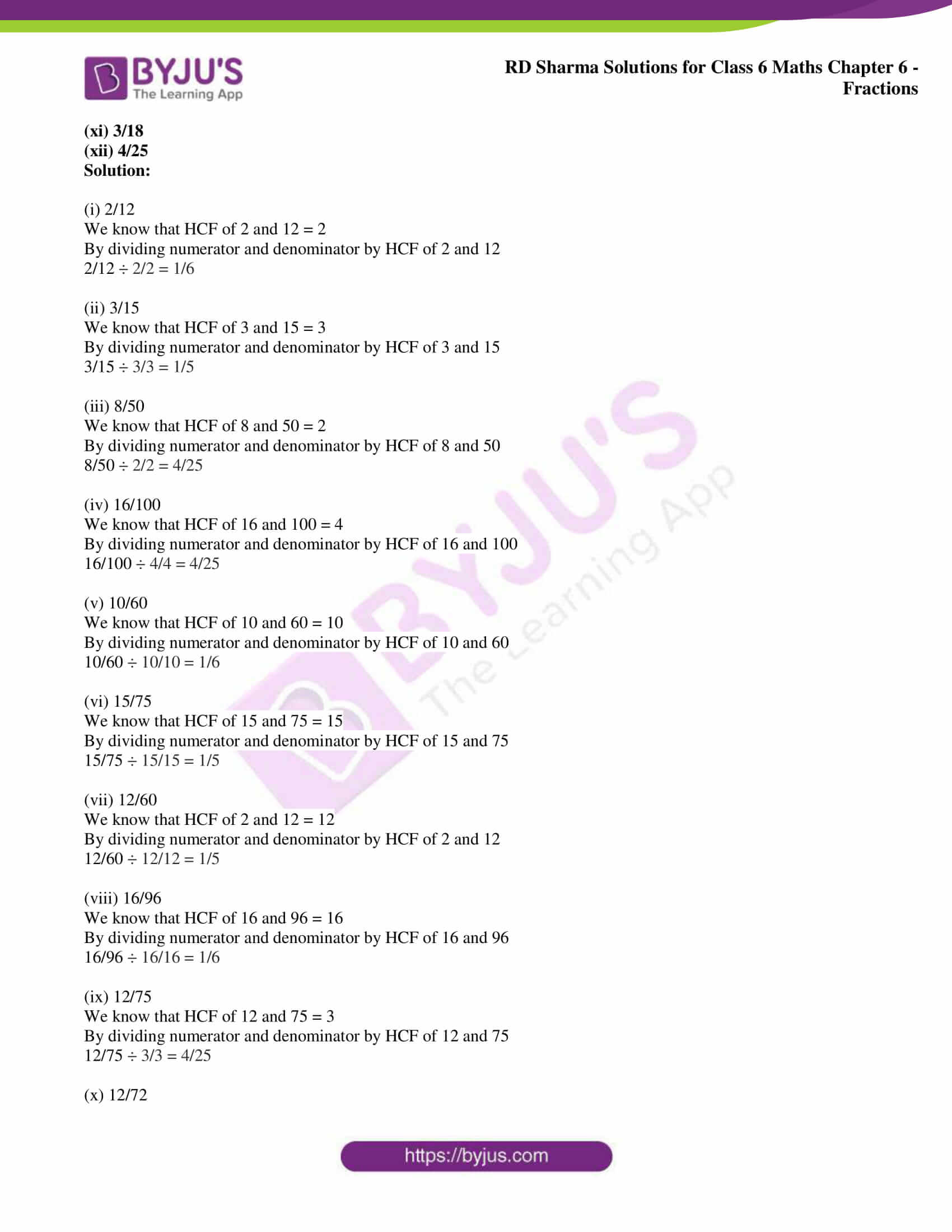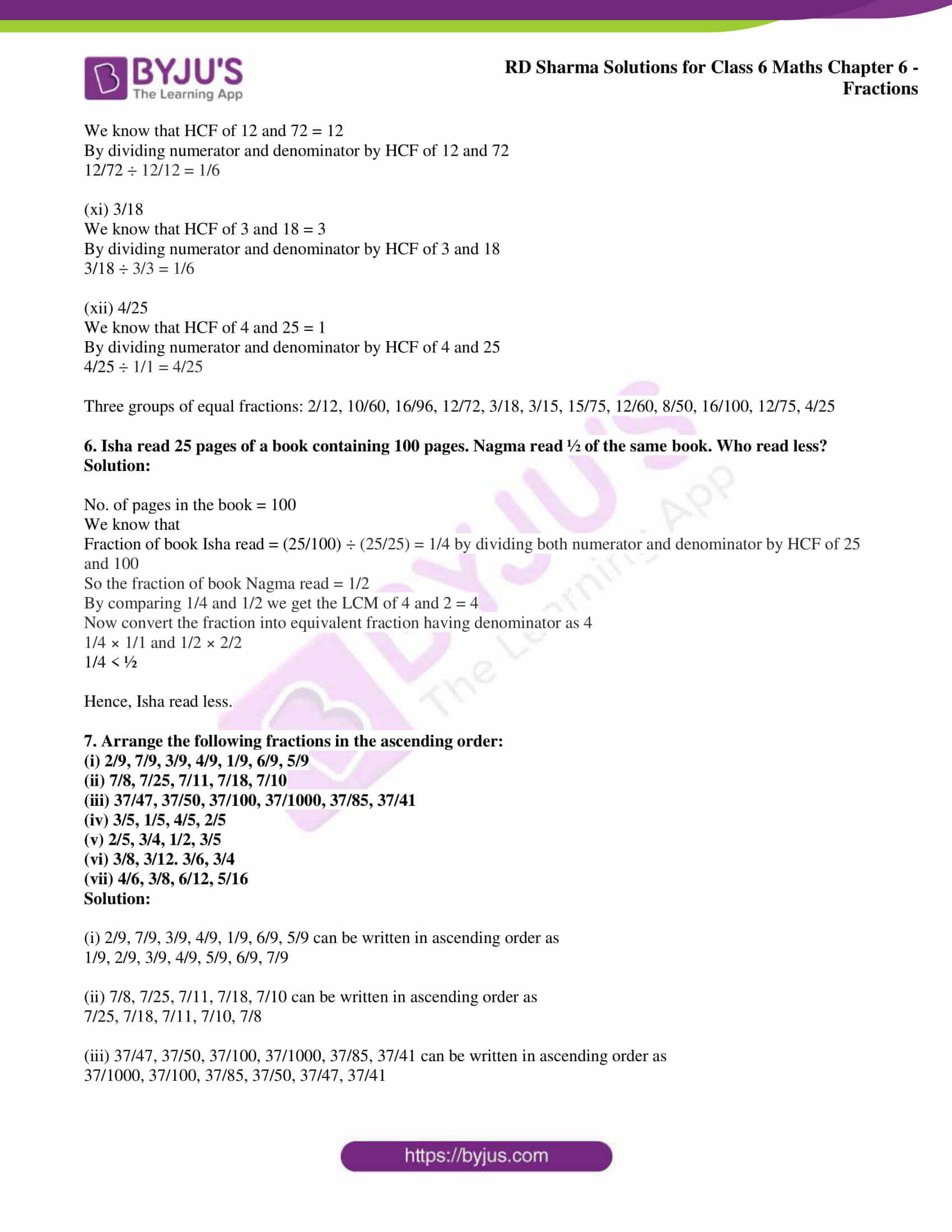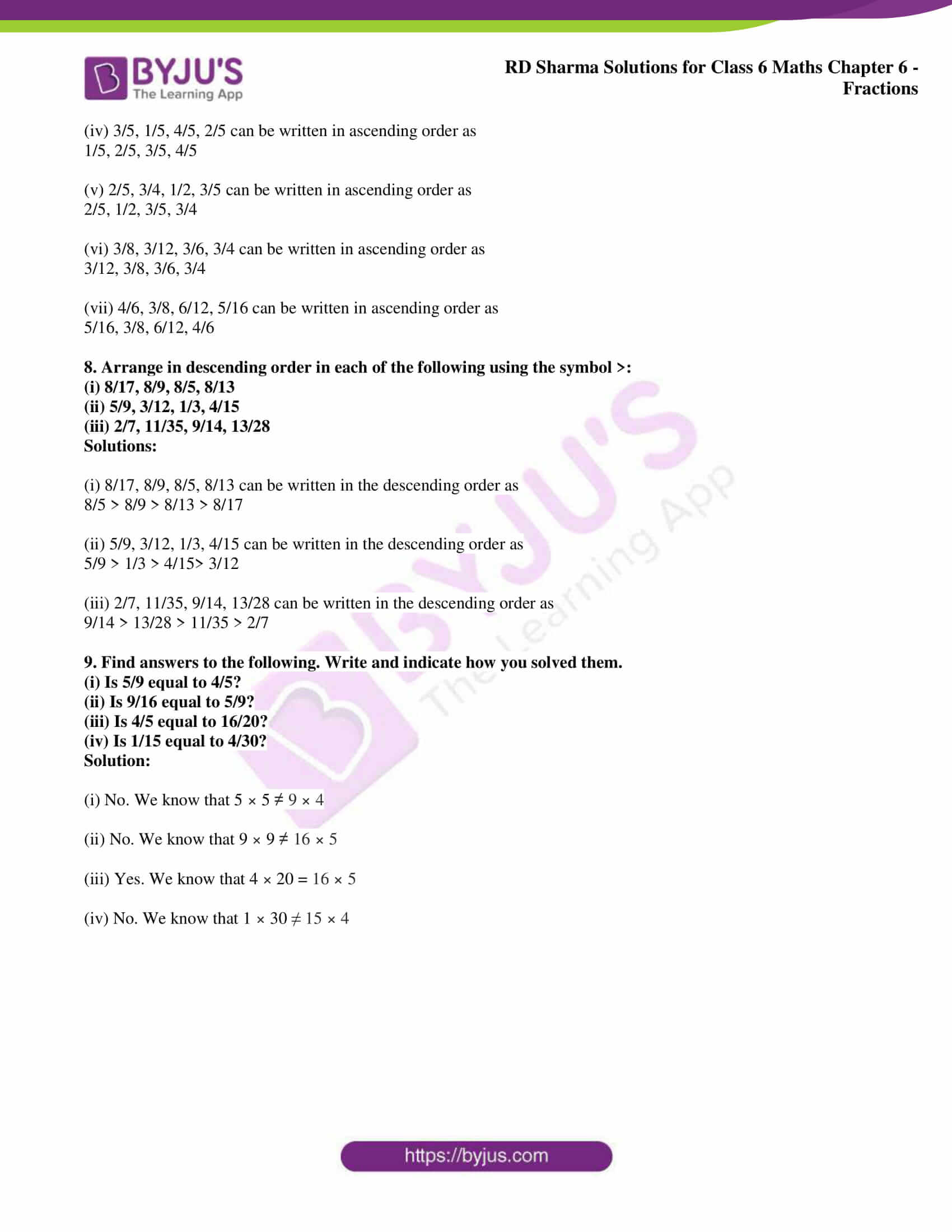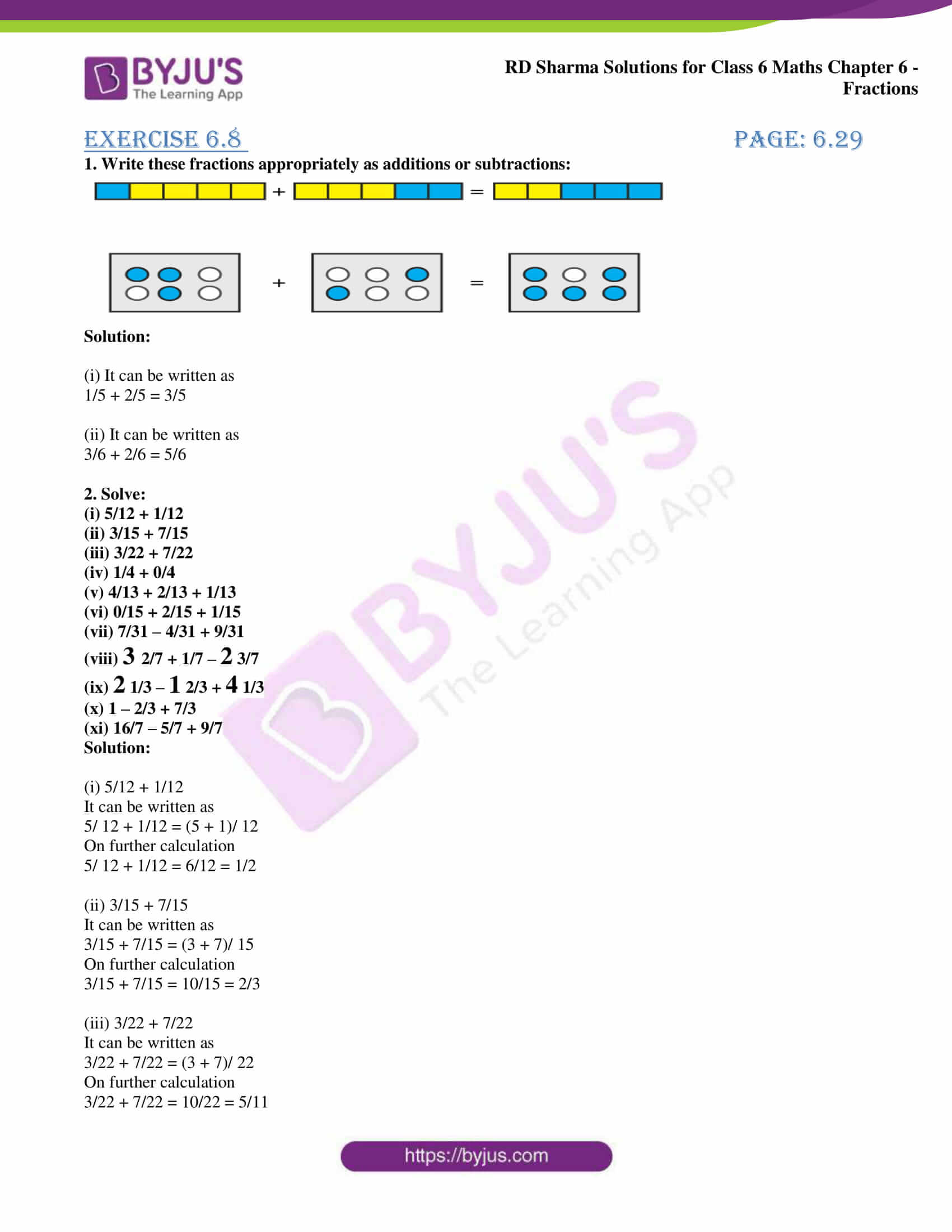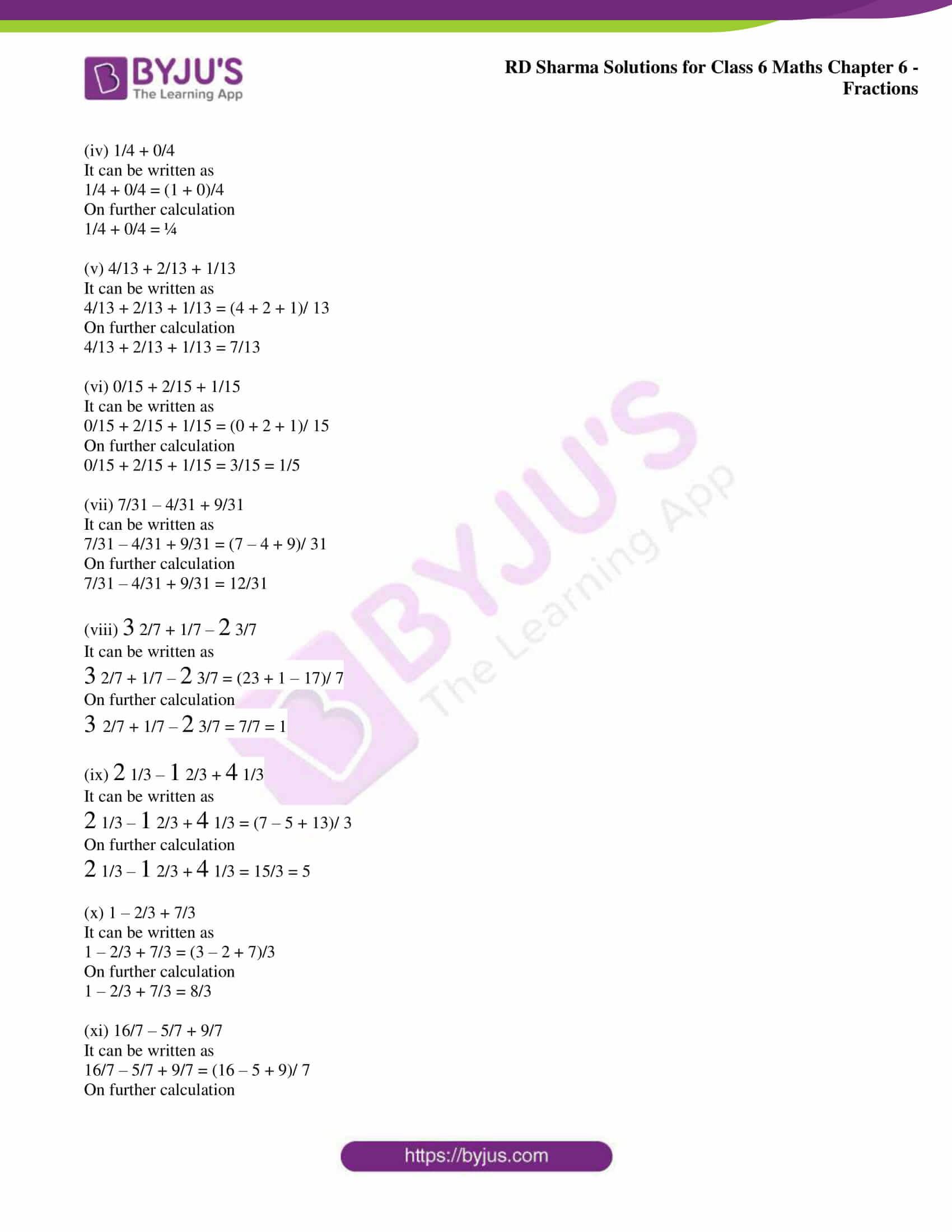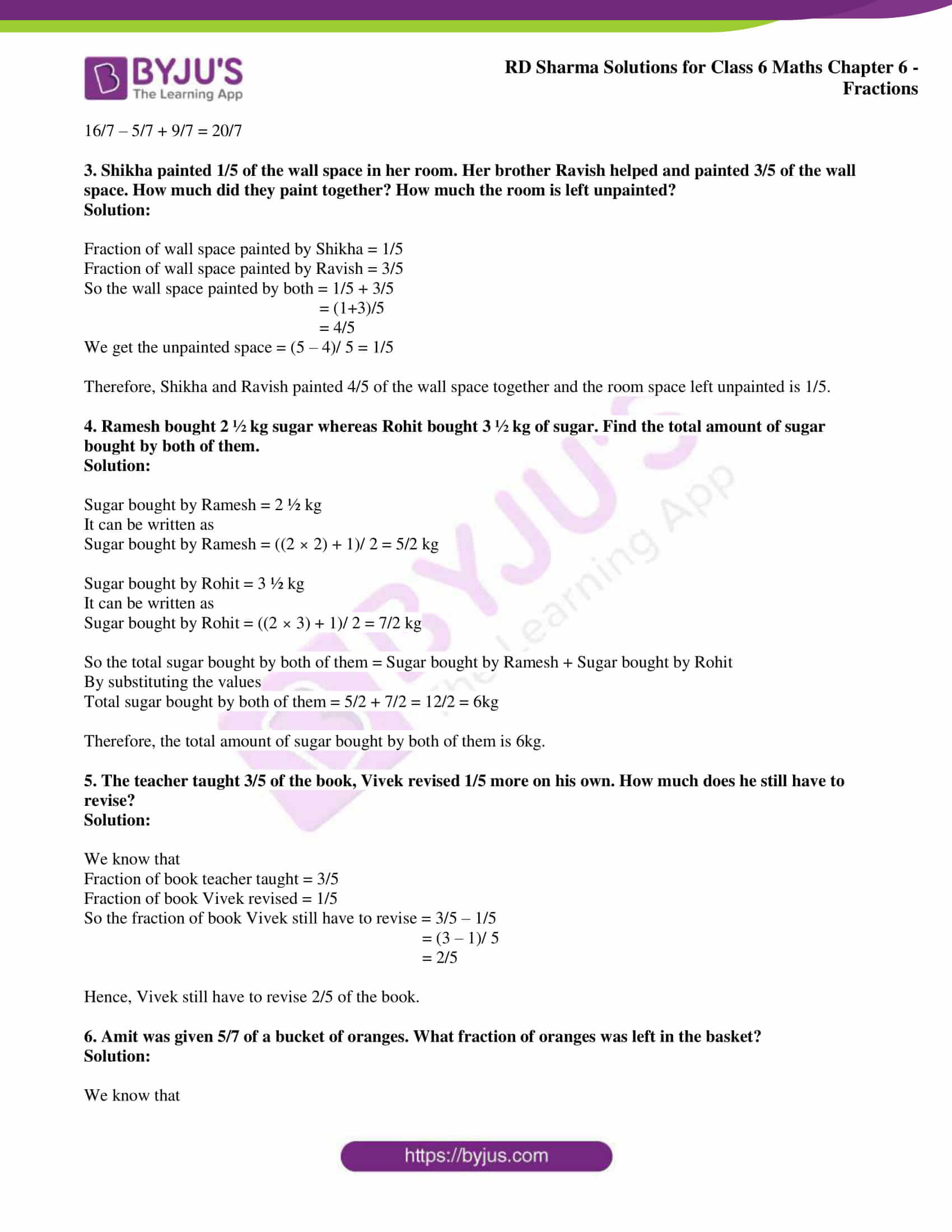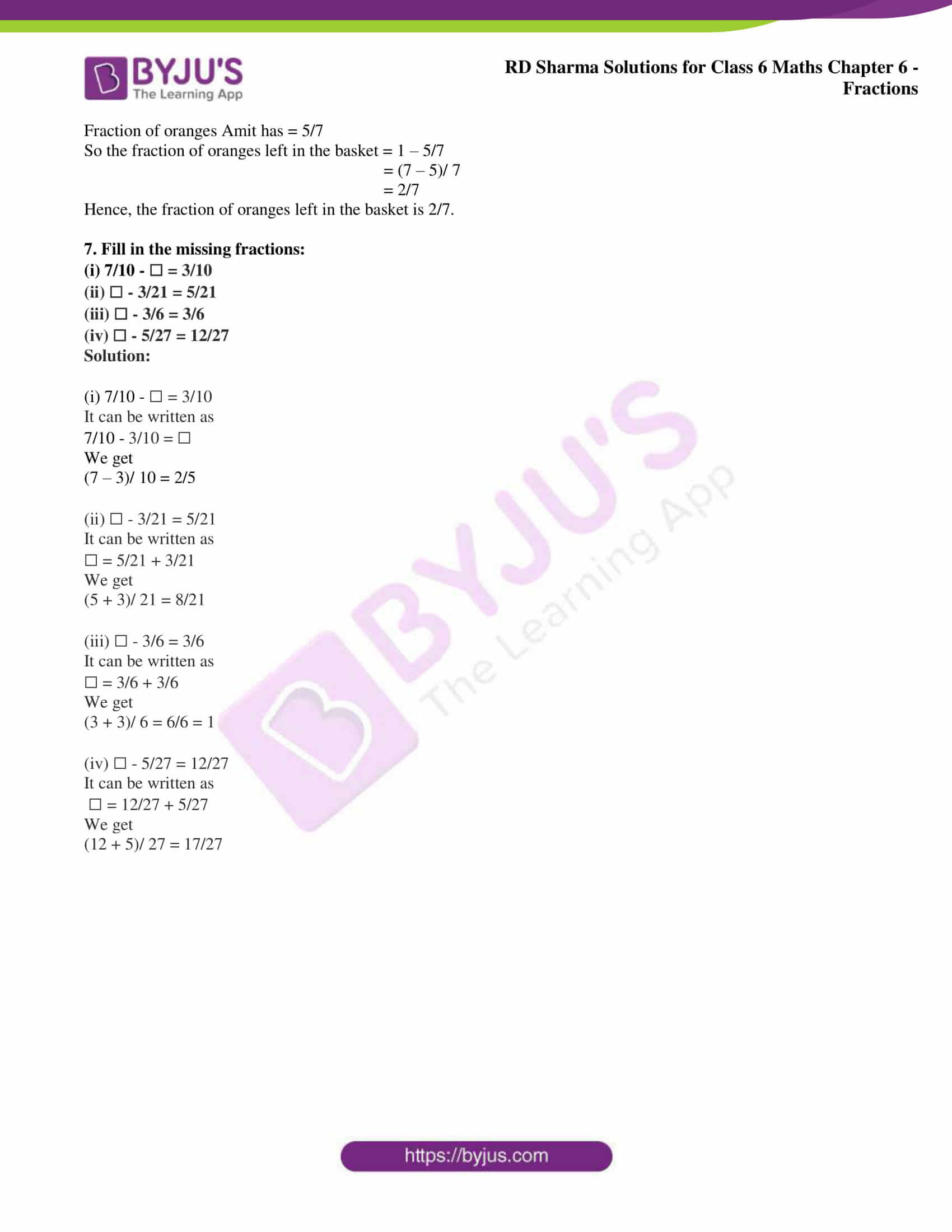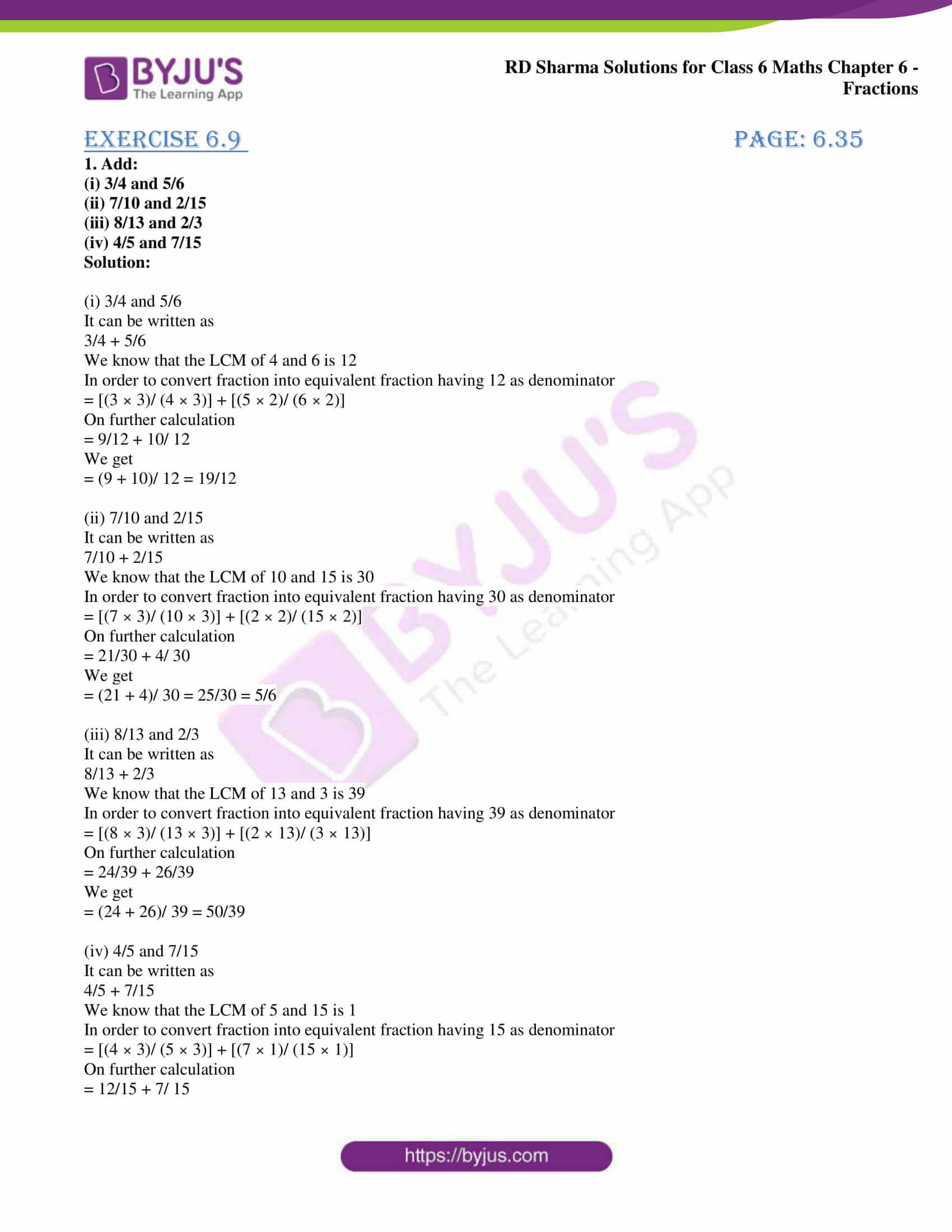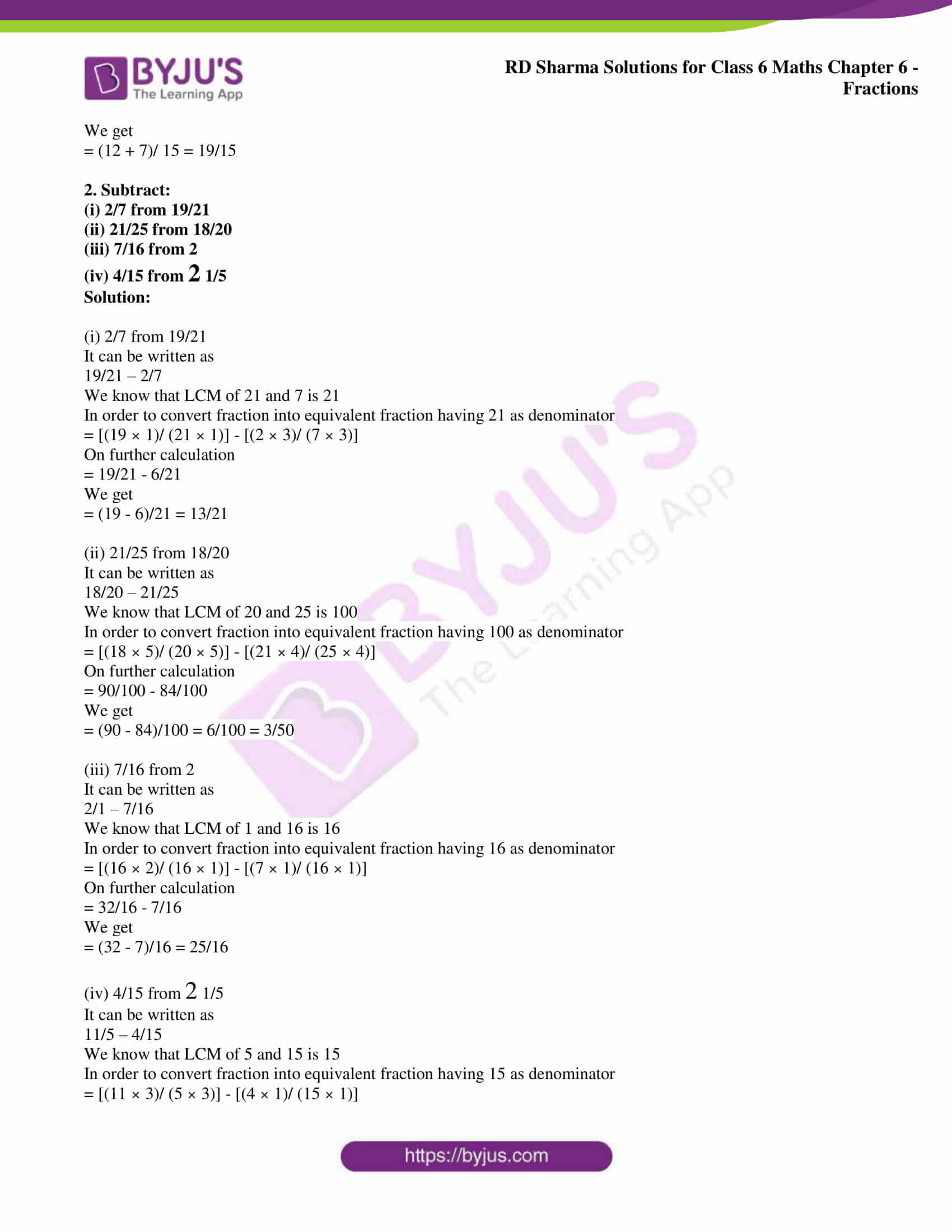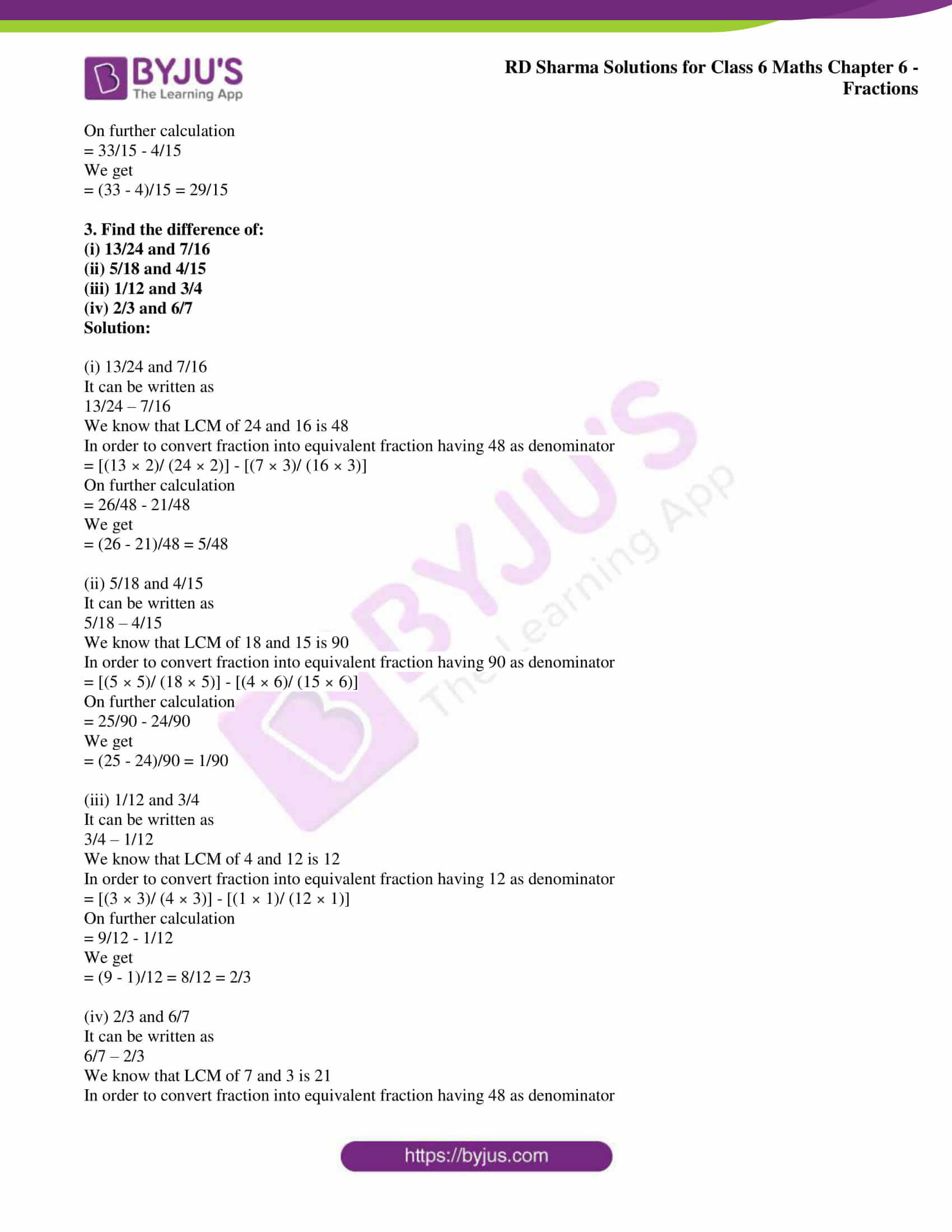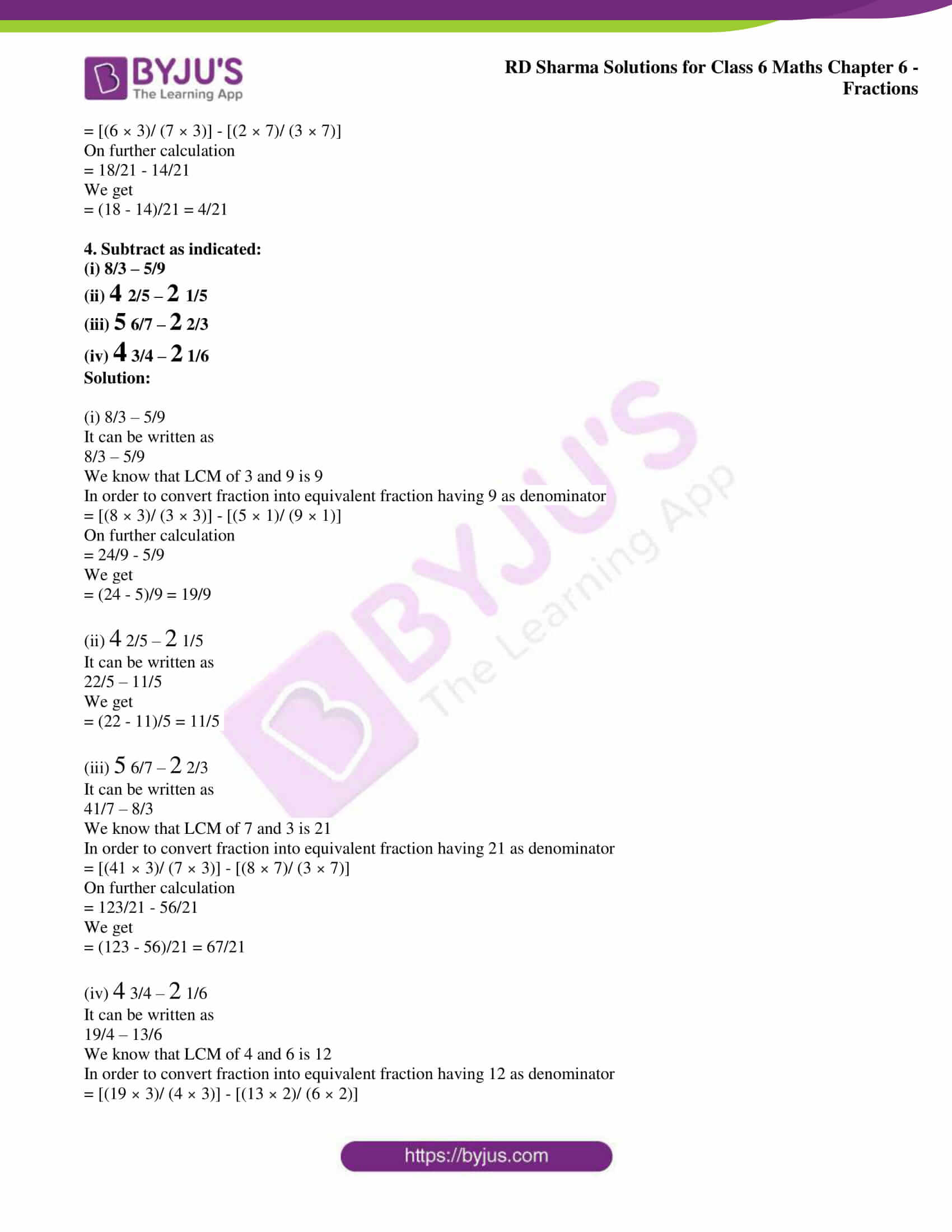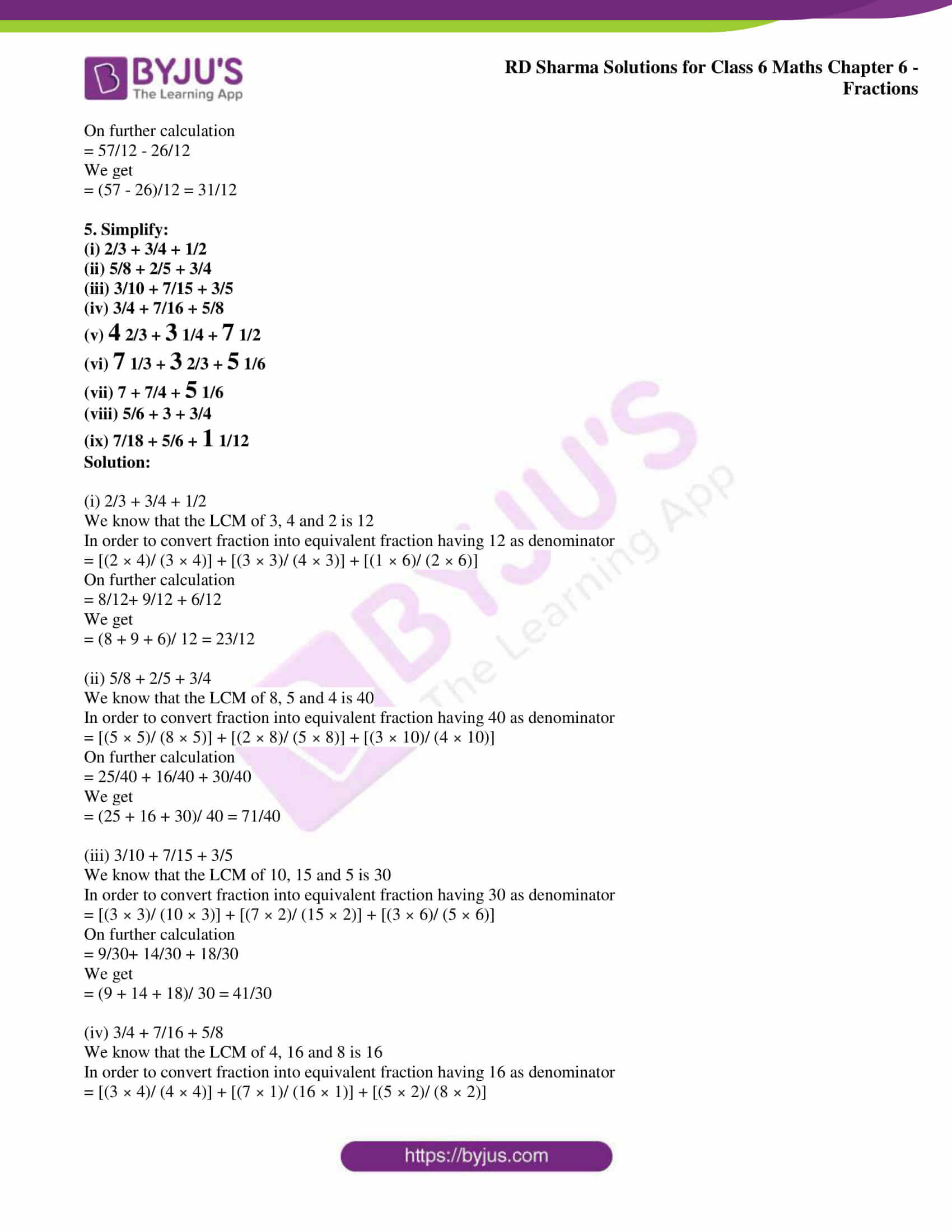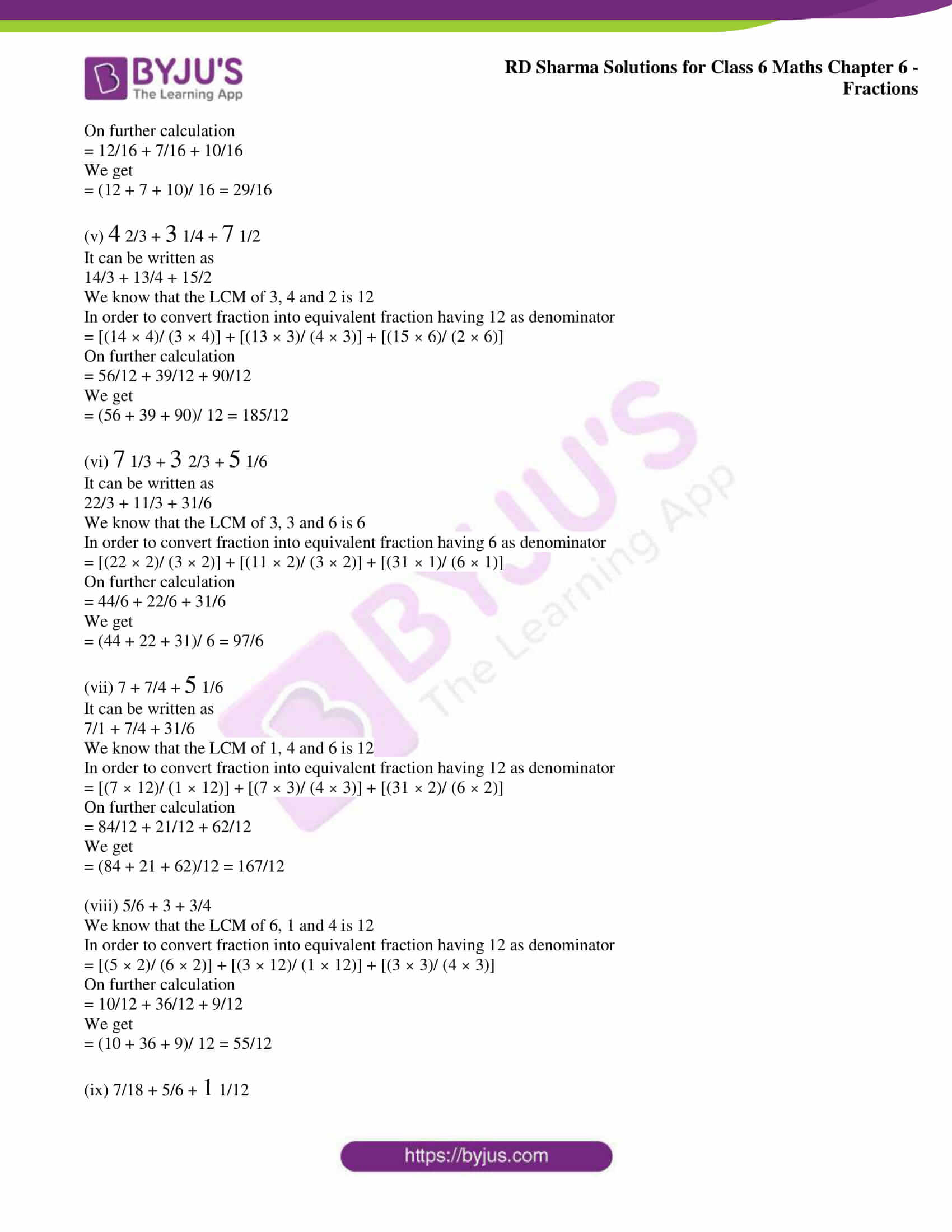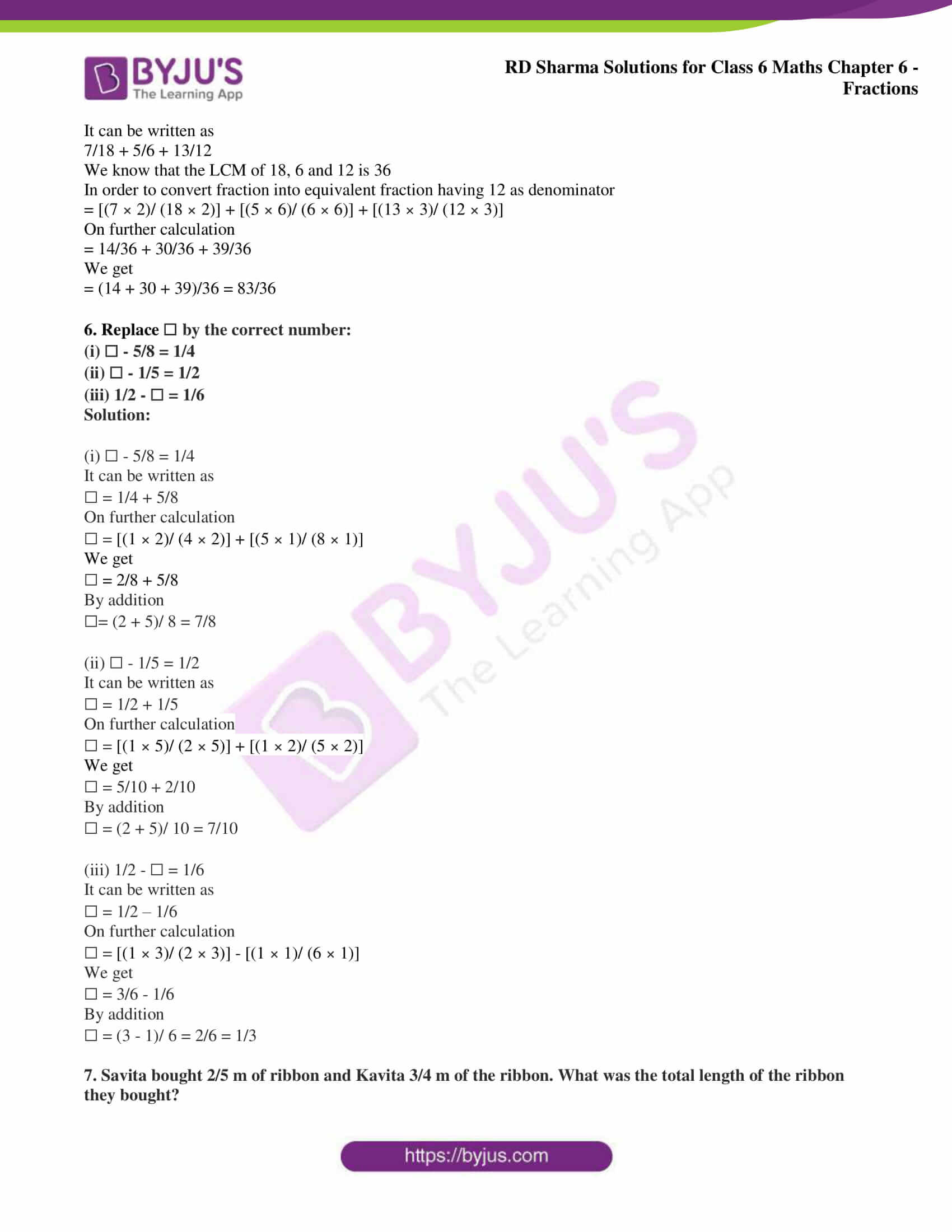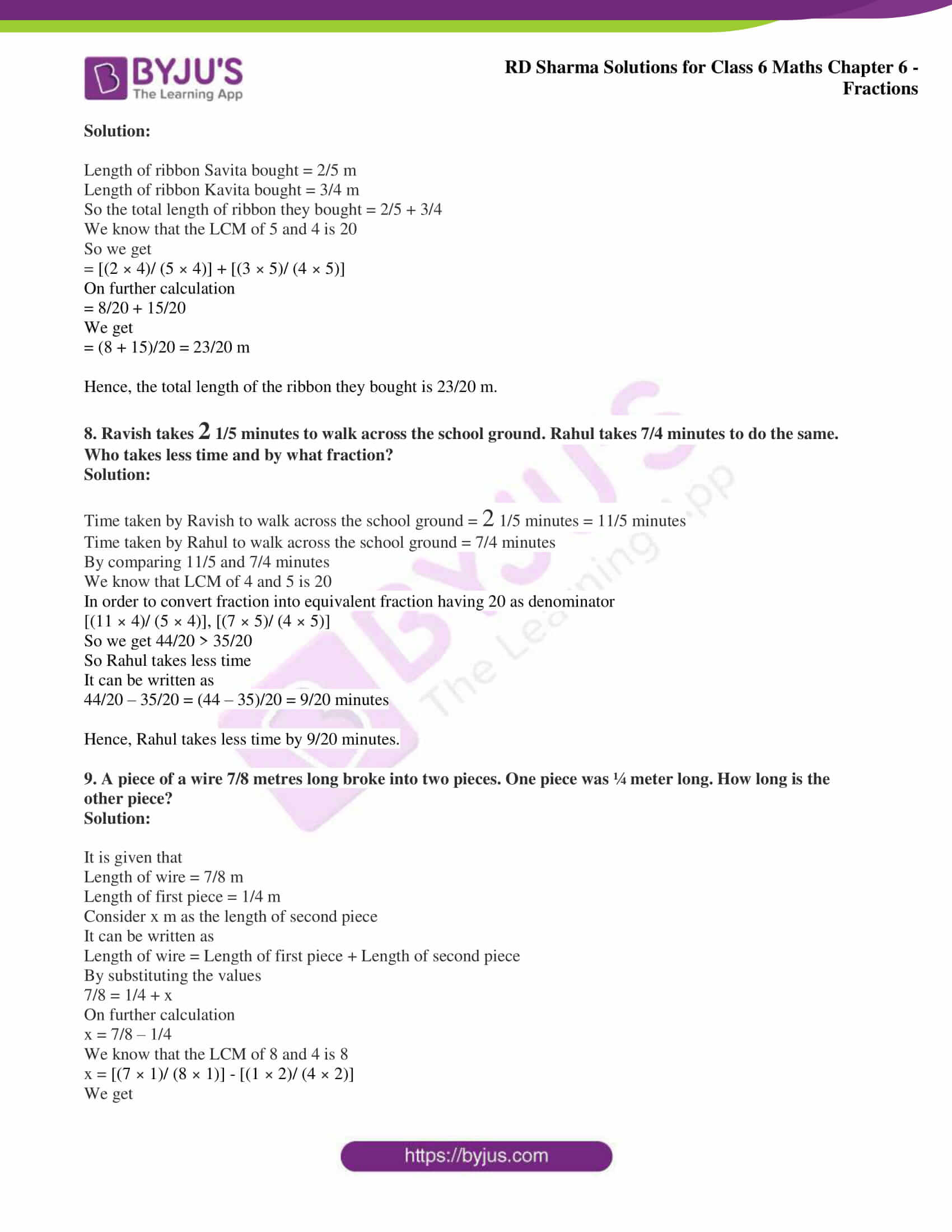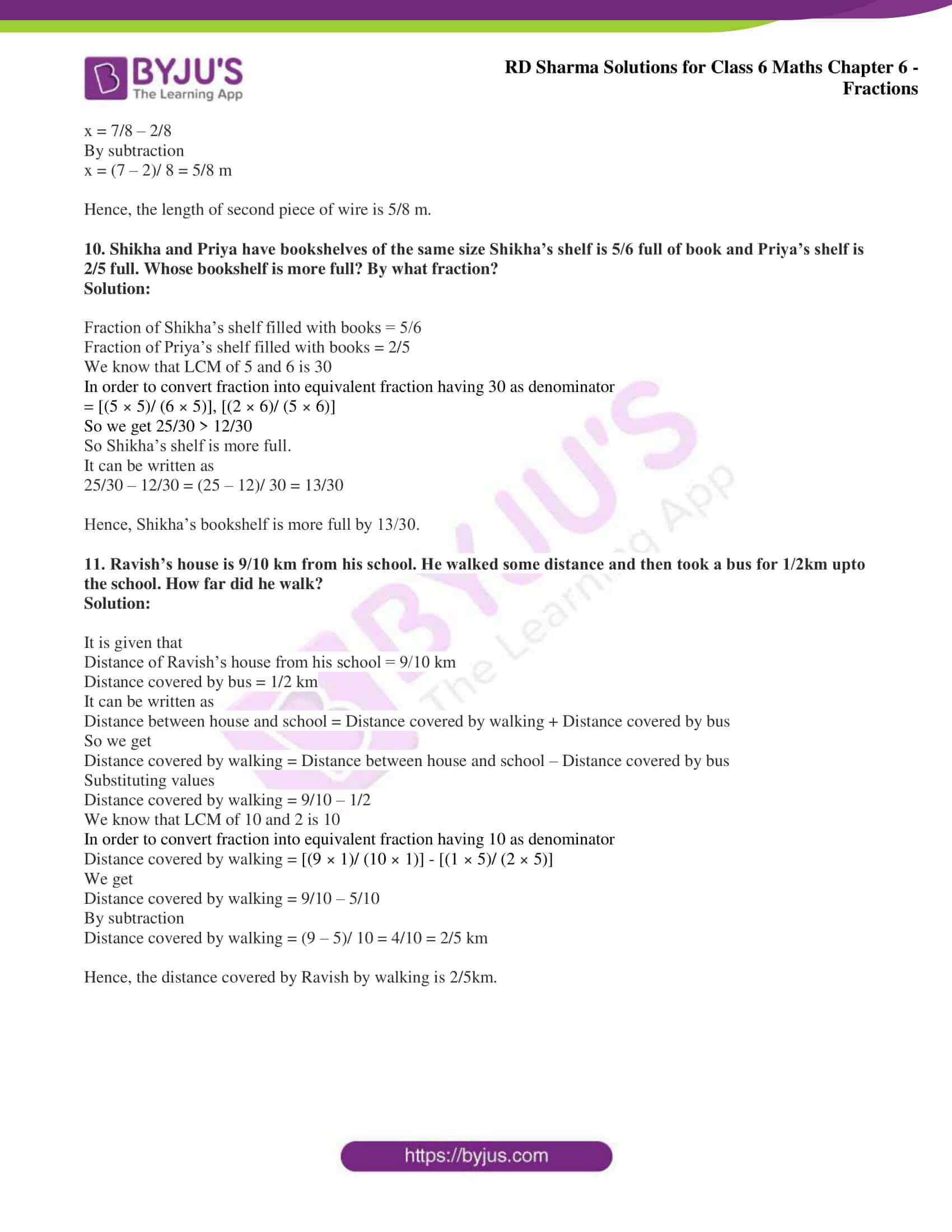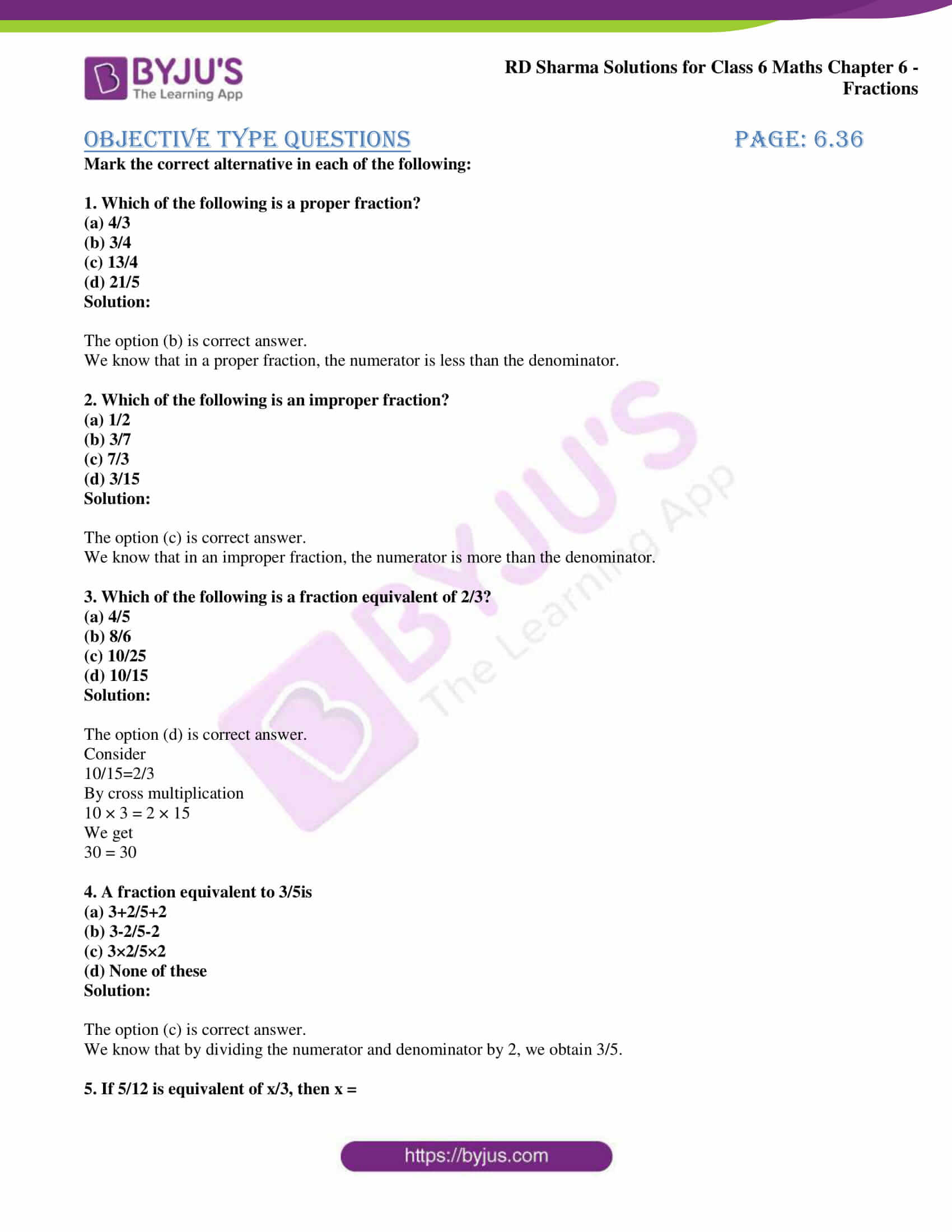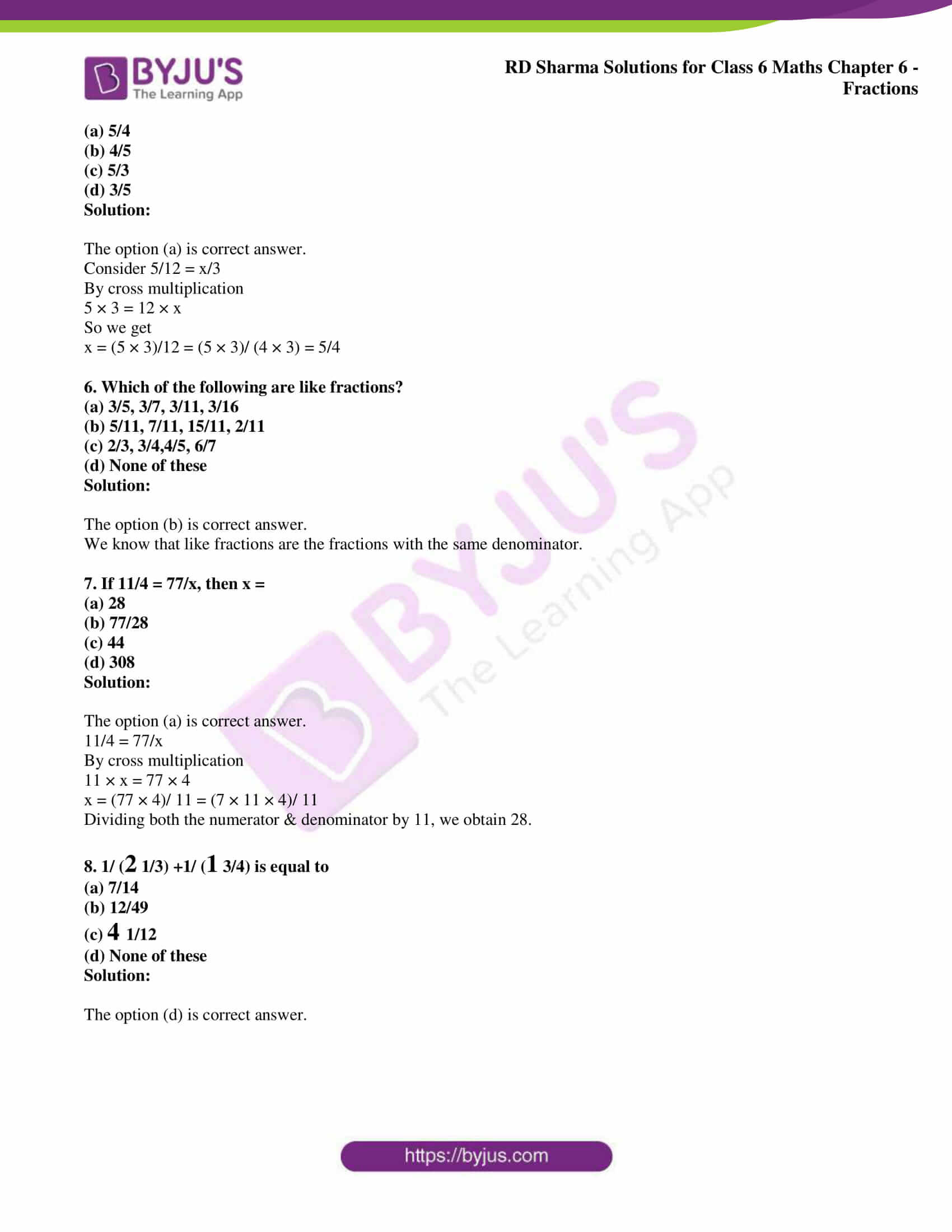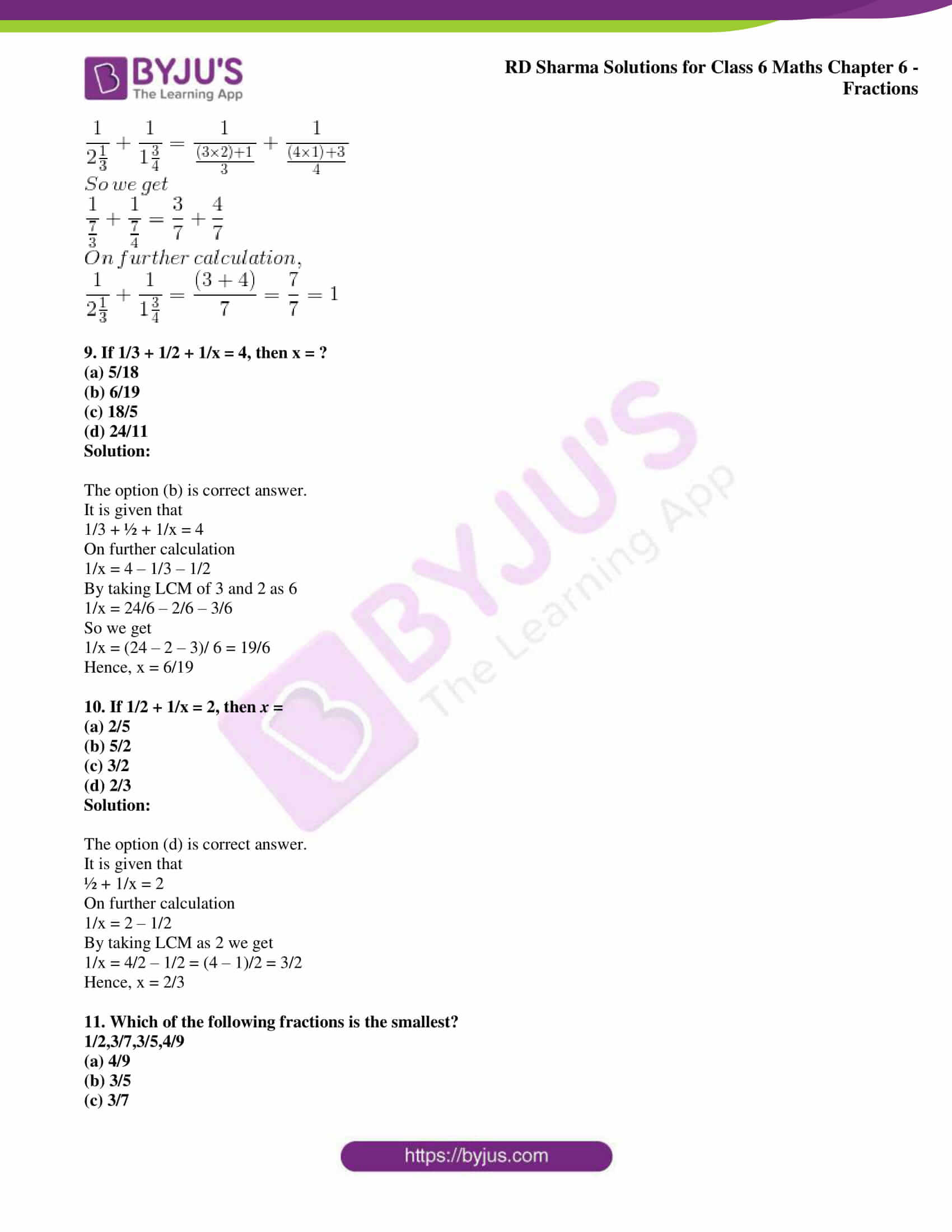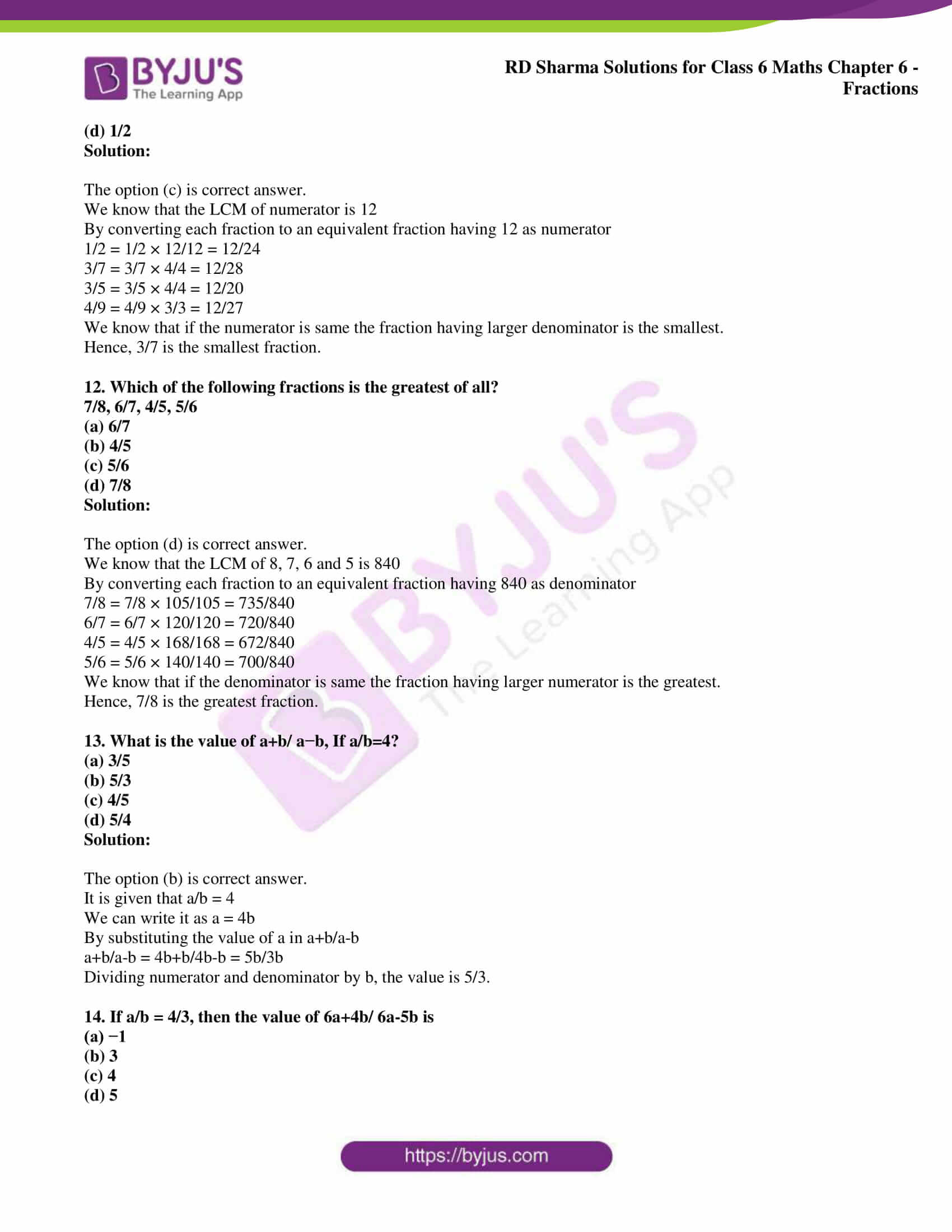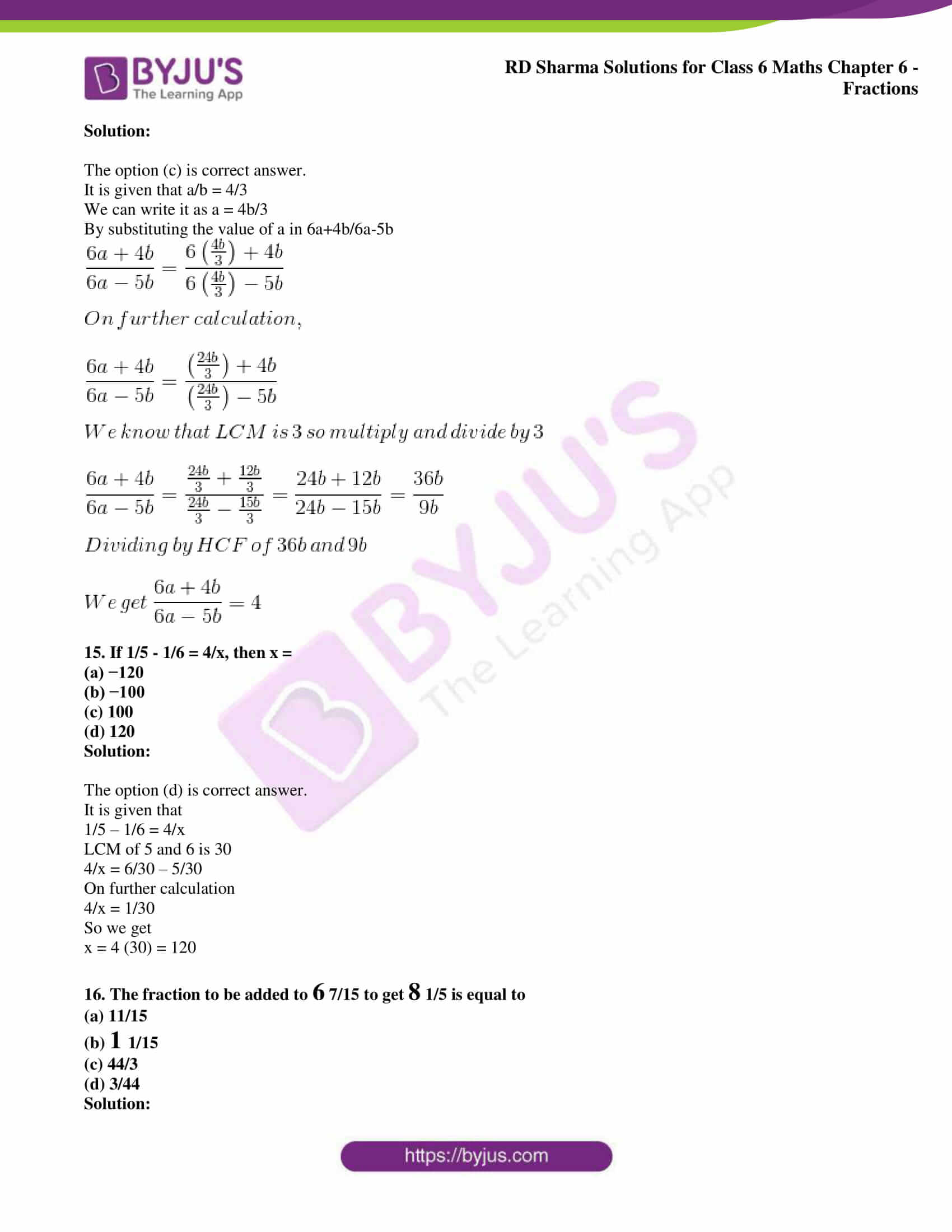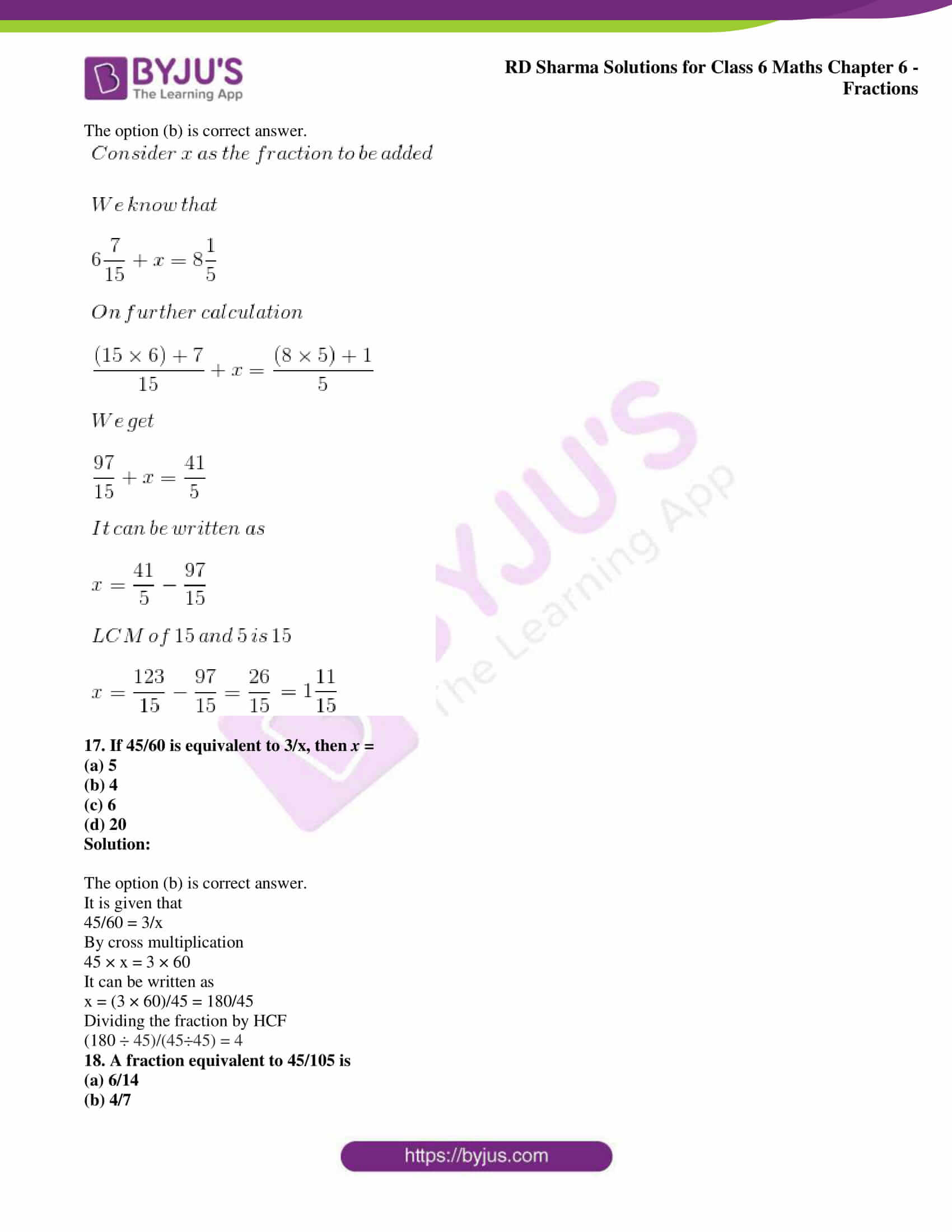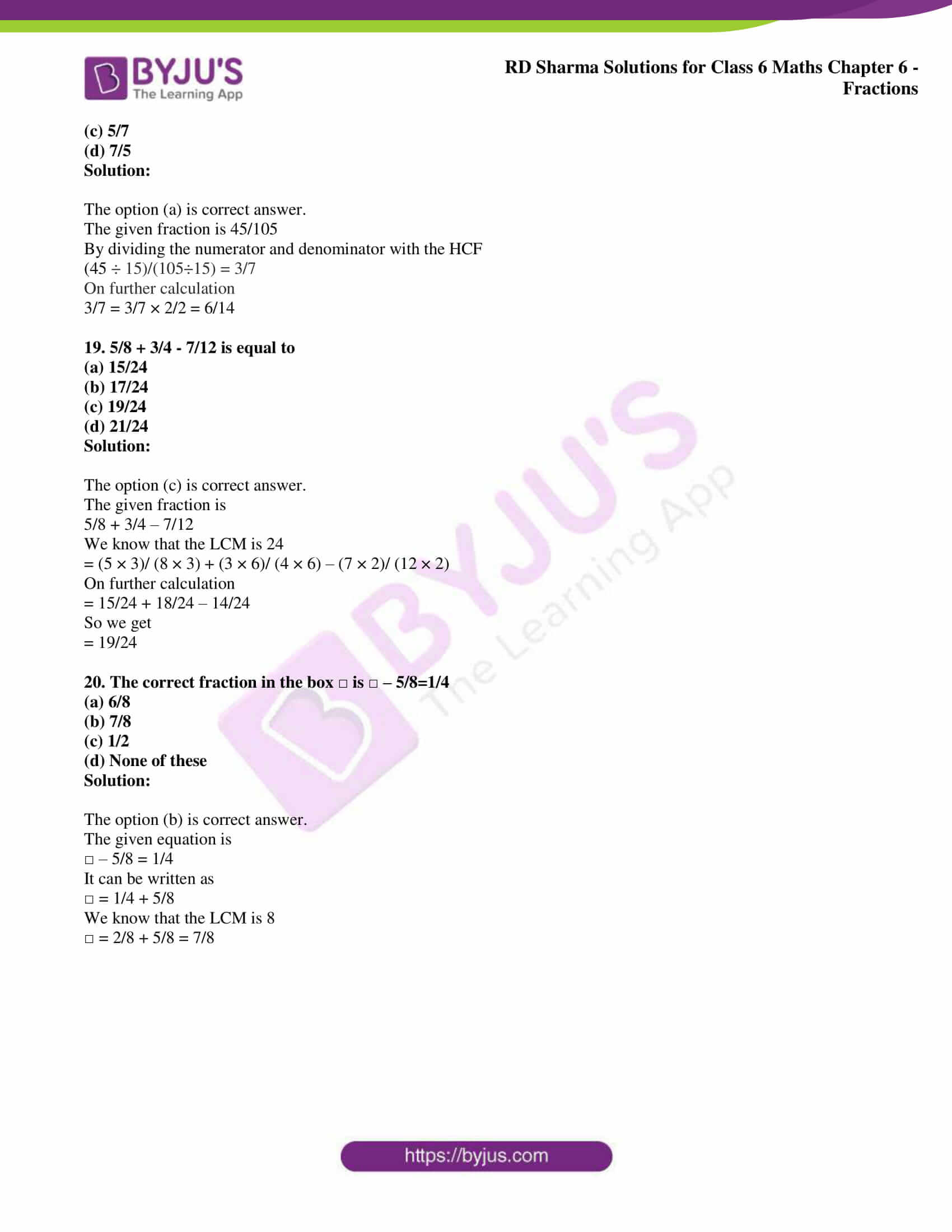## Access answers to Maths RD Sharma Solutions for Class 6 Chapter 6 Fractions

### Exercise 6.1 PAGE: 6.4

1. Write the fraction representing the shaded portion: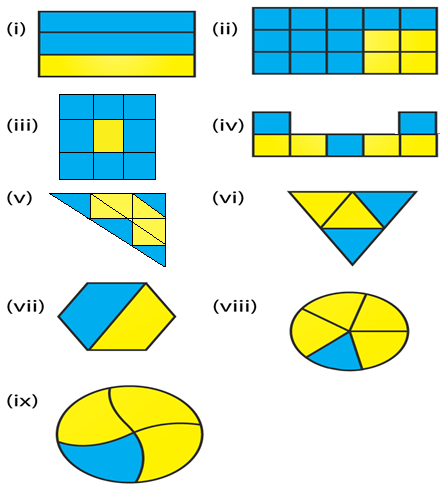Solution:

We know that

Fraction of the shaded portion = Number of shaded parts/ Total number of parts

(i) From the figure we know that

Total number of parts = 3

Number of parts which are shaded = 2

So we get

Fraction of the shaded portion = 2/3

(ii) From the figure we know that

Total number of parts = 15

Number of parts which are shaded = 11

So we get

Fraction of the shaded portion = 11/15

(iii) From the figure we know that

Total number of parts = 9

Number of parts which are shaded = 8

So we get

Fraction of the shaded portion = 8/9

(iv) From the figure we know that

Total number of parts = 7

Number of parts which are shaded = 3

So we get

Fraction of the shaded portion = 3/7

(v) From the figure we know that

Total number of parts = 9

Number of parts which are shaded = 4

So we get

Fraction of the shaded portion = 4/9

(vi) From the figure we know that

Total number of parts = 4

Number of parts which are shaded = 2

So we get

Fraction of the shaded portion = 2/4 = 1/2

(vii) From the figure we know that

Total number of parts = 2

Number of parts which are shaded = 1

So we get

Fraction of the shaded portion = 1/2

(viii) From the figure we know that

Total number of parts = 5

Number of parts which are shaded = 1

So we get

Fraction of the shaded portion = 1/5

(ix) From the figure we know that

Total number of parts = 4

Number of parts which are shaded = 1

So we get

Fraction of the shaded portion = 1/4

2. Write the fraction representing the shaded parts: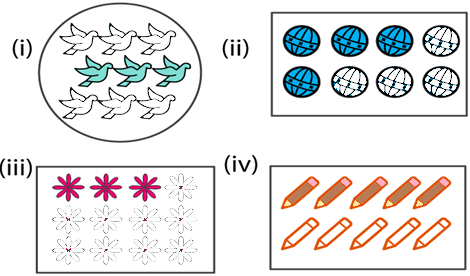Solution:

We know that

Fraction of the shaded portion = Number of shaded parts/ Total number of parts

(i) From the figure we know that

Total number of parts = 9

Number of parts which are shaded = 3

So we get

Fraction of the shaded portion = 3/9 = 1/3

(ii) From the figure we know that

Total number of parts = 8

Number of parts which are shaded = 4

So we get

Fraction of the shaded portion = 4/8 = 1/2

(iii) From the figure we know that

Total number of parts = 12

Number of parts which are shaded = 3

So we get

Fraction of the shaded portion = 3/12 = 1/4

(iv) From the figure we know that

Total number of parts = 10

Number of parts which are shaded = 5

So we get

Fraction of the shaded portion = 5/10 = 1/2

3. Write the fraction representing the shaded portion: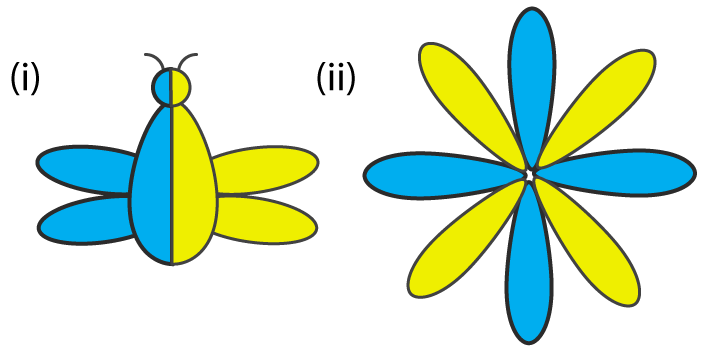Solution:

We know that

Fraction of the shaded portion = Number of shaded parts/ Total number of parts

(i) From the figure we know that

Total number of parts = 2

Number of parts which are shaded = 1

So we get

Fraction of the shaded portion = 1/2

(ii) From the figure we know that

Total number of parts = 8

Number of parts which are shaded = 4

So we get

Fraction of the shaded portion = 4/8

4. Colour the part according to the fraction given: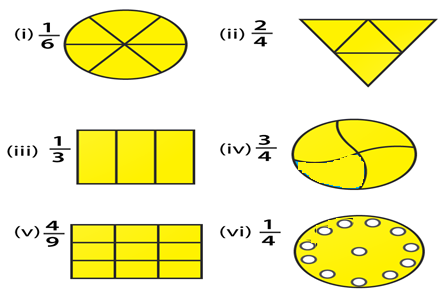Solution: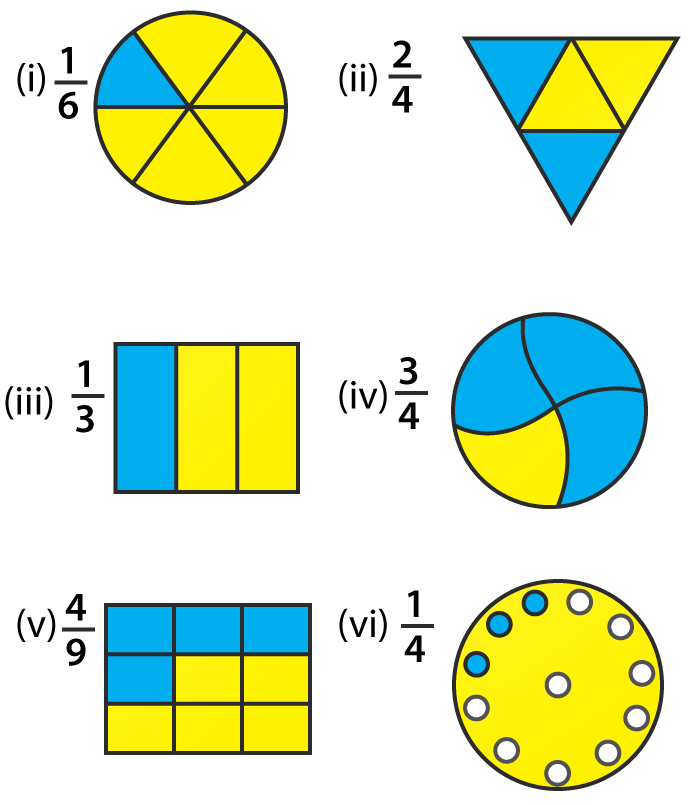5. What fraction of an hour is 20 minutes?

Solution:

We know that

Minutes in an hour = 60

So 20 minutes of an hour = 20/ 60 = 1/3

Therefore, 1/3 of an hour is 20 minutes.

6. Write the natural numbers from 2 to 12. What fraction of them are prime numbers?

Solution:

We know that natural numbers from 2 to 12 are

2, 3, 4, 5, 6, 7, 8, 9, 10, 11 and 12

The prime numbers from 2 to 12 are

2, 3, 5, 7 and 11

So 5 numbers are prime among the 11 numbers

Therefore, 5/11 of them are prime numbers.

7. Write the natural numbers from 102 to 113. What fraction of them are prime numbers?

Solution:

We know that the natural numbers from 102 to 113 are

102, 103, 104, 105, 106, 107, 108, 109, 110, 111, 112 and 113

The prime numbers from 102 to 113 are

103, 107, 109 and 113

So 4 numbers are prime among the 12 numbers

We get the fraction of prime numbers = 4/ 12 = 1/3

Therefore, 1/3 fraction of them are prime numbers.

8. Mukesh has a box of 24 pencils. He gives half of them to Sunita. How many does Sunita get? How many does Mukesh still have?

Solution:

Number of pencils Mukesh has = 24

He gives half of them to Sunita = 24/ 2 = 12

So the number of pencils Mukesh still has = 24 – 12 = 12

Therefore, Mukesh gives 12 pencils to Sunita and still has 12 pencils.

9. Kavita has 44 cassettes. She gives ¾ of them to Sonia. How many does Sonia get? How many does Kavita keep?

Solution:

Number of cassettes Kavita has = 44

She gives ¾ of them to Sonia = ¾ (44) = 33

So the number of cassettes Kavita keeps = 44 – 33 = 11

Therefore, Kavita gives 33 cassettes to Sonia and still keeps 11 cassettes.

10. Shikhas has three frocks that she wears when playing. The material is good, but the colours are faded. Her mother buys some blue dye and uses it on two of the frocks. What fraction of all of the Shikha play frocks did her mother dye?

Solution:

Number of frocks = 3

Number of frocks Shikha’s mother dyed = 2

So the fraction of dyed frocks = 2/3

Hence, Shikha’s mother dyed 2/3 fraction of all of Shika’s play frocks.

### Exercise 6.2 page: 6.7

1. Represent 2/5 on a number line.

Solution:

The fraction 2/5 is represented on a number line as given below: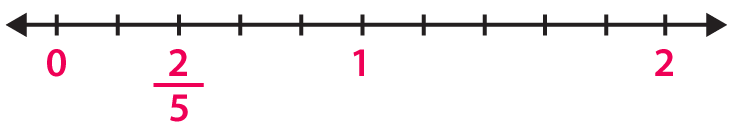2. Represent 0/10, 1/10, 5/10 and 10/10 on a number line.

Solution:

The fraction 0/10, 1/10, 5/10 and 10/10 are represented on a number line as given below: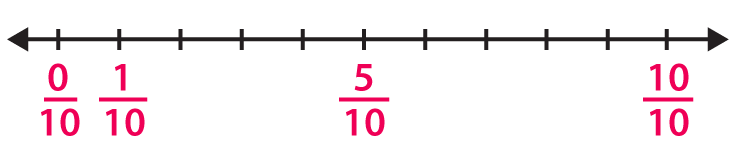3. Represent 2/7, 5/7 and 6/7 on a number line.

Solution:

The fraction 2/7, 5/7 and 6/7 are represented on a number line as given below: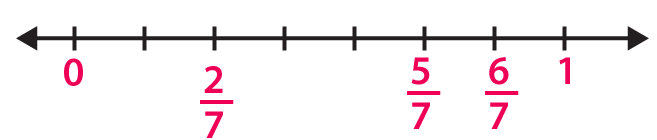4. How many fractions lie between 0 and 1.

Solution:

Infinite number of fractions lie between 0 and 1

This can be done by taking numerator less than denominator in a fraction.

5. Represent 0/8 and 8/8 on a number line.

Solution:

The fraction 0/8 and 8/8 are represented on a number line as given below: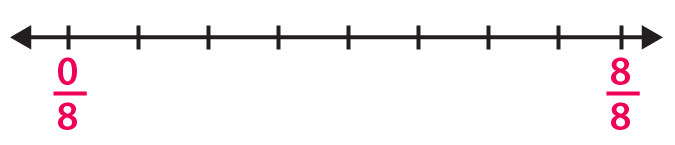### Exercise 6.3 page: 6.8

1. Write each of the following divisions as fractions:

(i) 6 ÷ 3

(ii) 25 ÷ 5

(iii) 125 ÷ 50

(iv) 55 ÷ 11

Solution:

(i) The division 6 ÷ 3 can be written as 6/3.

(ii) The division 25 ÷ 5 can be written as 25/5.

(iii) The division 125 ÷ 50 can be written as 125/50.

(iv) The division 55 ÷ 11 can be written as 55/11.

2. Write each of the following fractions as divisions:

(i) 9/7

(ii) 3/11

(iii) 90/63

(iv) 1/5

Solution:

(i) The fraction 9/7 can be written as 9 ÷ 7.

(ii) The fraction 3/11 can be written as 3 ÷ 11.

(iii) The fraction 90/63 can be written as 90 ÷ 63.

(iv) The fraction 1/5 can be written as 1 ÷ 5.

### Exercise 6.4 page: 6.11

1. Convert each of the following into a mixed fraction:

(i) 28/9

(ii) 226/15

(iii) 145/9

(iv) 128/5

Solution:

(i) 28/9 can be written as a mixed fraction as 3 1/9.

(ii) 226/15 can be written as a mixed fraction as 15 1/15.

(iii) 145/9 can be written as a mixed fraction as 16 1/9.

(iv) 128/5 can be written as a mixed fraction as 25 3/5.

2. Convert each of the following into an improper fraction:

(i) 7 ¼

(ii) 8 5/7

(iii) 5 3/10

(iv) 12 7/15

Solution:

(i) 7 ¼ can be written as an improper fraction as 29/4.

(ii) 8 5/7 can be written as an improper fraction as 61/7.

(iii) 5 3/10 can be written as an improper fraction as 53/10.

(iv) 12 7/15 can be written as an improper fraction as 187/15.

### Exercise 6.5 page: 6.15

1. Write the fractions and check whether they are equivalent or not: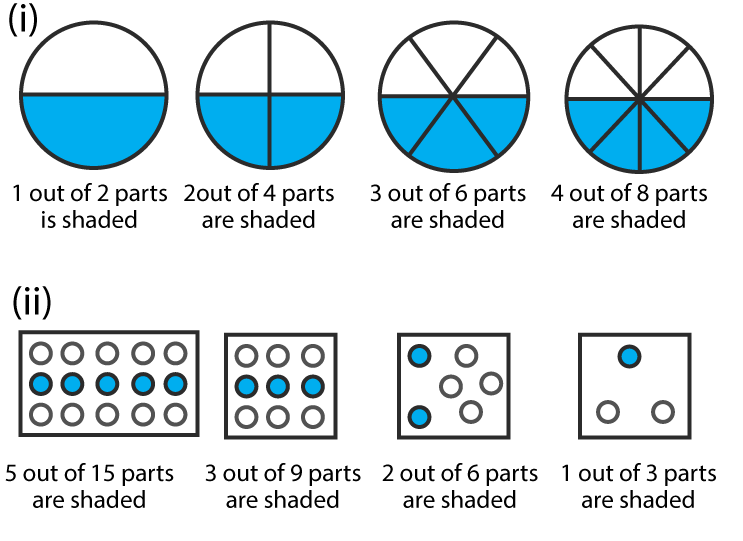Solution:

(i) We know that

Fraction = ½

Fraction = 2/4 = 1/2

Fraction = 3/6 = ½

Fraction = 4/8 = ½

Hence, they are equivalent.

(ii) We know that

Fraction = 5/15 = 1/3

Fraction = 3/9 = 1/3

Fraction = 2/6 = 1/3

Fraction = 1/3

Hence, they are equivalent.

2. Write the fractions and match fractions in Column I with the equivalent fractions in Column II.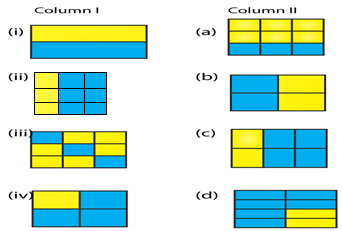Solution:

(i) (b)

(ii) (c)

(iii) (a)

(iv) (d)

3. Replace ☐ in each of the following by the correct number:

(i) 2/7 = 6/ ☐

(ii) 5/8 = 10/☐

(iii) 4/5 = ☐/20

(iv) 45/60 = 15/ ☐

(v) 18/24 = ☐/4

Solution:

(i) 2/7 = 6/21

(ii) 5/8 = 10/16

(iii) 4/5 = 16/20

(iv) 45/60 = 15/20

(v) 18/24 =3/4

4. Find the equivalent fraction of 3/5, having:

(i) numerator 9

(ii) denominator 30

(iii) numerator 21

(iv) denominator 40

Solution:

(i) The given fraction = 3/5

By considering numerator = 9

We know that 3 × 3 = 9

Multiply the numerator and denominator of the fraction by 3

3/5 × 3/3 = 9/15

(ii) The given fraction = 3/5

By considering denominator = 30

We know that 5 × 6 = 30

Multiply the numerator and denominator of the fraction by 6

3/5 × 6/6 = 18/30

(iii) The given fraction = 3/5

By considering numerator = 21

We know that 3 × 7 = 21

Multiply the numerator and denominator of the fraction by 7

3/5 × 7/7 = 21/35

(iv) The given fraction = 3/5

By considering denominator = 40

We know that 5 × 8 = 40

Multiply the numerator and denominator of the fraction by 8

3/5 × 8/8 = 24/40

5. Find the fraction equivalent to 45/60, having:

(i) numerator 15

(ii) denominator 4

(iii) denominator 240

(iv) numerator 135

Solution:

(i) The given fraction = 45/60

By considering numerator = 15

We know that 45 ÷ 3 = 15

Dividing the numerator and denominator of the fraction by 3

45/60 ÷ 3/3 = 15/20

(ii) The given fraction = 45/60

By considering denominator = 4

We know that 60 ÷ 15 = 4

Dividing the numerator and denominator of the fraction by 15

45/60 ÷ 15/15 = 3/4

(iii) The given fraction = 45/60

By considering denominator = 240

We know that 60 × 4 = 240

Multiply the numerator and denominator of the fraction by 4

45/60 × 4/4 = 180/240

(iv) The given fraction = 45/60

By considering numerator = 135

We know that 45 × 3 = 135

Multiply the numerator and denominator of the fraction by 3

45/60 × 3/3 = 135/180

6. Find the fraction equivalent of 35/42, having:

(i) numerator 15

(ii) denominator 18

(iii) denominator 30

(iv) numerator 30

Solution:

The given fraction = 35/42

In order to reduce the fraction, divide the numerator and denominator by the HCF of 35 and 42

We get

35/42 ÷ 7/7 = 5/6

(i) So the fraction = 5/6

By considering numerator = 15

We know that 5 × 3 = 15

Multiply the numerator and denominator of the fraction by 3

5/6 × 3/3 = 15/18

(ii) So the fraction = 5/6

By considering denominator = 18

We know that 6 × 3 = 18

Multiply the numerator and denominator of the fraction by 3

5/6 × 3/3 = 15/18

(iii) So the fraction = 5/6

By considering denominator = 30

We know that 6 × 5 = 30

Multiply the numerator and denominator of the fraction by 5

5/6 × 5/5 = 25/30

(iv) So the fraction = 5/6

By considering numerator = 30

We know that 5 × 6 = 30

Multiply the numerator and denominator of the fraction by 6

5/6 × 6/6 = 30/36

7. Check whether the given fractions are equivalent:

(i) 5/9, 30/54

(ii) 2/7, 16/42

(iii) 7/13, 5/11

(iv) 4/11, 32/88

(v) 3/10, 12/50

(vi) 9/27, 25/75

Solution:

(i) We know that

5/9 × 6/6 = 30/54

Therefore, 5/9 is equivalent to 30/54.

(ii) We know that

2/7 × 8/8 = 16/56

Therefore, 2/7 is not equivalent to 16/42.

(iii) We know that

7/13 × 5/5 = 35/65

The same way

5/11 × 7/7 = 35/77

Therefore, 7/13 is not equivalent to 5/11.

(iv) We know that

4/11 × 8/8 = 32/88

Therefore, 4/11 is equivalent to 32/88.

(v) We know that

3/10 × 4/4 = 12/40

Therefore, 3/10 is not equivalent to 12/50.

(vi) We know that

9/27 = 1/3 and 25/75 = 1/3

Therefore, 9/27 is equivalent to 25/75.

8. Match the equivalent fractions and write another 2 for each:

(i) 250/400 (a) 2/3

(ii) 180/200 (b) 2/5

(iii) 660/990 (c) ½

(iv) 180/360 (d) 5/8

(v) 220/550 (e) 9/10

Solution:

(i) 250/400

By dividing numerator and denominator by HCF of 250 and 400

= (250/50)/ (400/ 50) = 5/8

So the match is (d)

(ii) 180/200

By dividing numerator and denominator by HCF of 180 and 200

= (180/20)/ (200/20) = 9/10

So the match is (e)

(iii) 660/990

By dividing numerator and denominator by HCF of 660 and 990

= (660/90)/ (990/90) = 2/3

So the match is (a)

(iv) 180/360

By dividing numerator and denominator by HCF of 180 and 360

= (180/180)/ (360/180) = ½

So the match is (c)

(v) 220/550

By dividing numerator and denominator by HCF of 220 and 550

= (220/11)/ (550/11) = 2/5

So the match is (b)

9. Write some equivalent fractions which contain all digits from 1 to 9 once only.

Solution:

The equivalent fractions which contain all digits from 1 to 9 once only are

2/6 = 3/9 = 58/174, 2/4 = 3/6 = 79/158

10. Ravish had 20 pencils, Shikha had 50 pencils and Priya had 80 pencils. After 4 months, Ravish used up 10 pencils, Shikha used up 25 pencils and Priya used up 40 pencils. What fraction did each use up? Check if each has used up an equal fraction of their pencils?

Solution:

Number of pencils Ravish had = 20

Number of pencils Ravish used = 10

By dividing the numerator and denominator by HCF of 10 and 20

We get the fraction of pencils used = (10 ÷ 10)/ (20 ÷ 10) = 1/2

Number of pencils Shikha had = 50

Number of pencils used by Shikha = 25

By dividing the numerator and denominator by HCF of 25 and 50

We get the fraction of pencils used = (25 ÷ 25)/ (50 ÷ 25) = 1/2

Number of pencils Priya had = 80

Number of pencils used by Priya = 40

By dividing the numerator and denominator by HCF of 40 and 80

We get the fraction of pencils used = (40 ÷ 40)/ (80 ÷ 40) = 1/2

Yes, each has used up an equal fraction of their pencils.

### Exercise 6.6 PAGE: 6.19

Reduce each of the following fractions to its lowest term (simplest form):

1. (i) 40/75

(ii) 42/28

(iii) 12/52

(iv) 40/72

(v) 80/24

(vi) 84/56

Solution:

(i) 40/75

We know that

Factors of 40 are

1, 2, 4, 5, 8, 10, 20 and 40

Factors of 75 are

1, 3, 5, 15 and 75

So the common factors are 1 and 5

We get HCF = 5

By dividing numerator and denominator by 5

40/75 ÷ 5/5 = 8/15

Hence, the simplest form of 40/75 is 8/15.

(ii) 42/28

We know that

Factors of 42 are

1, 2, 3, 6, 7, 14, 21 and 42

Factors of 28 are

1, 2, 4, 7, 14 and 28

So the common factors are 1, 2 and 14

We get HCF = 14

By dividing numerator and denominator by 14

42/28 ÷ 14/14 = 3/2

Hence, the simplest form of 42/28 is 3/2.

(iii) 12/52

We know that

Factors of 12 are

1, 2, 3, 4, 6 and 12

Factors of 52 are

1, 2, 4, 13, 26 and 52

So the common factors are 1, 2 and 4

We get HCF = 4

By dividing numerator and denominator by 4

12/52 ÷ 4/4 = 3/13

Hence, the simplest form of 12/52 is 3/13.

(iv) 40/72

We know that

Factors of 40 are

1, 2, 4, 5, 8, 10, 20 and 40

Factors of 72 are

1, 2, 3, 4, 6, 8, 9, 12, 18, 24, 36 and 72

So the common factors are 1, 2, 4 and 8

We get HCF = 8

By dividing numerator and denominator by 8

40/72 ÷ 8/8 = 5/9

Hence, the simplest form of 40/72 is 5/9.

(v) 80/24

We know that

Factors of 80 are

1, 2, 4, 5, 8, 10, 16, 20, 40 and 80

Factors of 24 are

1, 2, 3, 4, 6, 8, 12 and 24

So the common factors are 1, 2, 4 and 8

We get HCF = 8

By dividing numerator and denominator by 8

80/24 ÷ 8/8 = 10/3

Hence, the simplest form of 80/24 is 10/3.

(vi) 84/56

We know that

Factors of 84 are

1, 2, 3, 4, 6, 7, 12, 14, 21, 28, 42 and 84

Factors of 56 are

1, 2, 4, 7, 8, 14, 28 and 56

So the common factors are 1, 2, 4, 7, 14 and 28

We get HCF = 28

By dividing numerator and denominator by 28

84/56 ÷ 28/28 = 3/2

Hence, the simplest form of 84/56 is 3/2.

2. Simplify each of the following to its lowest term:

(i) 75/80

(ii) 52/76

(iii) 84/98

(iv) 68/17

(v) 150/50

(vi) 162/108

Solution:

(i) 75/80

We know that

Factors of 75 are

1, 3, 5, 15, 25 and 75

Factors of 80 are

1, 2, 4, 5, 8, 10, 12, 16, 20, 40 and 80

So the common factors are 1 and 5

We get HCF = 5

By dividing numerator and denominator by 5

75/80 ÷ 5/5 = 15/16

Hence, the simplest form of 75/80 is 15/16.

(ii) 52/76

We know that

Factors of 52 are

1, 2, 4, 13, 26 and 52

Factors of 76 are

1, 2, 4, 19, 38 and 76

So the common factors are 1, 2 and 4

We get HCF = 4

By dividing numerator and denominator by 4

52/76 ÷ 4/4 = 13/19

Hence, the simplest form of 52/76 is 13/19.

(iii) 84/98

We know that

Factors of 84 are

1, 2, 3, 4, 6, 7, 12, 14, 21, 28, 42 and 84

Factors of 98 are

1, 2, 7, 14, 49 and 98

So the common factors are 1, 2, 7 and 14

We get HCF = 14

By dividing numerator and denominator by 14

84/98 ÷ 14/14 = 6/7

Hence, the simplest form of 84/98 is 6/7.

(iv) 68/17

We know that

Factors of 68 are

1, 2, 4, 17, 34 and 68

Factors of 17 are

1 and 17

So the common factors are 1 and 17

We get HCF = 17

By dividing numerator and denominator by 17

68/17 ÷ 17/17 = 4/1

Hence, the simplest form of 68/17 is 4/1.

(v) 150/50

We know that

Factors of 150 are

1, 2, 3, 5, 6, 10, 15, 25, 50 and 150

Factors of 50 are

1, 2, 5, 10, 25 and 50

So the common factors are 1, 2, 5, 10, 25 and 50

We get HCF = 50

By dividing numerator and denominator by 50

150/50 ÷ 50/50 = 3/1

Hence, the simplest form of 150/50 is 3/1.

(vi) 162/108

We know that

Factors of 162 are

1, 2, 3, 6, 9, 18, 27, 54, 81 and 162

Factors of 108 are

1, 2, 3, 4, 6, 9, 12, 18, 27 and 54

So the common factors are 1, 2, 3, 6, 9, 18, 27 and 54

We get HCF = 54

By dividing numerator and denominator by 54

162/108 ÷ 54/54 = 3/2

Hence, the simplest form of 162/108 is 3/2.

### Exercise 6.7 page: 6.24

1. Write each fraction. Arrange them in ascending and descending order using correct sign <, =, > between the fractions: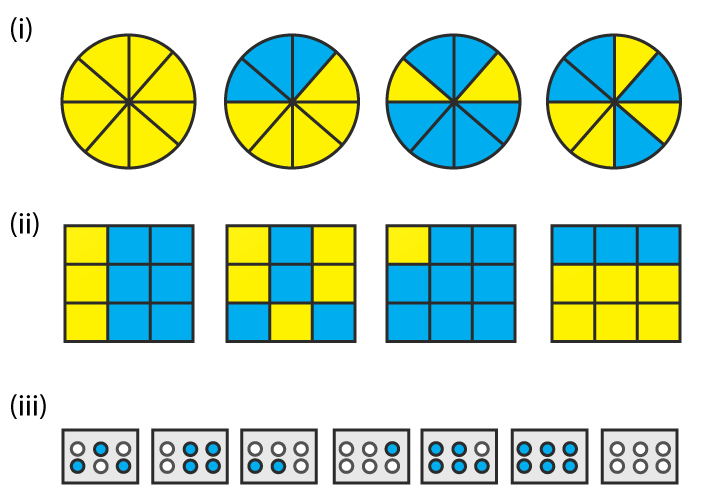Solution: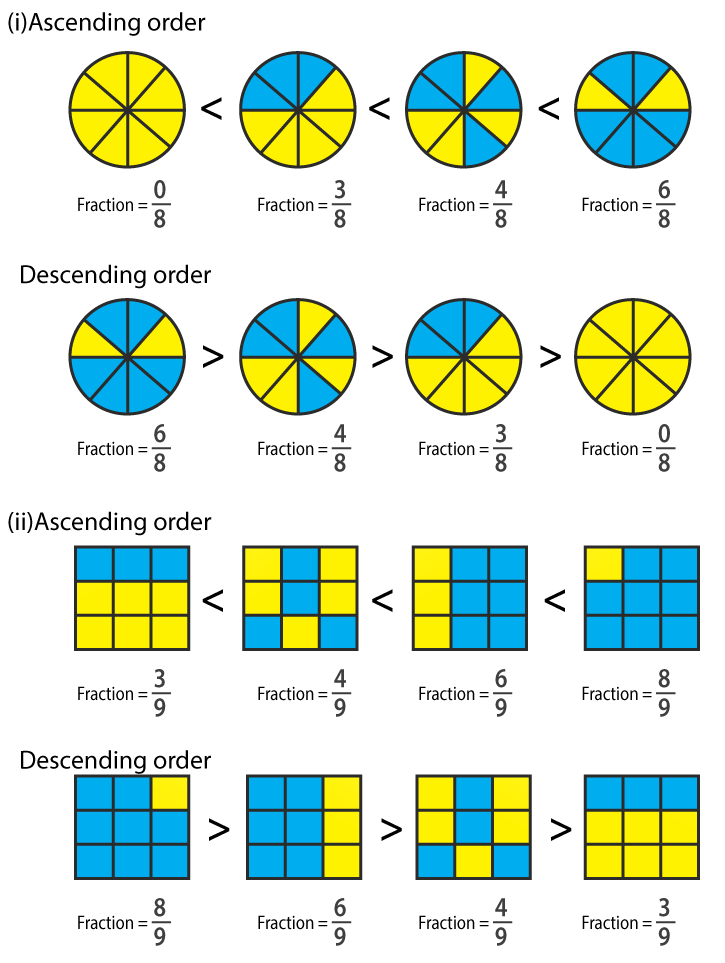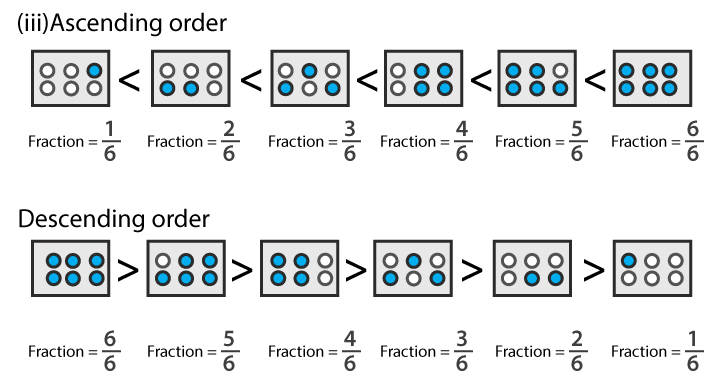2. Mark 2/6, 4/6, 8/6 and 6/6 on the number line and put appropriate signs between fractions given below:

(i) 5/6 …….. 2/6

(ii) 3/6 ……. 0/6

(iii) 1/6 …… 6/6

(iv) 8/6 …… 5/6

Solution:(i) We know that

5/6 > 2/6 as 5 > 2 and the denominator is same.

(ii) We know that

3/6 > 0/6 as 3 > 0 and the denominator is same.

(iii) We know that

1/6 < 6/6 as 6 > 1 and the denominator is same.

(iv) We know that

8/6 > 5/6 as 8 > 5 and the denominator is same.

3. Compare the following fractions and put an appropriate sign:

(i) 3/6 …… 5/6

(ii) 4/5 …… 0/5

(iii) 3/20 …… 4/20

(iv) 1/7 ……. 1/4

Solution:

(i) We know that

3/6 < 5/6 as 3 < 5 and the denominator is same.

(ii) We know that

4/5 > 0/5 as 4 > 0 and the denominator is same.

(iii) We know that

3/20 < 4/20 as 3 < 4 and the denominator is same.

(iv) We know that

1/7 < 1/4 as 7 > 4 and the fraction having smaller denominator is larger.

4. Compare the following fractions using the symbol > or <:

(i) 6/7 and 6/11

(ii) 3/7 and 5/7

(iii) 2/3 and 8/12

(iv) 1/5 and 4/15

(v) 8/3 and 8/13

(vi) 4/9 and 15/8

Solution:

(i) We know that

6/7 > 6/11 as the fraction having smaller denominator is larger.

(ii) We know that

3/7 < 5/7 as 3 < 5 and the denominator is same.

(iii) We know that

8/12 = (2 × 2 × 2)/ (2 × 2 × 3) = 2/3

Hence, 2/3 = 8/12

(iv) We know that

1/5 = (1/ 5) × (3/3) = 3/15 which is lesser than 4/15

Hence, 1/5 < 4/15

(v) We know that

8/3 > 8/13 as the fraction having smaller value of denominator is larger.

(vi) We know that

4/9 = (4/9) × (8/8) = 32/72

15/8 = (15/8) × (9/9) = 135/72

So we get 32/72 < 135/72

Hence, 4/9 < 15/8.

5. The following fractions represent just three different numbers. Separate them in to three groups of equal fractions by changing each one to its simplest form:

(i) 2/12

(ii) 3/15

(iii) 8/50

(iv) 16/100

(v) 10/60

(vi) 15/75

(vii) 12/60

(viii) 16/96

(ix) 12/75

(x) 12/72

(xi) 3/18

(xii) 4/25

Solution:

(i) 2/12

We know that HCF of 2 and 12 = 2

By dividing numerator and denominator by HCF of 2 and 12

2/12 ÷ 2/2 = 1/6

(ii) 3/15

We know that HCF of 3 and 15 = 3

By dividing numerator and denominator by HCF of 3 and 15

3/15 ÷ 3/3 = 1/5

(iii) 8/50

We know that HCF of 8 and 50 = 2

By dividing numerator and denominator by HCF of 8 and 50

8/50 ÷ 2/2 = 4/25

(iv) 16/100

We know that HCF of 16 and 100 = 4

By dividing numerator and denominator by HCF of 16 and 100

16/100 ÷ 4/4 = 4/25

(v) 10/60

We know that HCF of 10 and 60 = 10

By dividing numerator and denominator by HCF of 10 and 60

10/60 ÷ 10/10 = 1/6

(vi) 15/75

We know that HCF of 15 and 75 = 15

By dividing numerator and denominator by HCF of 15 and 75

15/75 ÷ 15/15 = 1/5

(vii) 12/60

We know that HCF of 2 and 12 = 12

By dividing numerator and denominator by HCF of 2 and 12

12/60 ÷ 12/12 = 1/5

(viii) 16/96

We know that HCF of 16 and 96 = 16

By dividing numerator and denominator by HCF of 16 and 96

16/96 ÷ 16/16 = 1/6

(ix) 12/75

We know that HCF of 12 and 75 = 3

By dividing numerator and denominator by HCF of 12 and 75

12/75 ÷ 3/3 = 4/25

(x) 12/72

We know that HCF of 12 and 72 = 12

By dividing numerator and denominator by HCF of 12 and 72

12/72 ÷ 12/12 = 1/6

(xi) 3/18

We know that HCF of 3 and 18 = 3

By dividing numerator and denominator by HCF of 3 and 18

3/18 ÷ 3/3 = 1/6

(xii) 4/25

We know that HCF of 4 and 25 = 1

By dividing numerator and denominator by HCF of 4 and 25

4/25 ÷ 1/1 = 4/25

Three groups of equal fractions: 2/12, 10/60, 16/96, 12/72, 3/18, 3/15, 15/75, 12/60, 8/50, 16/100, 12/75, 4/25

6. Isha read 25 pages of a book containing 100 pages. Nagma read ½ of the same book. Who read less?

Solution:

No. of pages in the book = 100

We know that

Fraction of book Isha read = (25/100) ÷ (25/25) = 1/4 by dividing both numerator and denominator by HCF of 25 and 100

So the fraction of book Nagma read = 1/2

By comparing 1/4 and 1/2 we get the LCM of 4 and 2 = 4

Now convert the fraction into equivalent fraction having denominator as 4

1/4 × 1/1 and 1/2 × 2/2

1/4 < ½

7. Arrange the following fractions in the ascending order:

(i) 2/9, 7/9, 3/9, 4/9, 1/9, 6/9, 5/9

(ii) 7/8, 7/25, 7/11, 7/18, 7/10

(iii) 37/47, 37/50, 37/100, 37/1000, 37/85, 37/41

(iv) 3/5, 1/5, 4/5, 2/5

(v) 2/5, 3/4, 1/2, 3/5

(vi) 3/8, 3/12. 3/6, 3/4

(vii) 4/6, 3/8, 6/12, 5/16

Solution:

(i) 2/9, 7/9, 3/9, 4/9, 1/9, 6/9, 5/9 can be written in ascending order as

1/9, 2/9, 3/9, 4/9, 5/9, 6/9, 7/9

(ii) 7/8, 7/25, 7/11, 7/18, 7/10 can be written in ascending order as

7/25, 7/18, 7/11, 7/10, 7/8

(iii) 37/47, 37/50, 37/100, 37/1000, 37/85, 37/41 can be written in ascending order as

37/1000, 37/100, 37/85, 37/50, 37/47, 37/41

(iv) 3/5, 1/5, 4/5, 2/5 can be written in ascending order as

1/5, 2/5, 3/5, 4/5

(v) 2/5, 3/4, 1/2, 3/5 can be written in ascending order as

2/5, 1/2, 3/5, 3/4

(vi) 3/8, 3/12, 3/6, 3/4 can be written in ascending order as

3/12, 3/8, 3/6, 3/4

(vii) 4/6, 3/8, 6/12, 5/16 can be written in ascending order as

5/16, 3/8, 6/12, 4/6

8. Arrange in descending order in each of the following using the symbol >:

(i) 8/17, 8/9, 8/5, 8/13

(ii) 5/9, 3/12, 1/3, 4/15

(iii) 2/7, 11/35, 9/14, 13/28

Solutions:

(i) 8/17, 8/9, 8/5, 8/13 can be written in the descending order as

8/5 > 8/9 > 8/13 > 8/17

(ii) 5/9, 3/12, 1/3, 4/15 can be written in the descending order as

5/9 > 1/3 > 4/15> 3/12

(iii) 2/7, 11/35, 9/14, 13/28 can be written in the descending order as

9/14 > 13/28 > 11/35 > 2/7

9. Find answers to the following. Write and indicate how you solved them.

(i) Is 5/9 equal to 4/5?

(ii) Is 9/16 equal to 5/9?

(iii) Is 4/5 equal to 16/20?

(iv) Is 1/15 equal to 4/30?

Solution:

(i) No. We know that 5 × 5 ≠ 9 × 4

(ii) No. We know that 9 × 9 ≠ 16 × 5

(iii) Yes. We know that 4 × 20 = 16 × 5

(iv) No. We know that 1 × 30 ≠ 15 × 4

### Exercise 6.8 PAGE: 6.29

1. Write these fractions appropriately as additions or subtractions: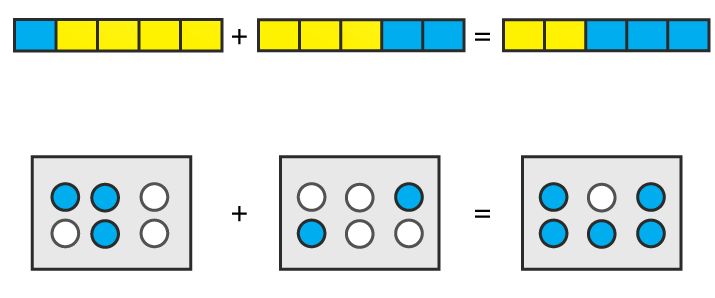Solution:

(i) It can be written as

1/5 + 2/5 = 3/5

(ii) It can be written as

3/6 + 2/6 = 5/6

2. Solve:

(i) 5/12 + 1/12

(ii) 3/15 + 7/15

(iii) 3/22 + 7/22

(iv) 1/4 + 0/4

(v) 4/13 + 2/13 + 1/13

(vi) 0/15 + 2/15 + 1/15

(vii) 7/31 – 4/31 + 9/31

(viii) 3 2/7 + 1/7 – 2 3/7

(ix) 2 1/3 – 1 2/3 + 4 1/3

(x) 1 – 2/3 + 7/3

(xi) 16/7 – 5/7 + 9/7

Solution:

(i) 5/12 + 1/12

It can be written as

5/ 12 + 1/12 = (5 + 1)/ 12

On further calculation

5/ 12 + 1/12 = 6/12 = 1/2

(ii) 3/15 + 7/15

It can be written as

3/15 + 7/15 = (3 + 7)/ 15

On further calculation

3/15 + 7/15 = 10/15 = 2/3

(iii) 3/22 + 7/22

It can be written as

3/22 + 7/22 = (3 + 7)/ 22

On further calculation

3/22 + 7/22 = 10/22 = 5/11

(iv) 1/4 + 0/4

It can be written as

1/4 + 0/4 = (1 + 0)/4

On further calculation

1/4 + 0/4 = ¼

(v) 4/13 + 2/13 + 1/13

It can be written as

4/13 + 2/13 + 1/13 = (4 + 2 + 1)/ 13

On further calculation

4/13 + 2/13 + 1/13 = 7/13

(vi) 0/15 + 2/15 + 1/15

It can be written as

0/15 + 2/15 + 1/15 = (0 + 2 + 1)/ 15

On further calculation

0/15 + 2/15 + 1/15 = 3/15 = 1/5

(vii) 7/31 – 4/31 + 9/31

It can be written as

7/31 – 4/31 + 9/31 = (7 – 4 + 9)/ 31

On further calculation

7/31 – 4/31 + 9/31 = 12/31

(viii) 3 2/7 + 1/7 – 2 3/7

It can be written as

3 2/7 + 1/7 – 2 3/7 = (23 + 1 – 17)/ 7

On further calculation

3 2/7 + 1/7 – 2 3/7 = 7/7 = 1

(ix) 2 1/3 – 1 2/3 + 4 1/3

It can be written as

2 1/3 – 1 2/3 + 4 1/3 = (7 – 5 + 13)/ 3

On further calculation

2 1/3 – 1 2/3 + 4 1/3 = 15/3 = 5

(x) 1 – 2/3 + 7/3

It can be written as

1 – 2/3 + 7/3 = (3 – 2 + 7)/3

On further calculation

1 – 2/3 + 7/3 = 8/3

(xi) 16/7 – 5/7 + 9/7

It can be written as

16/7 – 5/7 + 9/7 = (16 – 5 + 9)/ 7

On further calculation

16/7 – 5/7 + 9/7 = 20/7

3. Shikha painted 1/5 of the wall space in her room. Her brother Ravish helped and painted 3/5 of the wall space. How much did they paint together? How much the room is left unpainted?

Solution:

Fraction of wall space painted by Shikha = 1/5

Fraction of wall space painted by Ravish = 3/5

So the wall space painted by both = 1/5 + 3/5

= (1+3)/5

= 4/5

We get the unpainted space = (5 – 4)/ 5 = 1/5

Therefore, Shikha and Ravish painted 4/5 of the wall space together and the room space left unpainted is 1/5.

4. Ramesh bought 2 ½ kg sugar whereas Rohit bought 3 ½ kg of sugar. Find the total amount of sugar bought by both of them.

Solution:

Sugar bought by Ramesh = 2 ½ kg

It can be written as

Sugar bought by Ramesh = ((2 × 2) + 1)/ 2 = 5/2 kg

Sugar bought by Rohit = 3 ½ kg

It can be written as

Sugar bought by Rohit = ((2 × 3) + 1)/ 2 = 7/2 kg

So the total sugar bought by both of them = Sugar bought by Ramesh + Sugar bought by Rohit

By substituting the values

Total sugar bought by both of them = 5/2 + 7/2 = 12/2 = 6kg

Therefore, the total amount of sugar bought by both of them is 6kg.

5. The teacher taught 3/5 of the book, Vivek revised 1/5 more on his own. How much does he still have to revise?

Solution:

We know that

Fraction of book teacher taught = 3/5

Fraction of book Vivek revised = 1/5

So the fraction of book Vivek still have to revise = 3/5 – 1/5

= (3 – 1)/ 5

= 2/5

Hence, Vivek still have to revise 2/5 of the book.

6. Amit was given 5/7 of a bucket of oranges. What fraction of oranges was left in the basket?

Solution:

We know that

Fraction of oranges Amit has = 5/7

So the fraction of oranges left in the basket = 1 – 5/7

= (7 – 5)/ 7

= 2/7

Hence, the fraction of oranges left in the basket is 2/7.

7. Fill in the missing fractions:

(i) 7/10 – ☐ = 3/10

(ii) ☐ – 3/21 = 5/21

(iii) ☐ – 3/6 = 3/6

(iv) ☐ – 5/27 = 12/27

Solution:

(i) 7/10 – ☐ = 3/10

It can be written as

7/10 – 3/10 = ☐

We get

(7 – 3)/ 10 = 2/5

(ii) ☐ – 3/21 = 5/21

It can be written as

☐ = 5/21 + 3/21

We get

(5 + 3)/ 21 = 8/21

(iii) ☐ – 3/6 = 3/6

It can be written as

☐ = 3/6 + 3/6

We get

(3 + 3)/ 6 = 6/6 = 1

(iv) ☐ – 5/27 = 12/27

It can be written as

☐ = 12/27 + 5/27

We get

(12 + 5)/ 27 = 17/27

### Exercise 6.9 page: 6.35

(i) 3/4 and 5/6

(ii) 7/10 and 2/15

(iii) 8/13 and 2/3

(iv) 4/5 and 7/15

Solution:

(i) 3/4 and 5/6

It can be written as

3/4 + 5/6

We know that the LCM of 4 and 6 is 12

In order to convert fraction into equivalent fraction having 12 as denominator

= [(3 × 3)/ (4 × 3)] + [(5 × 2)/ (6 × 2)]

On further calculation

= 9/12 + 10/ 12

We get

= (9 + 10)/ 12 = 19/12

(ii) 7/10 and 2/15

It can be written as

7/10 + 2/15

We know that the LCM of 10 and 15 is 30

In order to convert fraction into equivalent fraction having 30 as denominator

= [(7 × 3)/ (10 × 3)] + [(2 × 2)/ (15 × 2)]

On further calculation

= 21/30 + 4/ 30

We get

= (21 + 4)/ 30 = 25/30 = 5/6

(iii) 8/13 and 2/3

It can be written as

8/13 + 2/3

We know that the LCM of 13 and 3 is 39

In order to convert fraction into equivalent fraction having 39 as denominator

= [(8 × 3)/ (13 × 3)] + [(2 × 13)/ (3 × 13)]

On further calculation

= 24/39 + 26/39

We get

= (24 + 26)/ 39 = 50/39

(iv) 4/5 and 7/15

It can be written as

4/5 + 7/15

We know that the LCM of 5 and 15 is 1

In order to convert fraction into equivalent fraction having 15 as denominator

= [(4 × 3)/ (5 × 3)] + [(7 × 1)/ (15 × 1)]

On further calculation

= 12/15 + 7/ 15

We get

= (12 + 7)/ 15 = 19/15

2. Subtract:

(i) 2/7 from 19/21

(ii) 21/25 from 18/20

(iii) 7/16 from 2

(iv) 4/15 from 2 1/5

Solution:

(i) 2/7 from 19/21

It can be written as

19/21 – 2/7

We know that LCM of 21 and 7 is 21

In order to convert fraction into equivalent fraction having 21 as denominator

= [(19 × 1)/ (21 × 1)] – [(2 × 3)/ (7 × 3)]

On further calculation

= 19/21 – 6/21

We get

= (19 – 6)/21 = 13/21

(ii) 21/25 from 18/20

It can be written as

18/20 – 21/25

We know that LCM of 20 and 25 is 100

In order to convert fraction into equivalent fraction having 100 as denominator

= [(18 × 5)/ (20 × 5)] – [(21 × 4)/ (25 × 4)]

On further calculation

= 90/100 – 84/100

We get

= (90 – 84)/100 = 6/100 = 3/50

(iii) 7/16 from 2

It can be written as

2/1 – 7/16

We know that LCM of 1 and 16 is 16

In order to convert fraction into equivalent fraction having 16 as denominator

= [(16 × 2)/ (16 × 1)] – [(7 × 1)/ (16 × 1)]

On further calculation

= 32/16 – 7/16

We get

= (32 – 7)/16 = 25/16

(iv) 4/15 from 2 1/5

It can be written as

11/5 – 4/15

We know that LCM of 5 and 15 is 15

In order to convert fraction into equivalent fraction having 15 as denominator

= [(11 × 3)/ (5 × 3)] – [(4 × 1)/ (15 × 1)]

On further calculation

= 33/15 – 4/15

We get

= (33 – 4)/15 = 29/15

3. Find the difference of:

(i) 13/24 and 7/16

(ii) 5/18 and 4/15

(iii) 1/12 and 3/4

(iv) 2/3 and 6/7

Solution:

(i) 13/24 and 7/16

It can be written as

13/24 – 7/16

We know that LCM of 24 and 16 is 48

In order to convert fraction into equivalent fraction having 48 as denominator

= [(13 × 2)/ (24 × 2)] – [(7 × 3)/ (16 × 3)]

On further calculation

= 26/48 – 21/48

We get

= (26 – 21)/48 = 5/48

(ii) 5/18 and 4/15

It can be written as

5/18 – 4/15

We know that LCM of 18 and 15 is 90

In order to convert fraction into equivalent fraction having 90 as denominator

= [(5 × 5)/ (18 × 5)] – [(4 × 6)/ (15 × 6)]

On further calculation

= 25/90 – 24/90

We get

= (25 – 24)/90 = 1/90

(iii) 1/12 and 3/4

It can be written as

3/4 – 1/12

We know that LCM of 4 and 12 is 12

In order to convert fraction into equivalent fraction having 12 as denominator

= [(3 × 3)/ (4 × 3)] – [(1 × 1)/ (12 × 1)]

On further calculation

= 9/12 – 1/12

We get

= (9 – 1)/12 = 8/12 = 2/3

(iv) 2/3 and 6/7

It can be written as

6/7 – 2/3

We know that LCM of 7 and 3 is 21

In order to convert fraction into equivalent fraction having 48 as denominator

= [(6 × 3)/ (7 × 3)] – [(2 × 7)/ (3 × 7)]

On further calculation

= 18/21 – 14/21

We get

= (18 – 14)/21 = 4/21

4. Subtract as indicated:

(i) 8/3 – 5/9

(ii) 4 2/5 – 2 1/5

(iii) 5 6/7 – 2 2/3

(iv) 4 3/4 – 2 1/6

Solution:

(i) 8/3 – 5/9

It can be written as

8/3 – 5/9

We know that LCM of 3 and 9 is 9

In order to convert fraction into equivalent fraction having 9 as denominator

= [(8 × 3)/ (3 × 3)] – [(5 × 1)/ (9 × 1)]

On further calculation

= 24/9 – 5/9

We get

= (24 – 5)/9 = 19/9

(ii) 4 2/5 – 2 1/5

It can be written as

22/5 – 11/5

We get

= (22 – 11)/5 = 11/5

(iii) 5 6/7 – 2 2/3

It can be written as

41/7 – 8/3

We know that LCM of 7 and 3 is 21

In order to convert fraction into equivalent fraction having 21 as denominator

= [(41 × 3)/ (7 × 3)] – [(8 × 7)/ (3 × 7)]

On further calculation

= 123/21 – 56/21

We get

= (123 – 56)/21 = 67/21

(iv) 4 3/4 – 2 1/6

It can be written as

19/4 – 13/6

We know that LCM of 4 and 6 is 12

In order to convert fraction into equivalent fraction having 12 as denominator

= [(19 × 3)/ (4 × 3)] – [(13 × 2)/ (6 × 2)]

On further calculation

= 57/12 – 26/12

We get

= (57 – 26)/12 = 31/12

5. Simplify:

(i) 2/3 + 3/4 + 1/2

(ii) 5/8 + 2/5 + 3/4

(iii) 3/10 + 7/15 + 3/5

(iv) 3/4 + 7/16 + 5/8

(v) 4 2/3 + 3 1/4 + 7 1/2

(vi) 7 1/3 + 3 2/3 + 5 1/6

(vii) 7 + 7/4 + 5 1/6

(viii) 5/6 + 3 + 3/4

(ix) 7/18 + 5/6 + 1 1/12

Solution:

(i) 2/3 + 3/4 + 1/2

We know that the LCM of 3, 4 and 2 is 12

In order to convert fraction into equivalent fraction having 12 as denominator

= [(2 × 4)/ (3 × 4)] + [(3 × 3)/ (4 × 3)] + [(1 × 6)/ (2 × 6)]

On further calculation

= 8/12+ 9/12 + 6/12

We get

= (8 + 9 + 6)/ 12 = 23/12

(ii) 5/8 + 2/5 + 3/4

We know that the LCM of 8, 5 and 4 is 40

In order to convert fraction into equivalent fraction having 40 as denominator

= [(5 × 5)/ (8 × 5)] + [(2 × 8)/ (5 × 8)] + [(3 × 10)/ (4 × 10)]

On further calculation

= 25/40 + 16/40 + 30/40

We get

= (25 + 16 + 30)/ 40 = 71/40

(iii) 3/10 + 7/15 + 3/5

We know that the LCM of 10, 15 and 5 is 30

In order to convert fraction into equivalent fraction having 30 as denominator

= [(3 × 3)/ (10 × 3)] + [(7 × 2)/ (15 × 2)] + [(3 × 6)/ (5 × 6)]

On further calculation

= 9/30+ 14/30 + 18/30

We get

= (9 + 14 + 18)/ 30 = 41/30

(iv) 3/4 + 7/16 + 5/8

We know that the LCM of 4, 16 and 8 is 16

In order to convert fraction into equivalent fraction having 16 as denominator

= [(3 × 4)/ (4 × 4)] + [(7 × 1)/ (16 × 1)] + [(5 × 2)/ (8 × 2)]

On further calculation

= 12/16 + 7/16 + 10/16

We get

= (12 + 7 + 10)/ 16 = 29/16

(v) 4 2/3 + 3 1/4 + 7 1/2

It can be written as

14/3 + 13/4 + 15/2

We know that the LCM of 3, 4 and 2 is 12

In order to convert fraction into equivalent fraction having 12 as denominator

= [(14 × 4)/ (3 × 4)] + [(13 × 3)/ (4 × 3)] + [(15 × 6)/ (2 × 6)]

On further calculation

= 56/12 + 39/12 + 90/12

We get

= (56 + 39 + 90)/ 12 = 185/12

(vi) 7 1/3 + 3 2/3 + 5 1/6

It can be written as

22/3 + 11/3 + 31/6

We know that the LCM of 3, 3 and 6 is 6

In order to convert fraction into equivalent fraction having 6 as denominator

= [(22 × 2)/ (3 × 2)] + [(11 × 2)/ (3 × 2)] + [(31 × 1)/ (6 × 1)]

On further calculation

= 44/6 + 22/6 + 31/6

We get

= (44 + 22 + 31)/ 6 = 97/6

(vii) 7 + 7/4 + 5 1/6

It can be written as

7/1 + 7/4 + 31/6

We know that the LCM of 1, 4 and 6 is 12

In order to convert fraction into equivalent fraction having 12 as denominator

= [(7 × 12)/ (1 × 12)] + [(7 × 3)/ (4 × 3)] + [(31 × 2)/ (6 × 2)]

On further calculation

= 84/12 + 21/12 + 62/12

We get

= (84 + 21 + 62)/12 = 167/12

(viii) 5/6 + 3 + 3/4

We know that the LCM of 6, 1 and 4 is 12

In order to convert fraction into equivalent fraction having 12 as denominator

= [(5 × 2)/ (6 × 2)] + [(3 × 12)/ (1 × 12)] + [(3 × 3)/ (4 × 3)]

On further calculation

= 10/12 + 36/12 + 9/12

We get

= (10 + 36 + 9)/ 12 = 55/12

(ix) 7/18 + 5/6 + 1 1/12

It can be written as

7/18 + 5/6 + 13/12

We know that the LCM of 18, 6 and 12 is 36

In order to convert fraction into equivalent fraction having 12 as denominator

= [(7 × 2)/ (18 × 2)] + [(5 × 6)/ (6 × 6)] + [(13 × 3)/ (12 × 3)]

On further calculation

= 14/36 + 30/36 + 39/36

We get

= (14 + 30 + 39)/36 = 83/36

6. Replace ☐ by the correct number:

(i) ☐ – 5/8 = 1/4

(ii) ☐ – 1/5 = 1/2

(iii) 1/2 – ☐ = 1/6

Solution:

(i) ☐ – 5/8 = 1/4

It can be written as

☐ = 1/4 + 5/8

On further calculation

☐ = [(1 × 2)/ (4 × 2)] + [(5 × 1)/ (8 × 1)]

We get

☐ = 2/8 + 5/8

☐= (2 + 5)/ 8 = 7/8

(ii) ☐ – 1/5 = 1/2

It can be written as

☐ = 1/2 + 1/5

On further calculation

☐ = [(1 × 5)/ (2 × 5)] + [(1 × 2)/ (5 × 2)]

We get

☐ = 5/10 + 2/10

☐ = (2 + 5)/ 10 = 7/10

(iii) 1/2 – ☐ = 1/6

It can be written as

☐ = 1/2 – 1/6

On further calculation

☐ = [(1 × 3)/ (2 × 3)] – [(1 × 1)/ (6 × 1)]

We get

☐ = 3/6 – 1/6

☐ = (3 – 1)/ 6 = 2/6 = 1/3

7. Savita bought 2/5 m of ribbon and Kavita 3/4 m of the ribbon. What was the total length of the ribbon they bought?

Solution:

Length of ribbon Savita bought = 2/5 m

Length of ribbon Kavita bought = 3/4 m

So the total length of ribbon they bought = 2/5 + 3/4

We know that the LCM of 5 and 4 is 20

So we get

= [(2 × 4)/ (5 × 4)] + [(3 × 5)/ (4 × 5)]

On further calculation

= 8/20 + 15/20

We get

= (8 + 15)/20 = 23/20 m

Hence, the total length of the ribbon they bought is 23/20 m.

8. Ravish takes 2 1/5 minutes to walk across the school ground. Rahul takes 7/4 minutes to do the same. Who takes less time and by what fraction?

Solution:

Time taken by Ravish to walk across the school ground = 2 1/5 minutes = 11/5 minutes

Time taken by Rahul to walk across the school ground = 7/4 minutes

By comparing 11/5 and 7/4 minutes

We know that LCM of 4 and 5 is 20

In order to convert fraction into equivalent fraction having 20 as denominator

[(11 × 4)/ (5 × 4)], [(7 × 5)/ (4 × 5)]

So we get 44/20 > 35/20

So Rahul takes less time

It can be written as

44/20 – 35/20 = (44 – 35)/20 = 9/20 minutes

Hence, Rahul takes less time by 9/20 minutes.

9. A piece of a wire 7/8 metres long broke into two pieces. One piece was ¼ meter long. How long is the other piece?

Solution:

It is given that

Length of wire = 7/8 m

Length of first piece = 1/4 m

Consider x m as the length of second piece

It can be written as

Length of wire = Length of first piece + Length of second piece

By substituting the values

7/8 = 1/4 + x

On further calculation

x = 7/8 – 1/4

We know that the LCM of 8 and 4 is 8

x = [(7 × 1)/ (8 × 1)] – [(1 × 2)/ (4 × 2)]

We get

x = 7/8 – 2/8

By subtraction

x = (7 – 2)/ 8 = 5/8 m

Hence, the length of second piece of wire is 5/8 m.

10. Shikha and Priya have bookshelves of the same size Shikha’s shelf is 5/6 full of book and Priya’s shelf is 2/5 full. Whose bookshelf is more full? By what fraction?

Solution:

Fraction of Shikha’s shelf filled with books = 5/6

Fraction of Priya’s shelf filled with books = 2/5

We know that LCM of 5 and 6 is 30

In order to convert fraction into equivalent fraction having 30 as denominator

= [(5 × 5)/ (6 × 5)], [(2 × 6)/ (5 × 6)]

So we get 25/30 > 12/30

So Shikha’s shelf is more full.

It can be written as

25/30 – 12/30 = (25 – 12)/ 30 = 13/30

Hence, Shikha’s bookshelf is more full by 13/30.

11. Ravish’s house is 9/10 km from his school. He walked some distance and then took a bus for 1/2km upto the school. How far did he walk?

Solution:

It is given that

Distance of Ravish’s house from his school = 9/10 km

Distance covered by bus = 1/2 km

It can be written as

Distance between house and school = Distance covered by walking + Distance covered by bus

So we get

Distance covered by walking = Distance between house and school – Distance covered by bus

Substituting values

Distance covered by walking = 9/10 – 1/2

We know that LCM of 10 and 2 is 10

In order to convert fraction into equivalent fraction having 10 as denominator

Distance covered by walking = [(9 × 1)/ (10 × 1)] – [(1 × 5)/ (2 × 5)]

We get

Distance covered by walking = 9/10 – 5/10

By subtraction

Distance covered by walking = (9 – 5)/ 10 = 4/10 = 2/5 km

Hence, the distance covered by Ravish by walking is 2/5km.

### Objective Type Questions page: 6.36

Mark the correct alternative in each of the following:

1. Which of the following is a proper fraction?
(a) 4/3
(b) 3/4
(c) 13/4
(d) 21/5

Solution:

The option (b) is correct answer.

We know that in a proper fraction, the numerator is less than the denominator.

2. Which of the following is an improper fraction?
(a) 1/2
(b) 3/7
(c) 7/3
(d) 3/15

Solution:

The option (c) is correct answer.

We know that in an improper fraction, the numerator is more than the denominator.

3. Which of the following is a fraction equivalent of 2/3?
(a) 4/5
(b) 8/6
(c) 10/25
(d) 10/15

Solution:

The option (d) is correct answer.

Consider

10/15=2/3

By cross multiplication

10 × 3 = 2 × 15

We get

30 = 30

4. A fraction equivalent to 3/5is
(a) 3+2/5+2
(b) 3-2/5-2
(c) 3×2/5×2
(d) None of these

Solution:

The option (c) is correct answer.

We know that by dividing the numerator and denominator by 2, we obtain 3/5.

5. If 5/12 is equivalent of x/3, then x =
(a) 5/4
(b) 4/5
(c) 5/3
(d) 3/5

Solution:

The option (a) is correct answer.

Consider 5/12 = x/3

By cross multiplication

5 × 3 = 12 × x

So we get

x = (5 × 3)/12 = (5 × 3)/ (4 × 3) = 5/4

6. Which of the following are like fractions?
(a) 3/5, 3/7, 3/11, 3/16
(b) 5/11, 7/11, 15/11, 2/11
(c) 2/3, 3/4,4/5, 6/7
(d) None of these

Solution:

The option (b) is correct answer.

We know that like fractions are the fractions with the same denominator.

7. If 11/4 = 77/x, then x =
(a) 28
(b) 77/28
(c) 44
(d) 308

Solution:

The option (a) is correct answer.

11/4 = 77/x

By cross multiplication

11 × x = 77 × 4

x = (77 × 4)/ 11 = (7 × 11 × 4)/ 11

Dividing both the numerator & denominator by 11, we obtain 28.

8. 1/ (2 1/3) +1/ (1 3/4) is equal to
(a) 7/14
(b) 12/49
(c) 4 1/12
(d) None of these

Solution:

The option (d) is correct answer.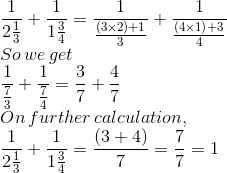9. If 1/3 + 1/2 + 1/x = 4, then x = ?
(a) 5/18
(b) 6/19
(c) 18/5
(d) 24/11

Solution:

The option (b) is correct answer.

It is given that

1/3 + ½ + 1/x = 4

On further calculation

1/x = 4 – 1/3 – 1/2

By taking LCM of 3 and 2 as 6

1/x = 24/6 – 2/6 – 3/6

So we get

1/x = (24 – 2 – 3)/ 6 = 19/6

Hence, x = 6/19

10. If 1/2 + 1/x = 2, then x =
(a) 2/5
(b) 5/2
(c) 3/2
(d) 2/3

Solution:

The option (d) is correct answer.

It is given that

½ + 1/x = 2

On further calculation

1/x = 2 – 1/2

By taking LCM as 2 we get

1/x = 4/2 – 1/2 = (4 – 1)/2 = 3/2

Hence, x = 2/3

11. Which of the following fractions is the smallest?
1/2,3/7,3/5,4/9
(a) 4/9
(b) 3/5
(c) 3/7
(d) 1/2

Solution:

The option (c) is correct answer.

We know that the LCM of numerator is 12

By converting each fraction to an equivalent fraction having 12 as numerator

1/2 = 1/2 × 12/12 = 12/24

3/7 = 3/7 × 4/4 = 12/28

3/5 = 3/5 × 4/4 = 12/20

4/9 = 4/9 × 3/3 = 12/27

We know that if the numerator is same the fraction having larger denominator is the smallest.

Hence, 3/7 is the smallest fraction.

12. Which of the following fractions is the greatest of all?

7/8, 6/7, 4/5, 5/6
(a) 6/7
(b) 4/5
(c) 5/6
(d) 7/8

Solution:

The option (d) is correct answer.

We know that the LCM of 8, 7, 6 and 5 is 840

By converting each fraction to an equivalent fraction having 840 as denominator

7/8 = 7/8 × 105/105 = 735/840

6/7 = 6/7 × 120/120 = 720/840

4/5 = 4/5 × 168/168 = 672/840

5/6 = 5/6 × 140/140 = 700/840

We know that if the denominator is same the fraction having larger numerator is the greatest.

Hence, 7/8 is the greatest fraction.

13. What is the value of a+b/ a−b, If a/b=4?
(a) 3/5
(b) 5/3
(c) 4/5
(d) 5/4

Solution:

The option (b) is correct answer.

It is given that a/b = 4

We can write it as a = 4b

By substituting the value of a in a+b/a-b

a+b/a-b = 4b+b/4b-b = 5b/3b

Dividing numerator and denominator by b, the value is 5/3.

14. If a/b = 4/3, then the value of 6a+4b/ 6a-5b is
(a) −1
(b) 3
(c) 4
(d) 5

Solution:

The option (c) is correct answer.

It is given that a/b = 4/3

We can write it as a = 4b/3

By substituting the value of a in 6a+4b/6a-5b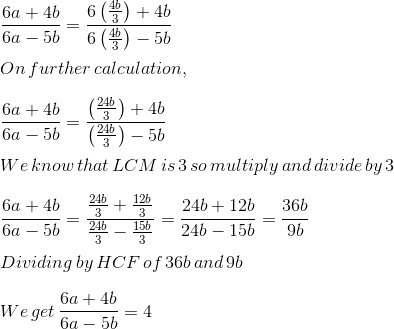15. If 1/5 – 1/6 = 4/x, then x =
(a) −120
(b) −100
(c) 100
(d) 120

Solution:

The option (d) is correct answer.

It is given that

1/5 – 1/6 = 4/x

LCM of 5 and 6 is 30

4/x = 6/30 – 5/30

On further calculation

4/x = 1/30

So we get

x = 4 (30) = 120

16. The fraction to be added to 6 7/15 to get 8 1/5 is equal to
(a) 11/15
(b) 1 1/15
(c) 44/3
(d) 3/44

Solution:

The option (b) is correct answer.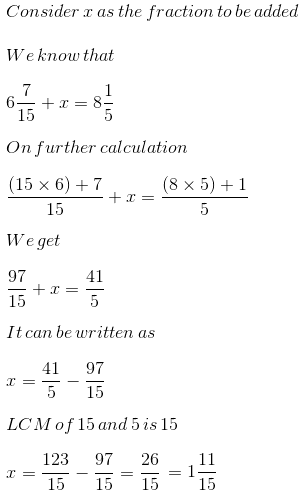17. If 45/60 is equivalent to 3/x, then x =
(a) 5
(b) 4
(c) 6
(d) 20

Solution:

The option (b) is correct answer.

It is given that

45/60 = 3/x

By cross multiplication

45 × x = 3 × 60

It can be written as

x = (3 × 60)/45 = 180/45

Dividing the fraction by HCF

(180 ÷ 45)/(45÷45) = 4

18. A fraction equivalent to 45/105 is
(a) 6/14
(b) 4/7
(c) 5/7
(d) 7/5

Solution:

The option (a) is correct answer.

The given fraction is 45/105

By dividing the numerator and denominator with the HCF

(45 ÷ 15)/(105÷15) = 3/7

On further calculation

3/7 = 3/7 × 2/2 = 6/14

19. 5/8 + 3/4 – 7/12 is equal to
(a) 15/24
(b) 17/24
(c) 19/24
(d) 21/24

Solution:

The option (c) is correct answer.

The given fraction is

5/8 + 3/4 – 7/12

We know that the LCM is 24

= (5 × 3)/ (8 × 3) + (3 × 6)/ (4 × 6) – (7 × 2)/ (12 × 2)

On further calculation

= 15/24 + 18/24 – 14/24

So we get

= 19/24

20. The correct fraction in the box □ is □ – 5/8=1/4
(a) 6/8
(b) 7/8
(c) 1/2
(d) None of these

Solution:

The option (b) is correct answer.

The given equation is

□ – 5/8 = 1/4

It can be written as

□ = 1/4 + 5/8

We know that the LCM is 8

□ = 2/8 + 5/8 = 7/8

### RD Sharma Solutions for Class 6 Maths Chapter 6: Fractions

Chapter 6, Fractions, has 9 exercises which explains the methods used in solving problems along with diagrammatic representation. The Chapter 6 of RD Sharma Solutions contains some of the basic concepts like:

• Fraction
• Representation of fractions on the number line
• Fraction as division
• Types of fractions
• Equivalent fractions
• Fractions in lowest terms
• Like and Unlike Fractions
• Comparing and ordering of fractions
• Addition and Subtraction of like fractions
• Addition and subtraction of unlike fractions

### Chapter Brief of RD Sharma Solutions for Class 6 Maths Chapter 6 – Fractions

Concepts which are explained in Class 6 are important as they are continued in higher classes as well. This Chapter contains methods of solving types of fractions using operations. The solutions are prepared by subject matter experts in order to improve conceptual knowledge and logical thinking abilities among students.

Some of the applications of fractions in our daily life are ingredients used for cooking and their proportion, portion of painted wall and the amount of sugar in the vessel etc. The PDF can be downloaded to get further information regarding the concepts which are explained in this Chapter.